# Lakhmir Singh Solutions of Class 10 Chemistry Chapter 3 Metals and Non-Metals

Based on the properties, elements have been divided into two; Metals and Non- Metals. They have an important part in our daily life. Both metals and non-metals are useful in different ways. There is a large number of metals and non-metals in the world.

Metals are elements that can conduct electricity and are malleable and ductile. They are hard and shining in nature. Examples are iron, aluminium, copper, silver and gold. It is widely used in our daily life for a large number of purposes. It is used for making cooking utensils, buses, cars, etc.

Non-metals are good conductors of electricity. They are neither malleable nor ductile and are also brittle. Examples are carbon, sulphur phosphorus, etc. Carbon has two allotropes, diamond and graphite. Diamond is used as jewellery and graphite is used in pencil leads.

All metals and non-metals have similar properties. But, the properties of non-metals are opposite those of metals. This chapter deals with the properties, reactivities and basic examples of metals and non-metals.

## Download PDF Of Lakhmir Singh Solutions for Class 10 Chemistry Chapter 3 Metals and Non-Metals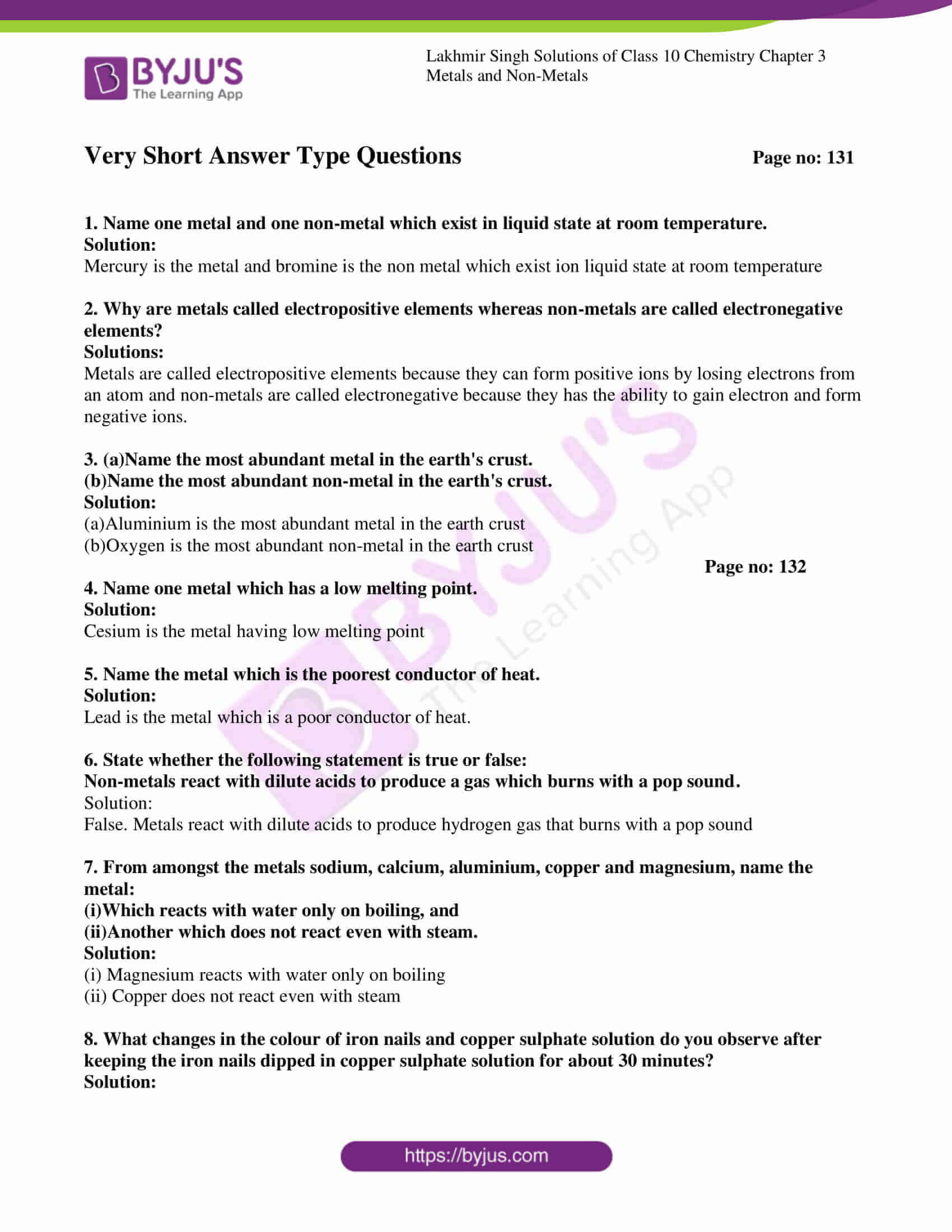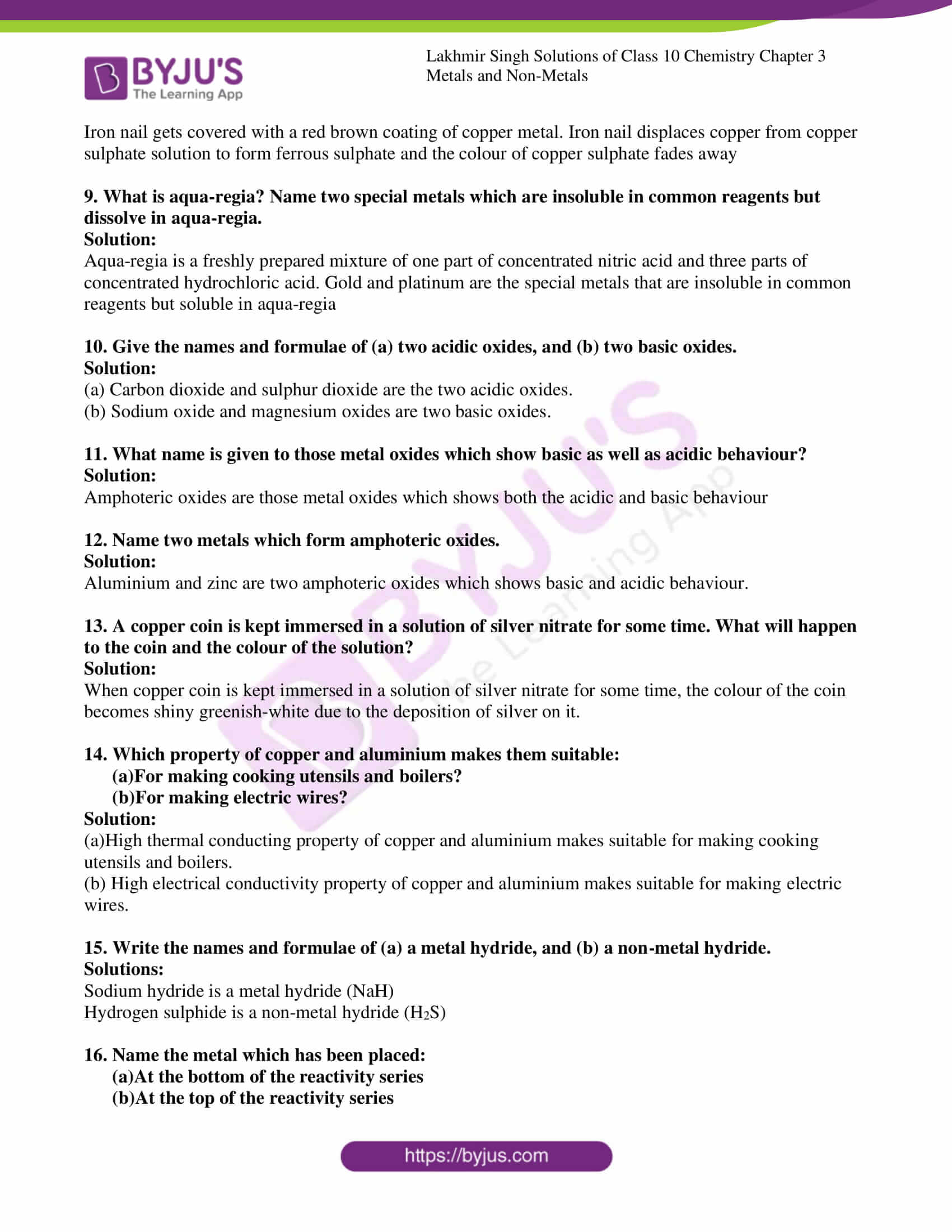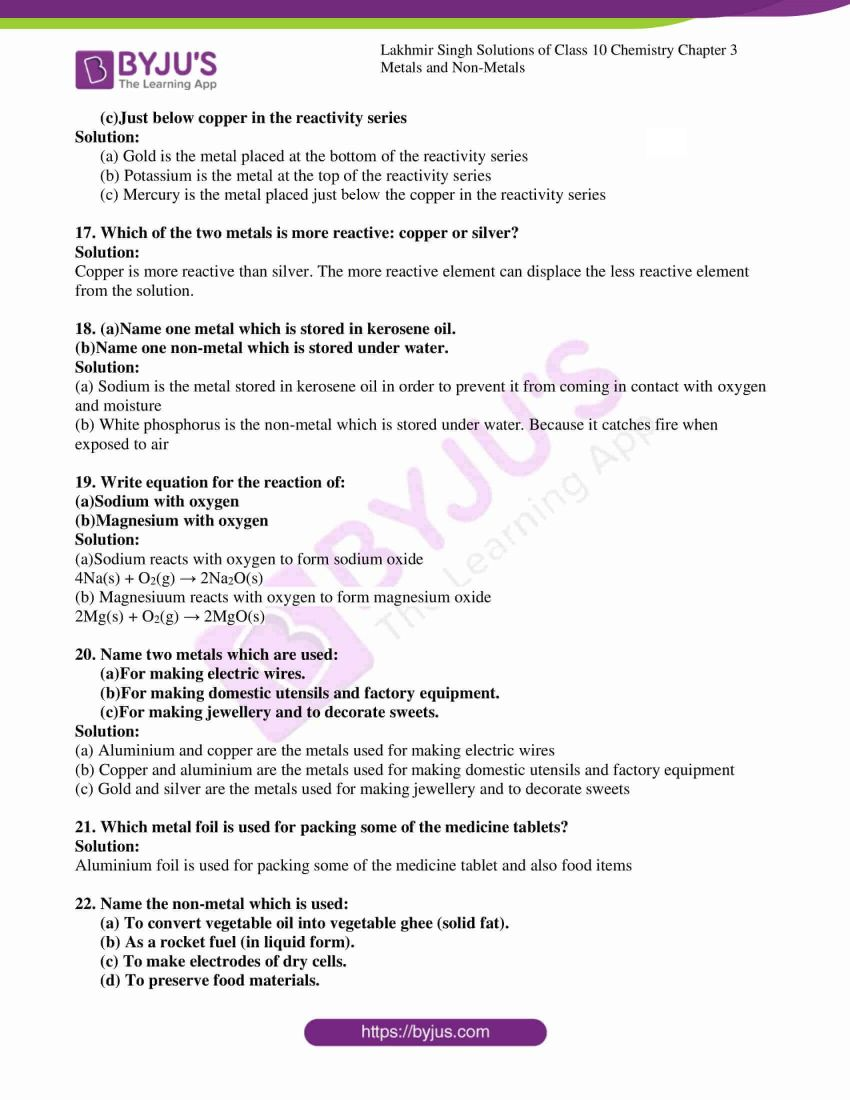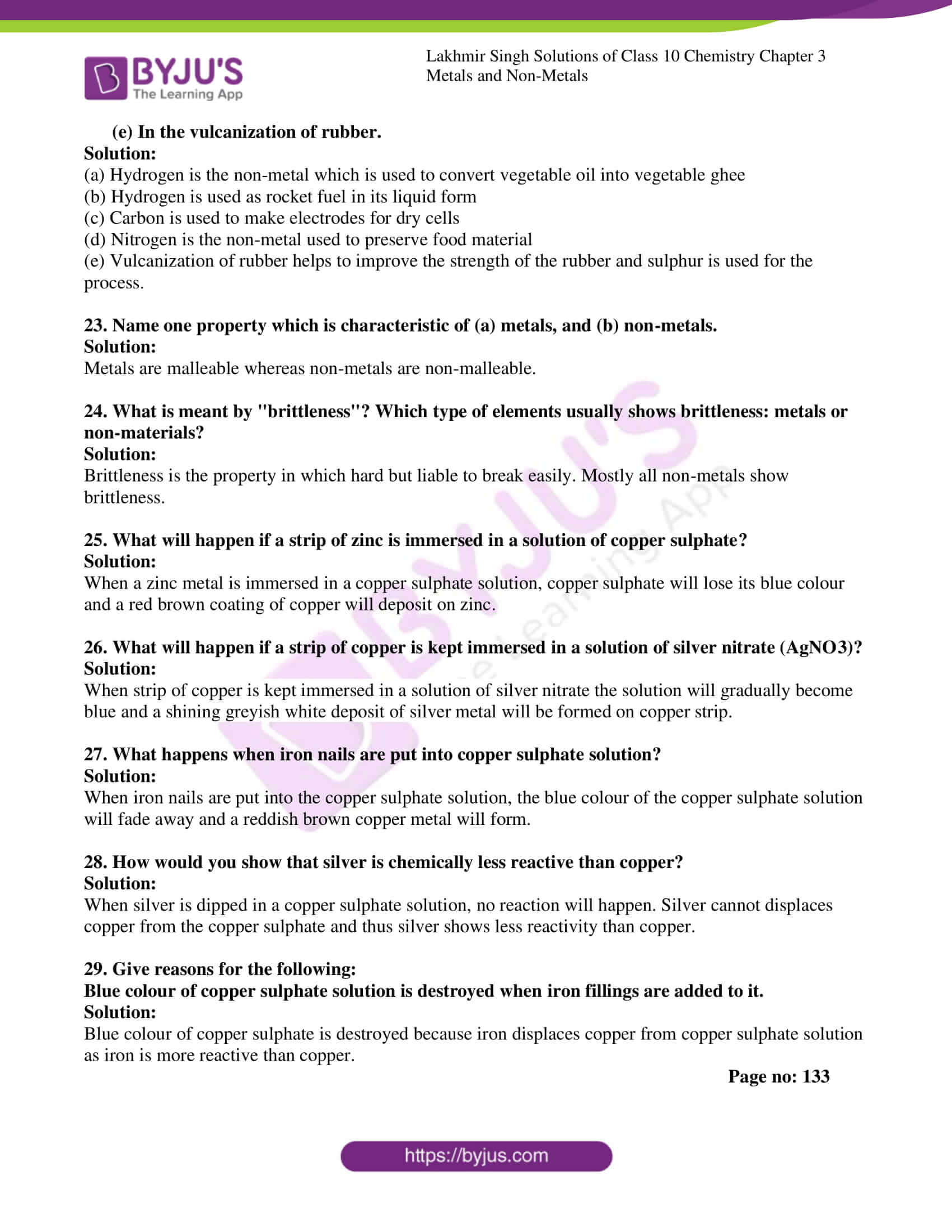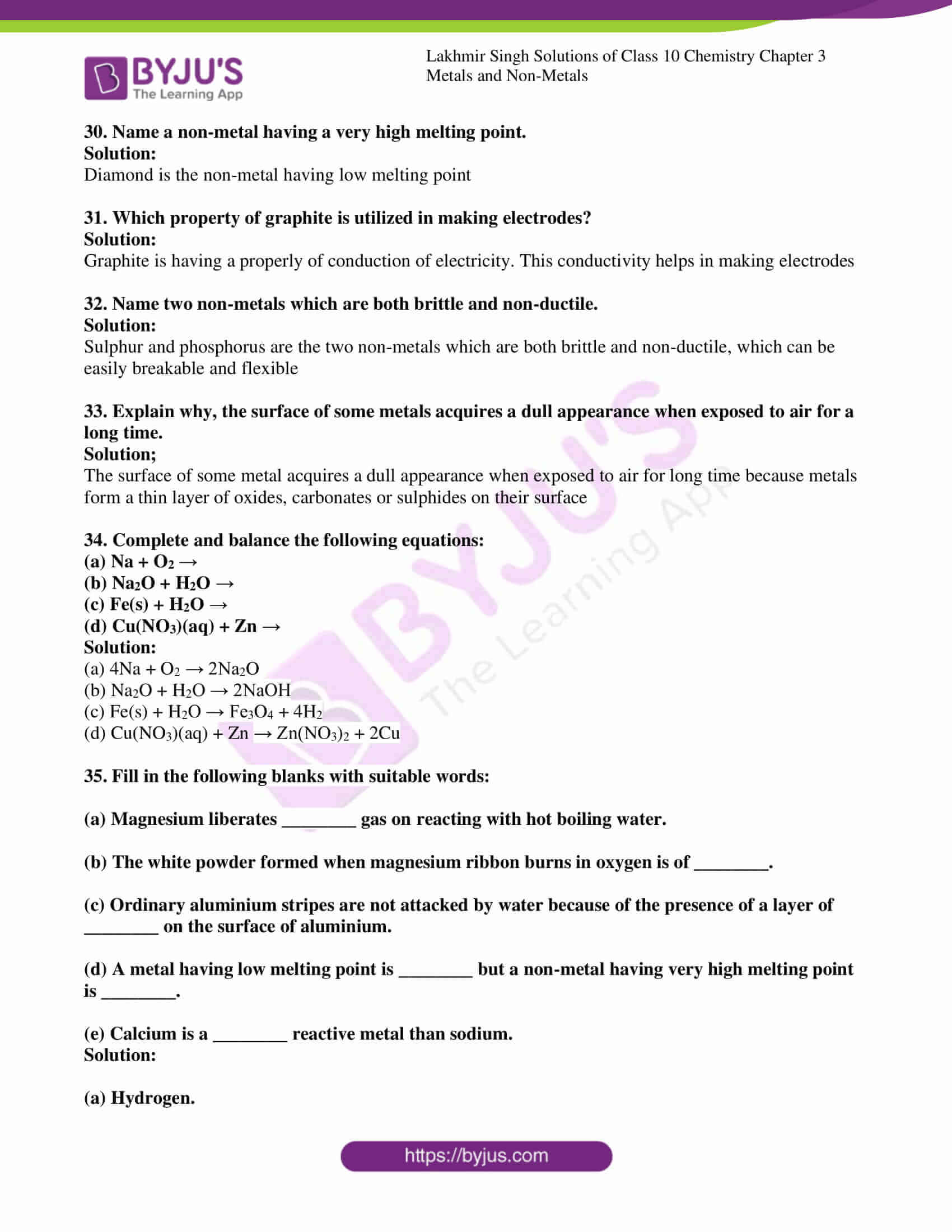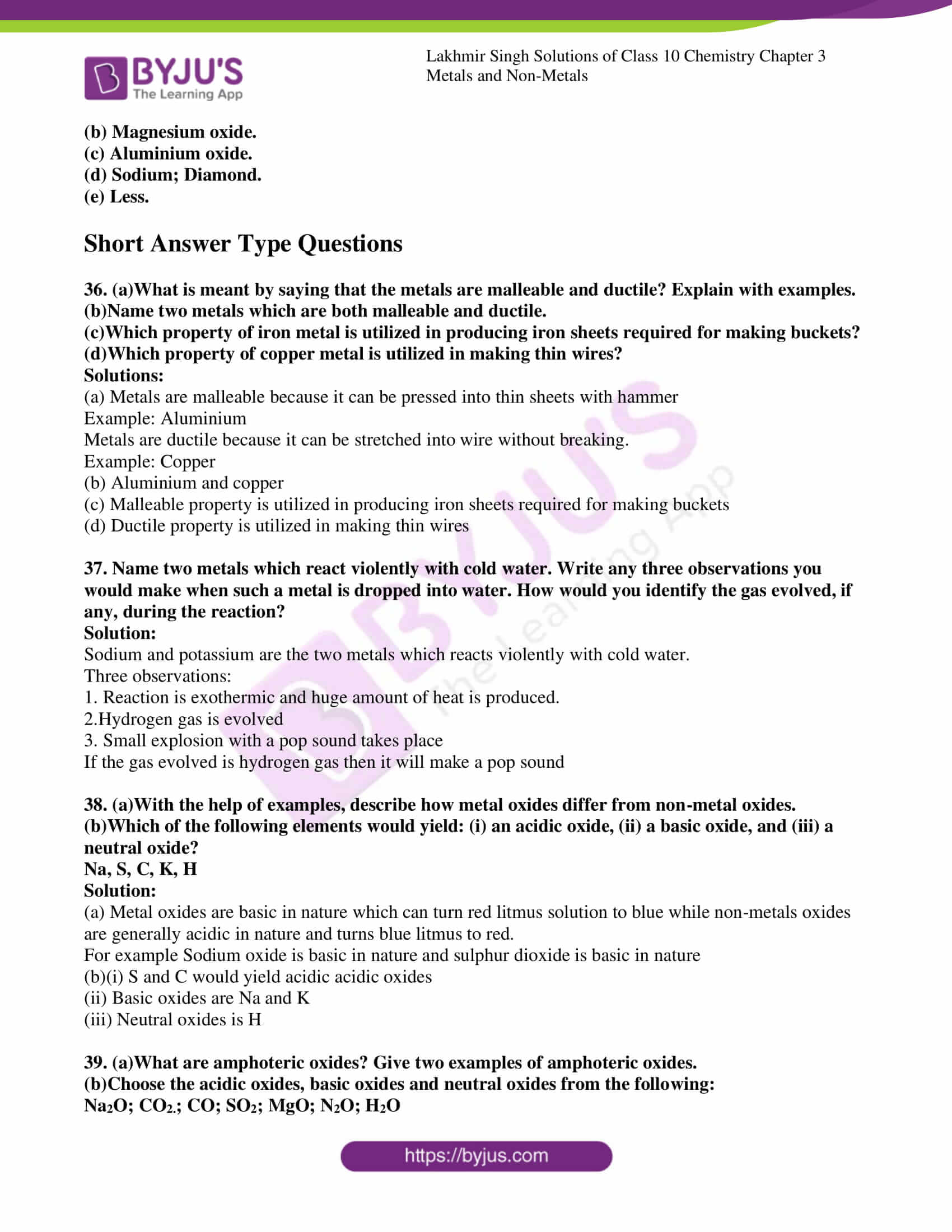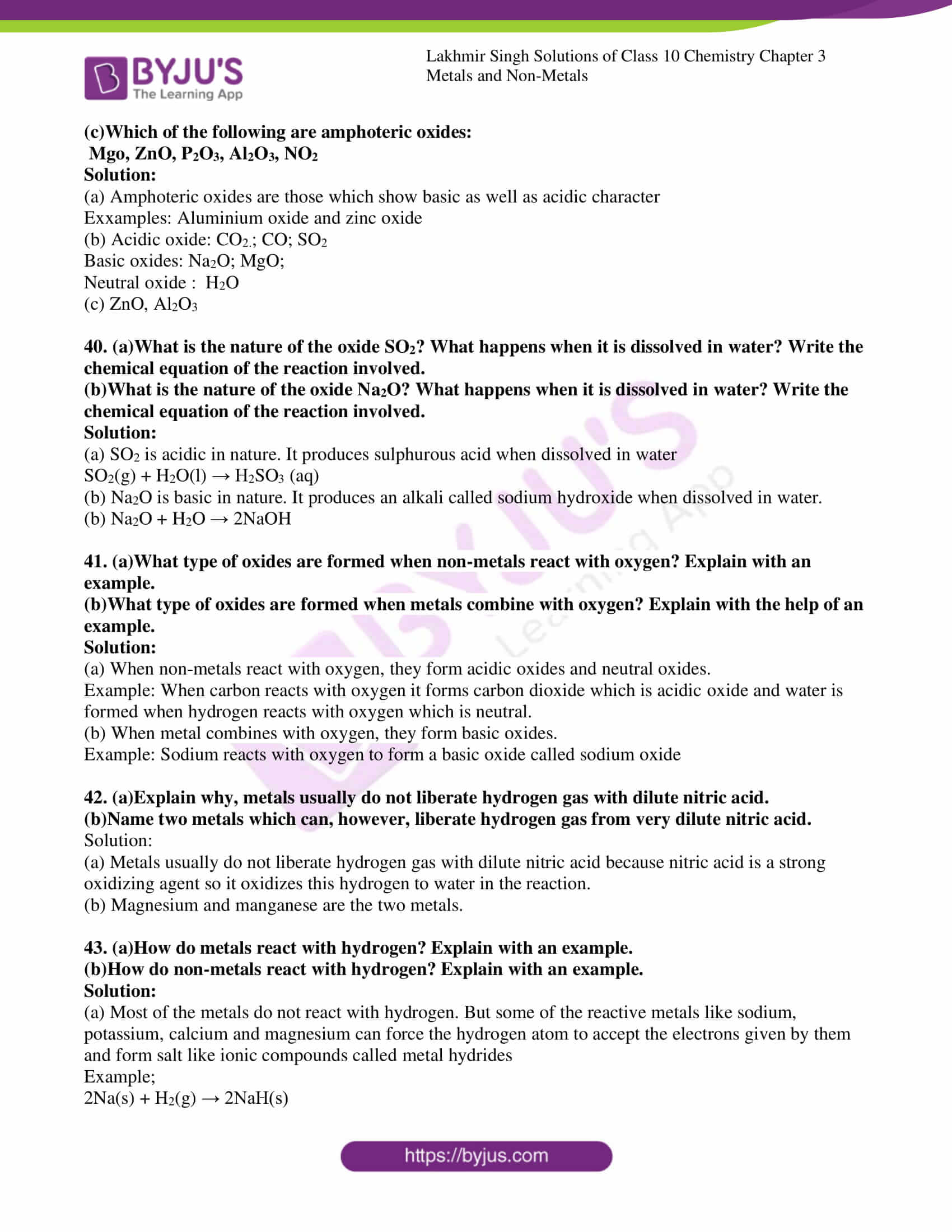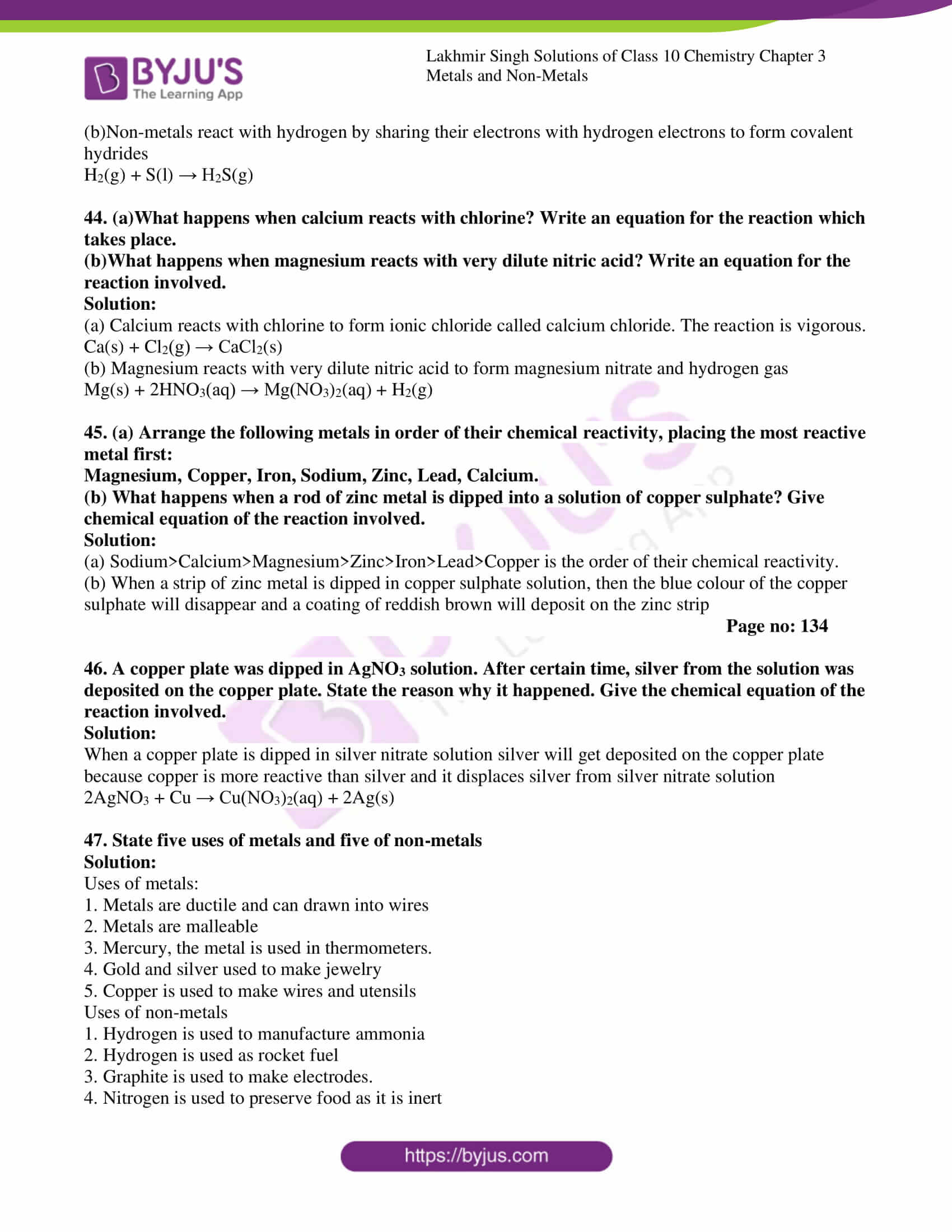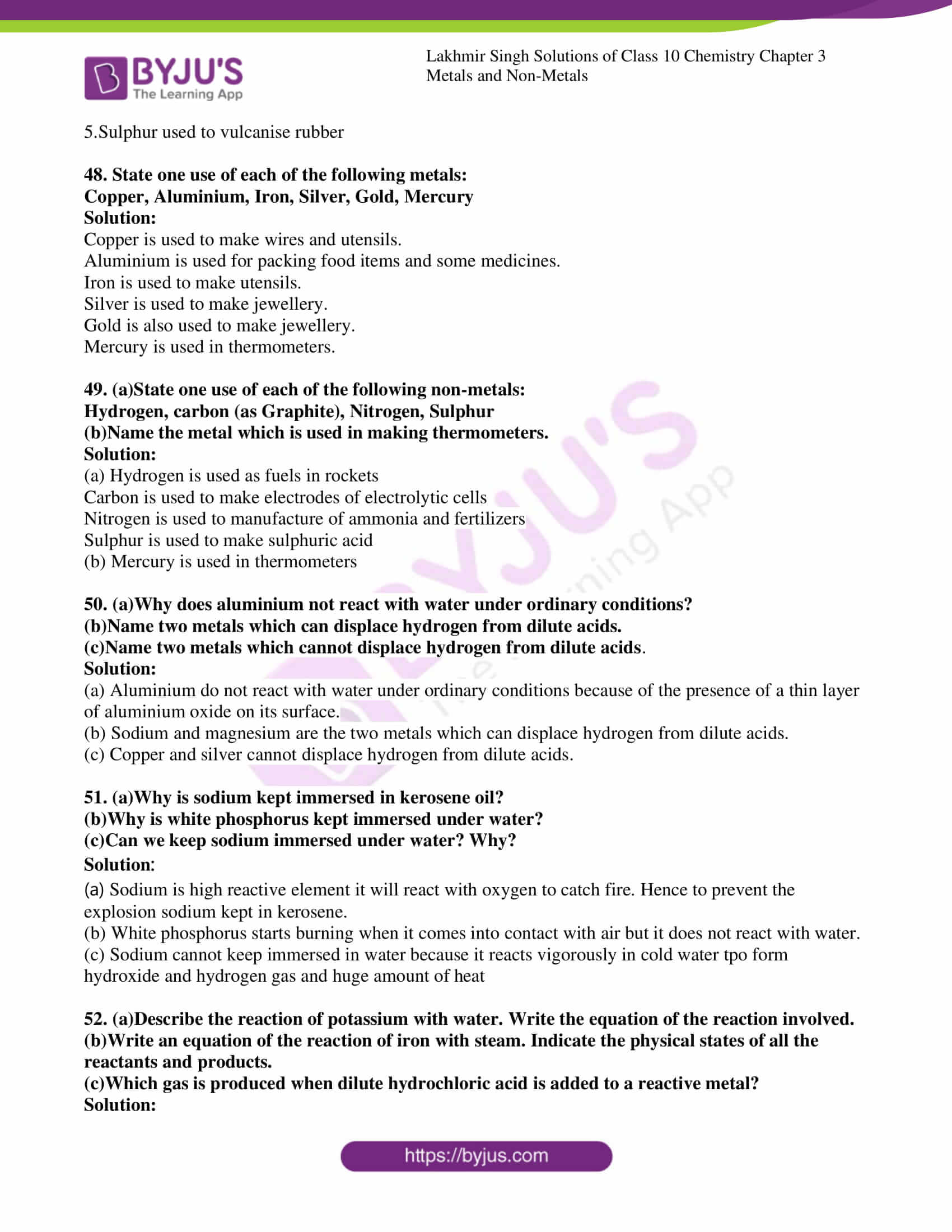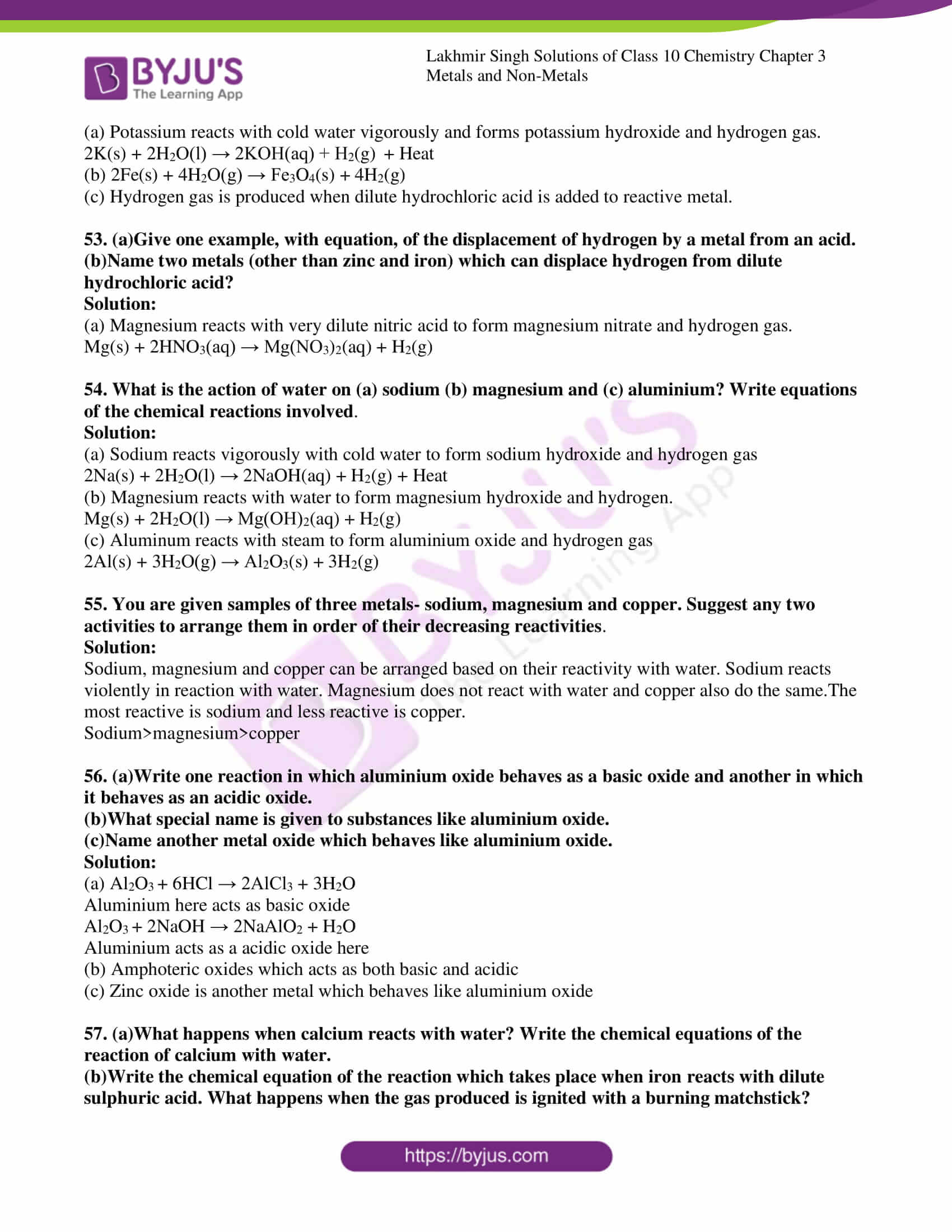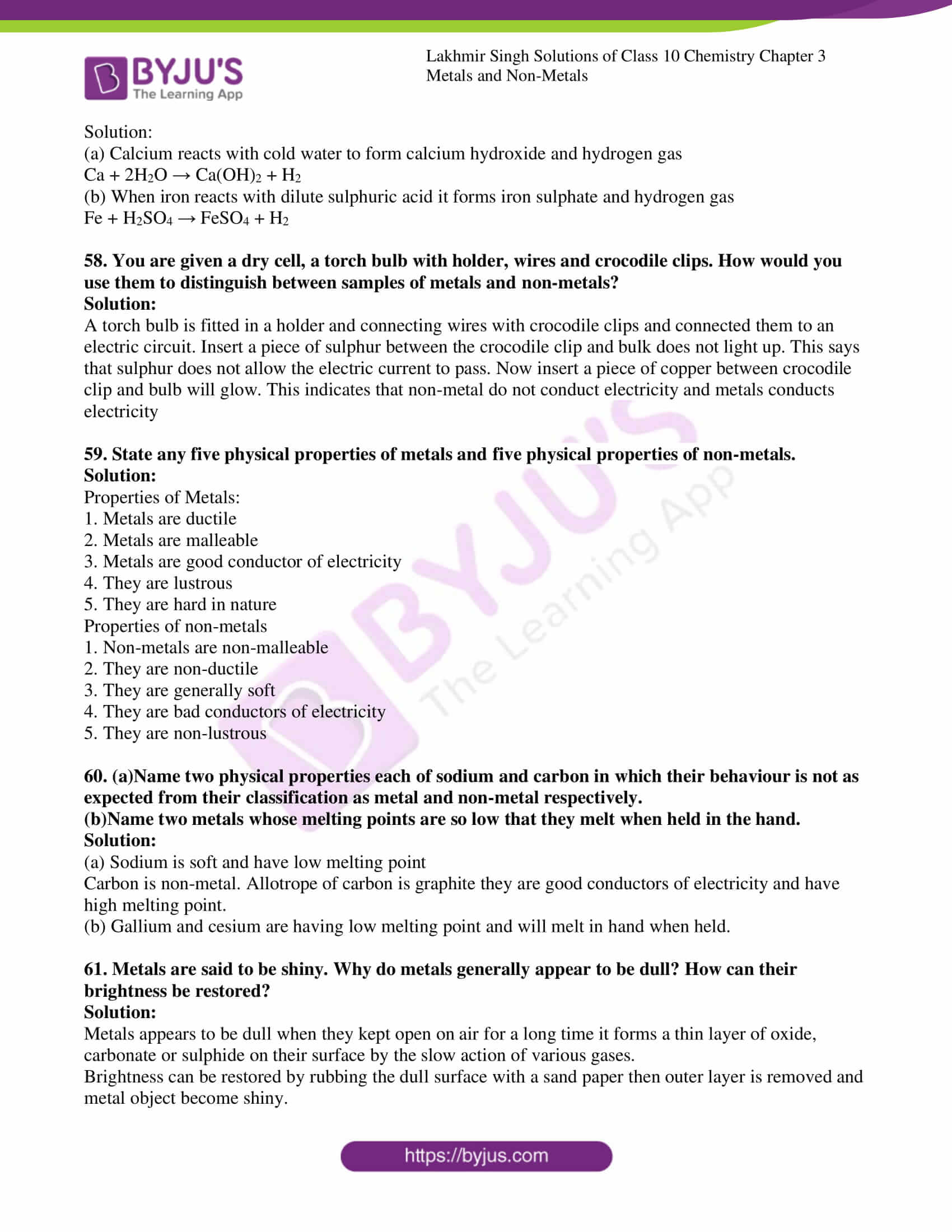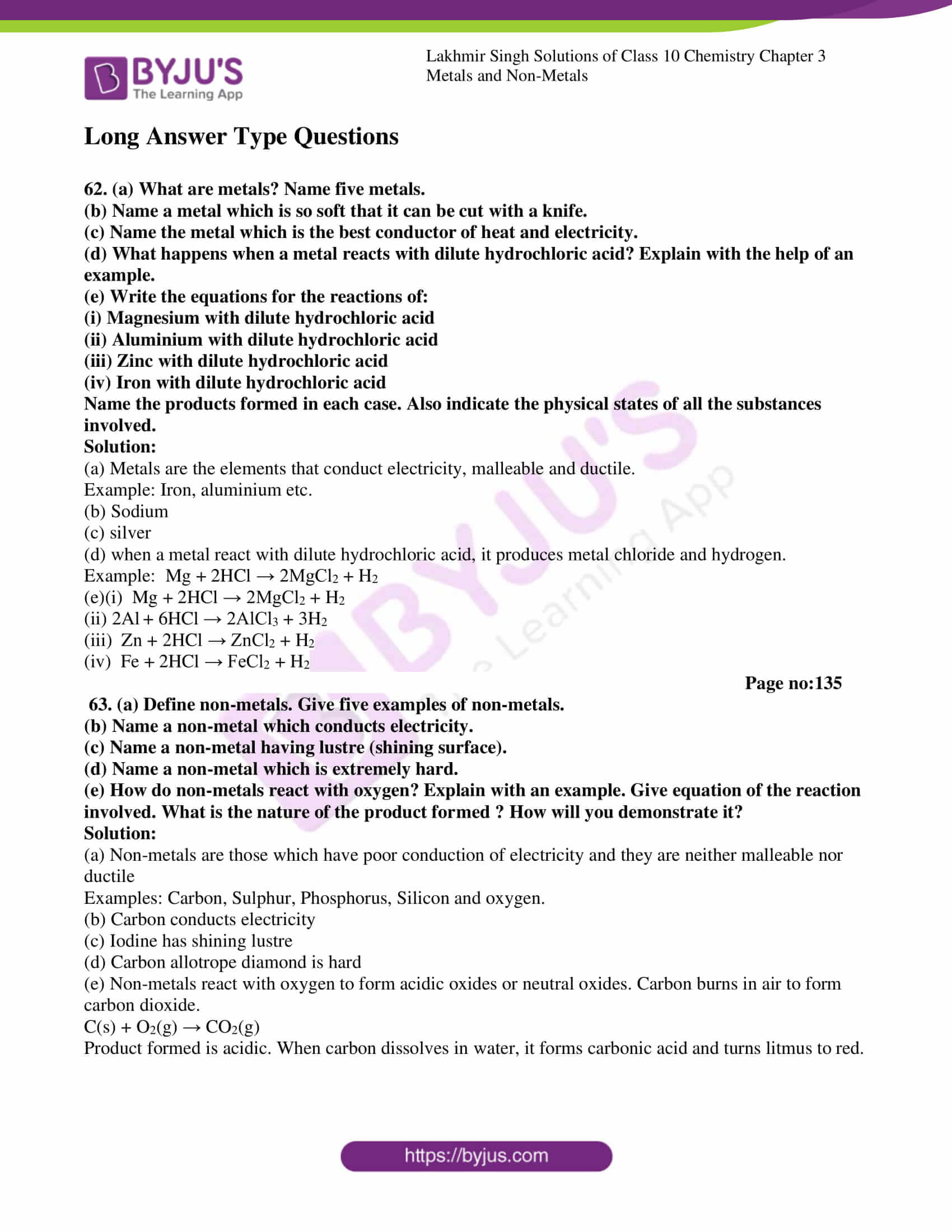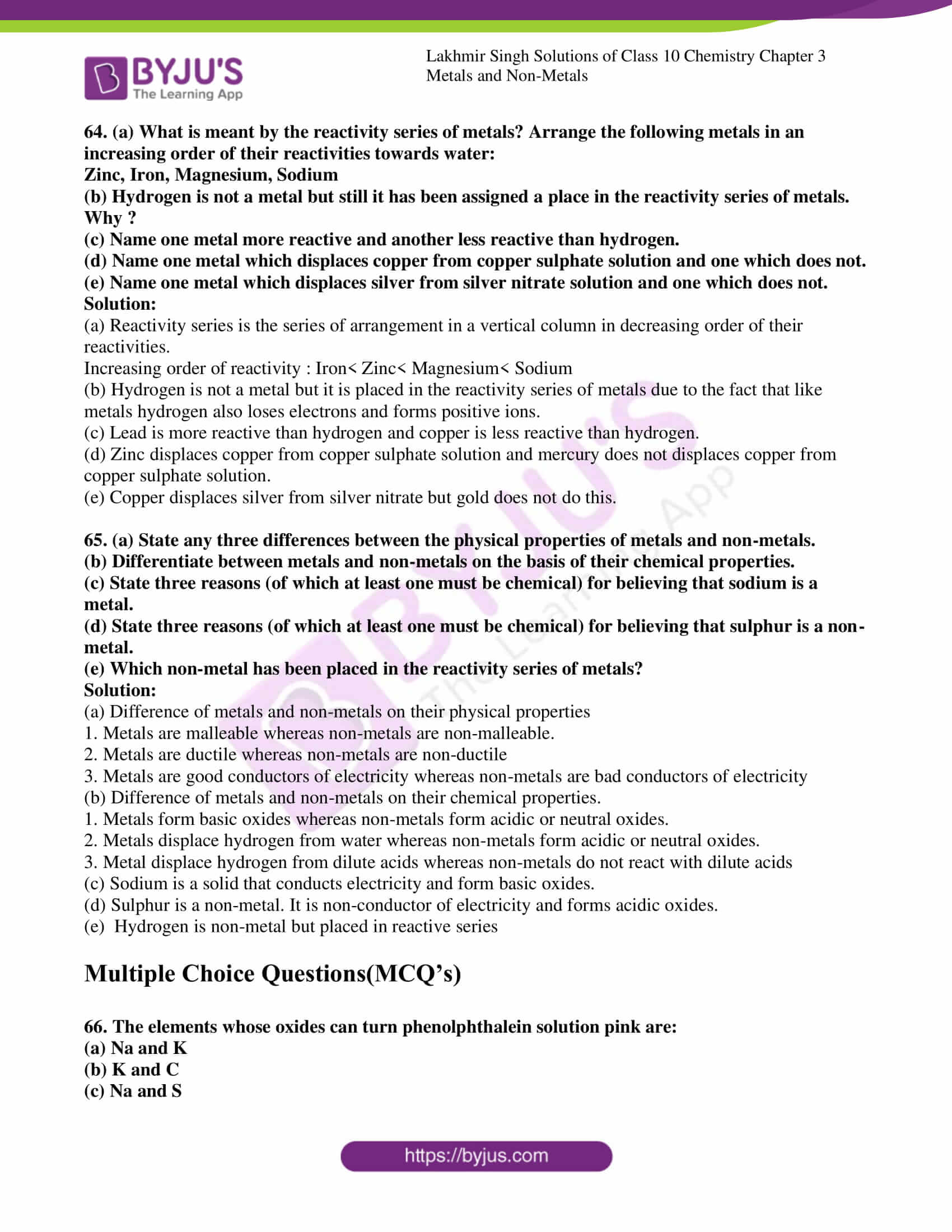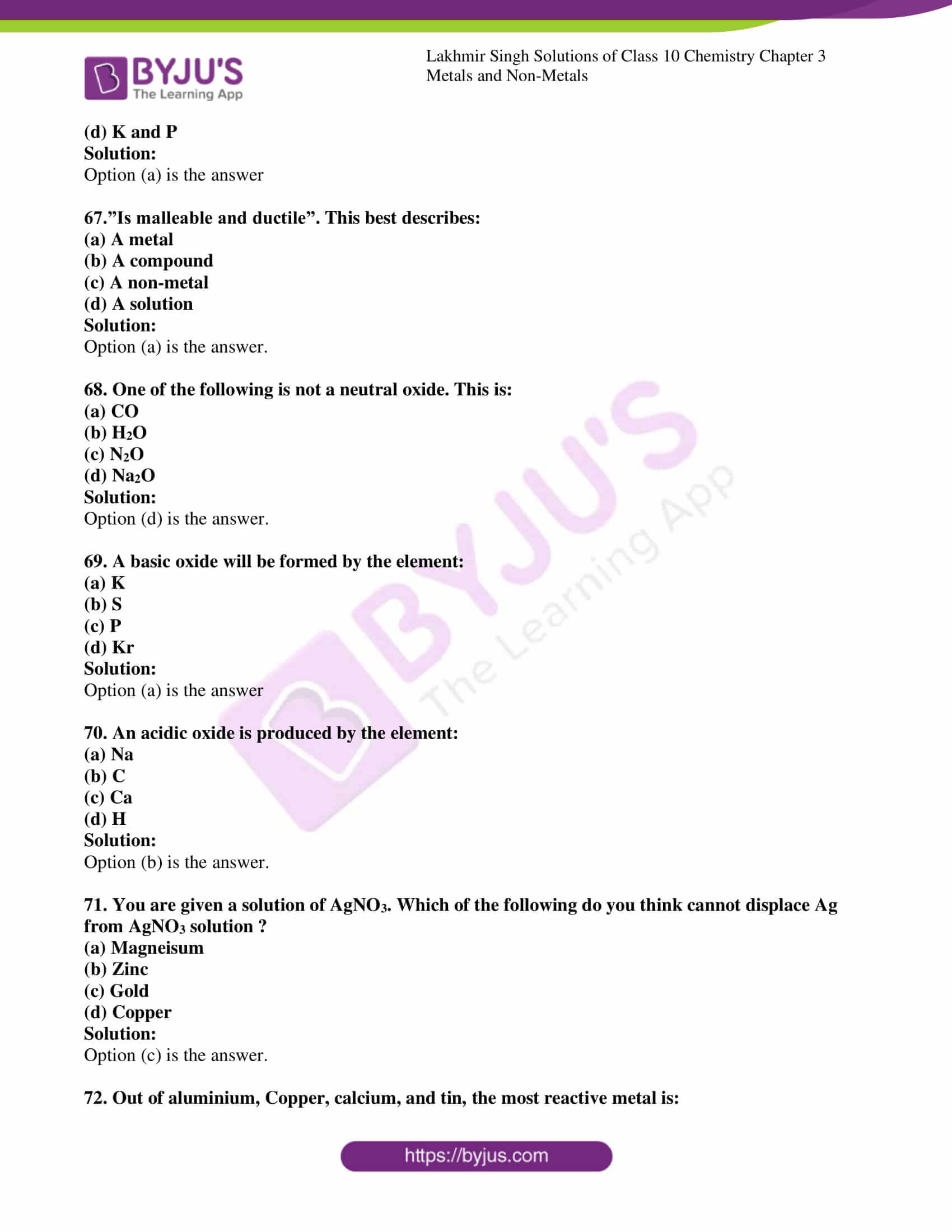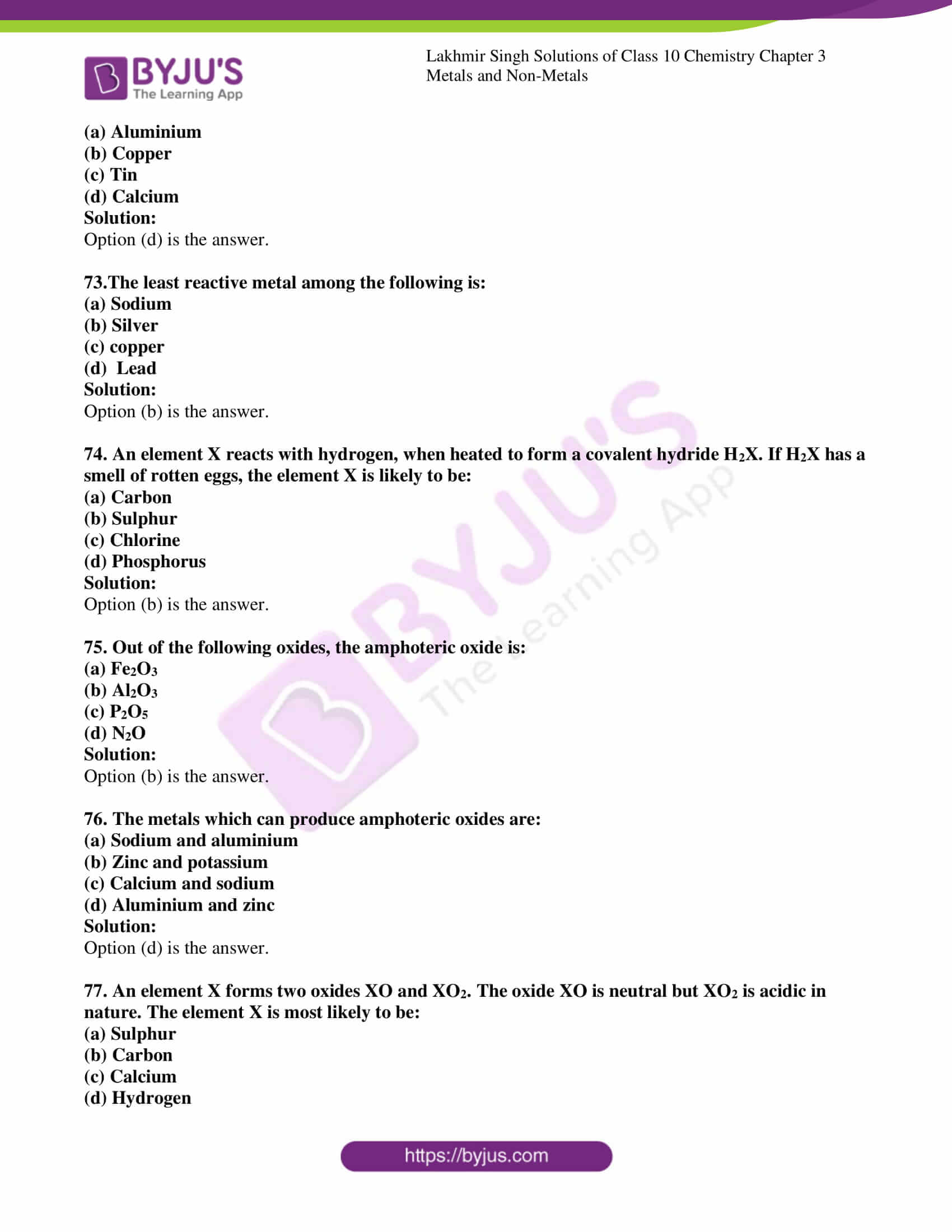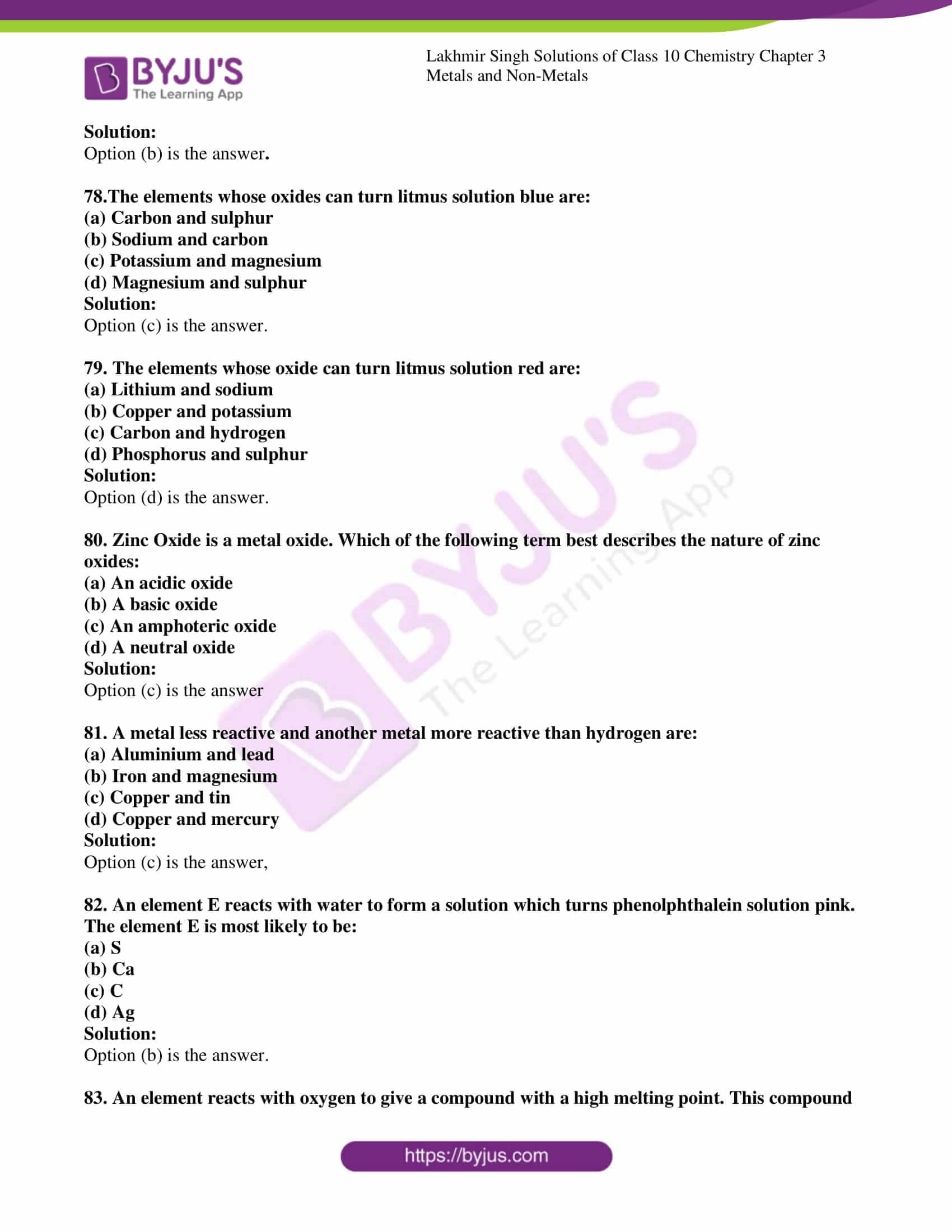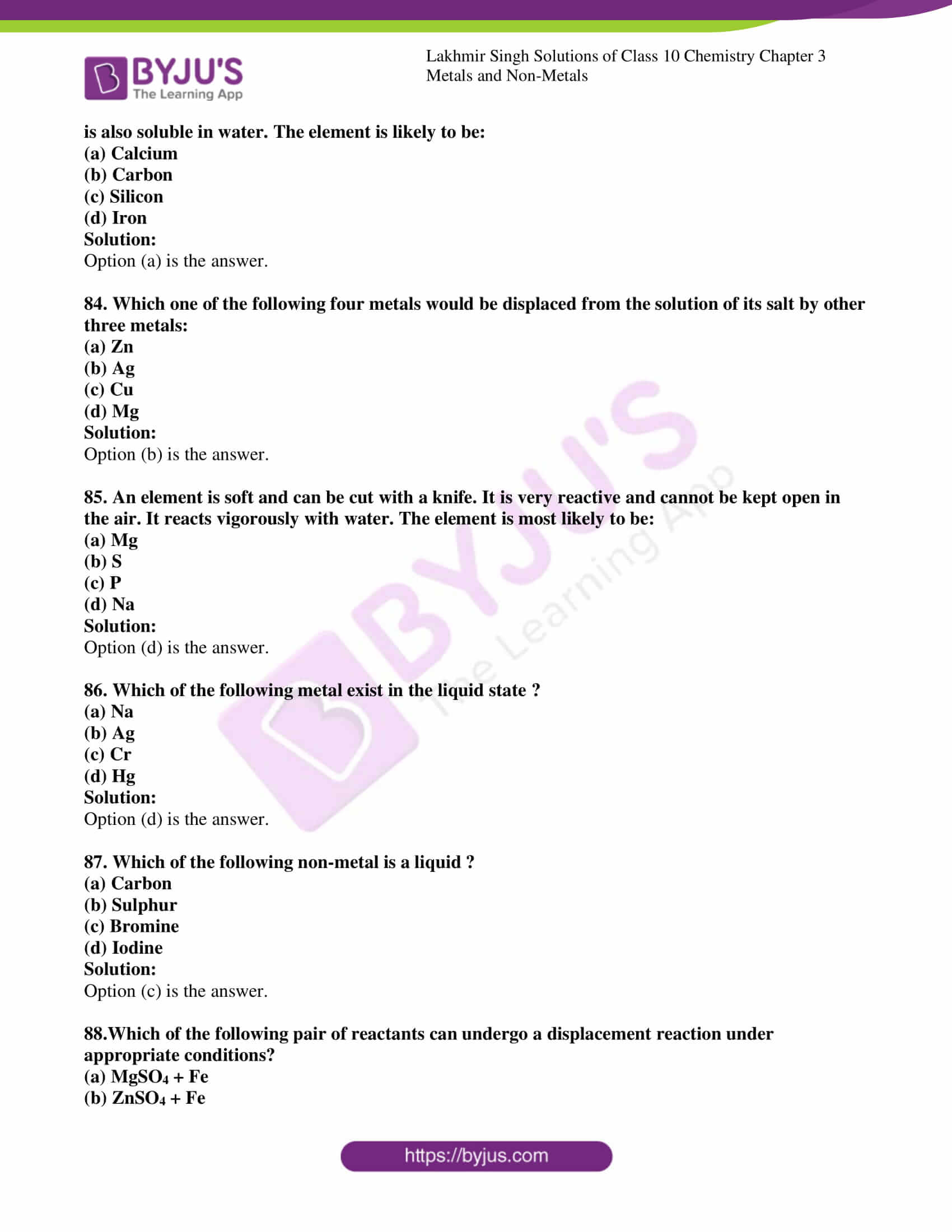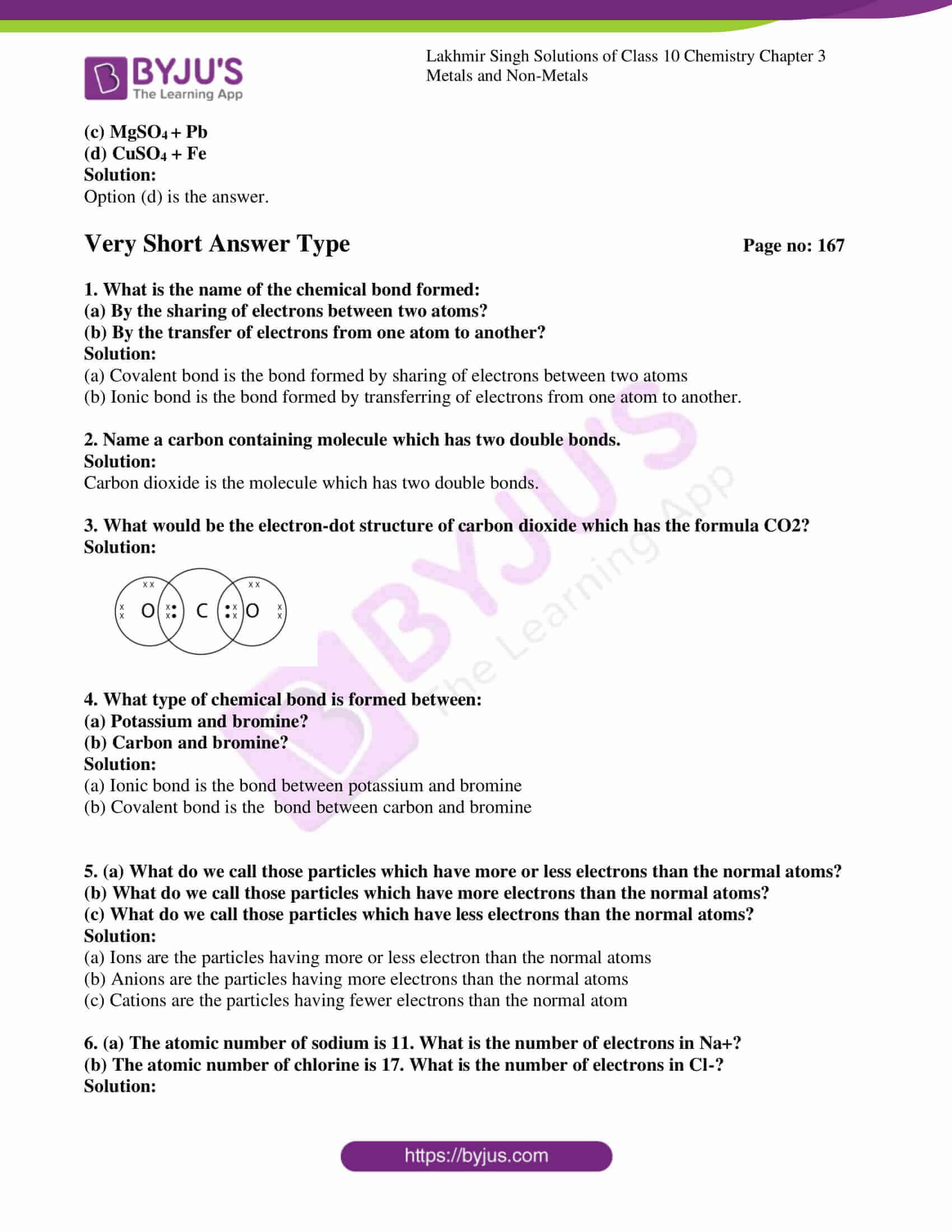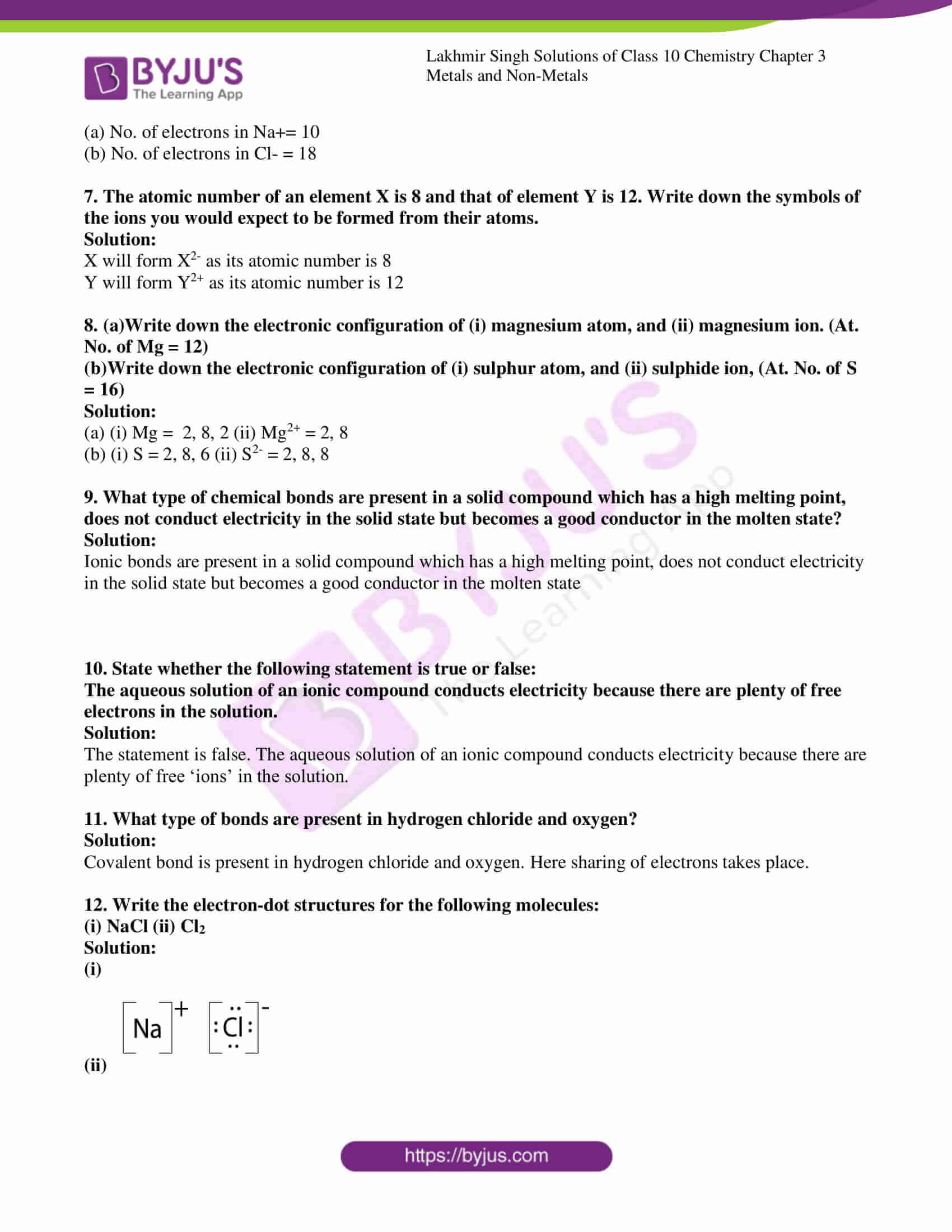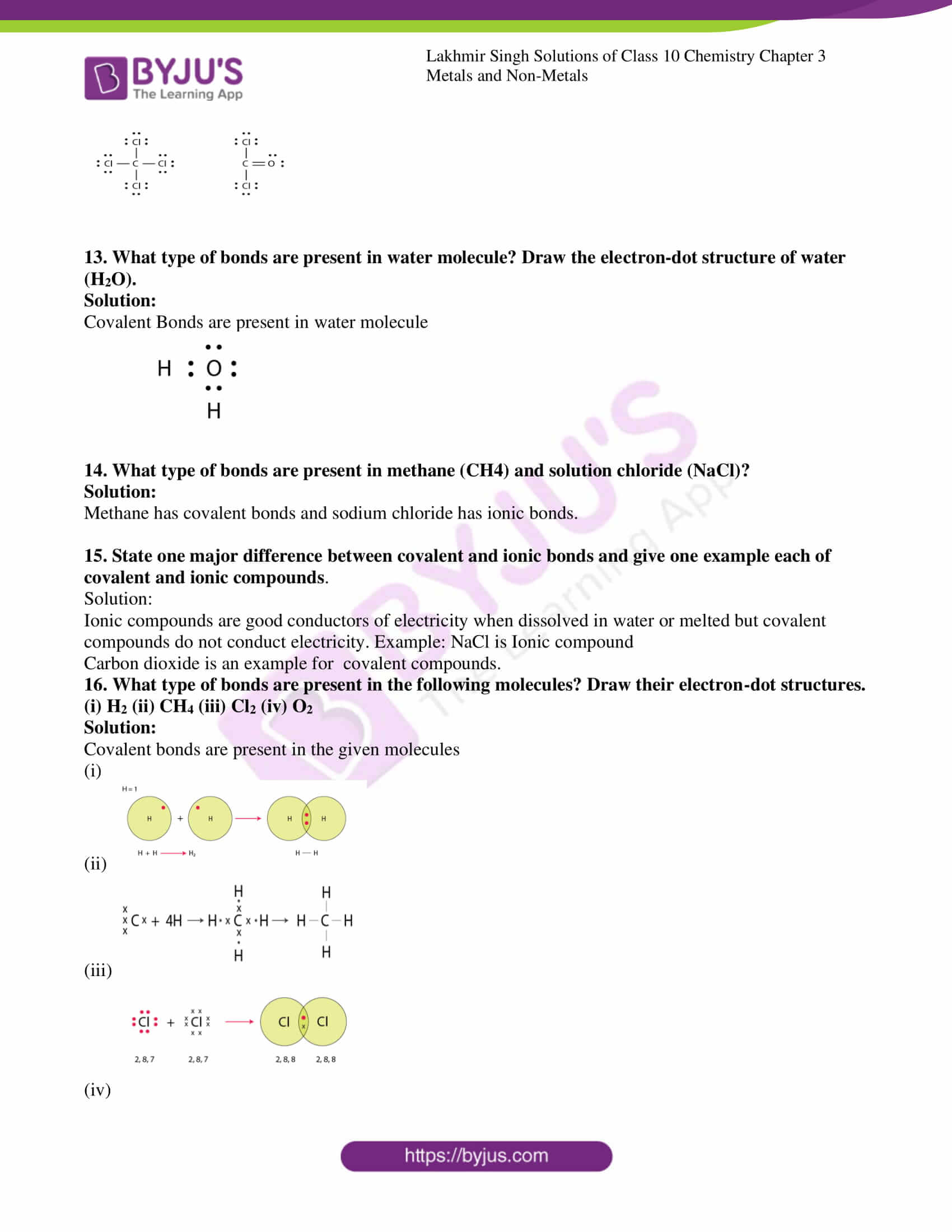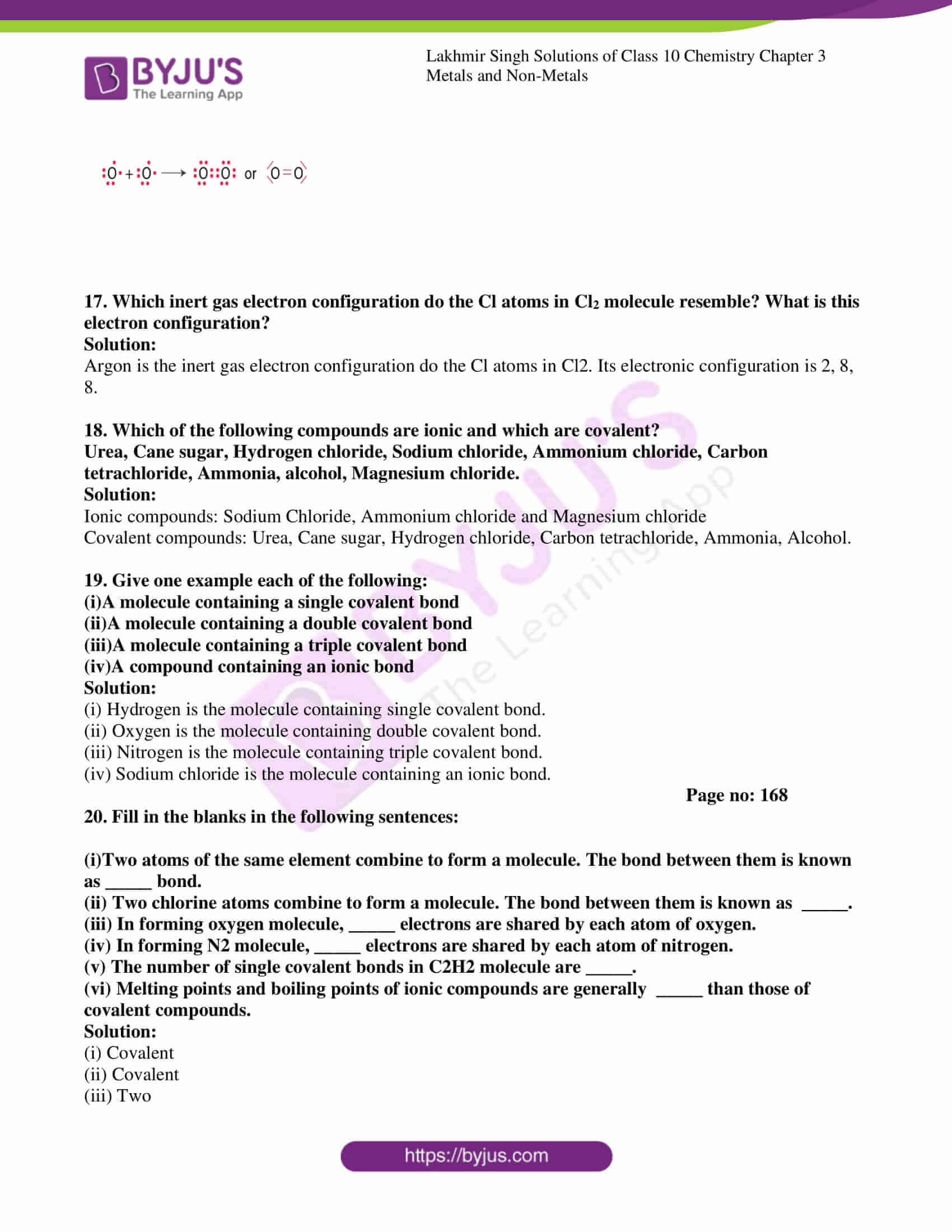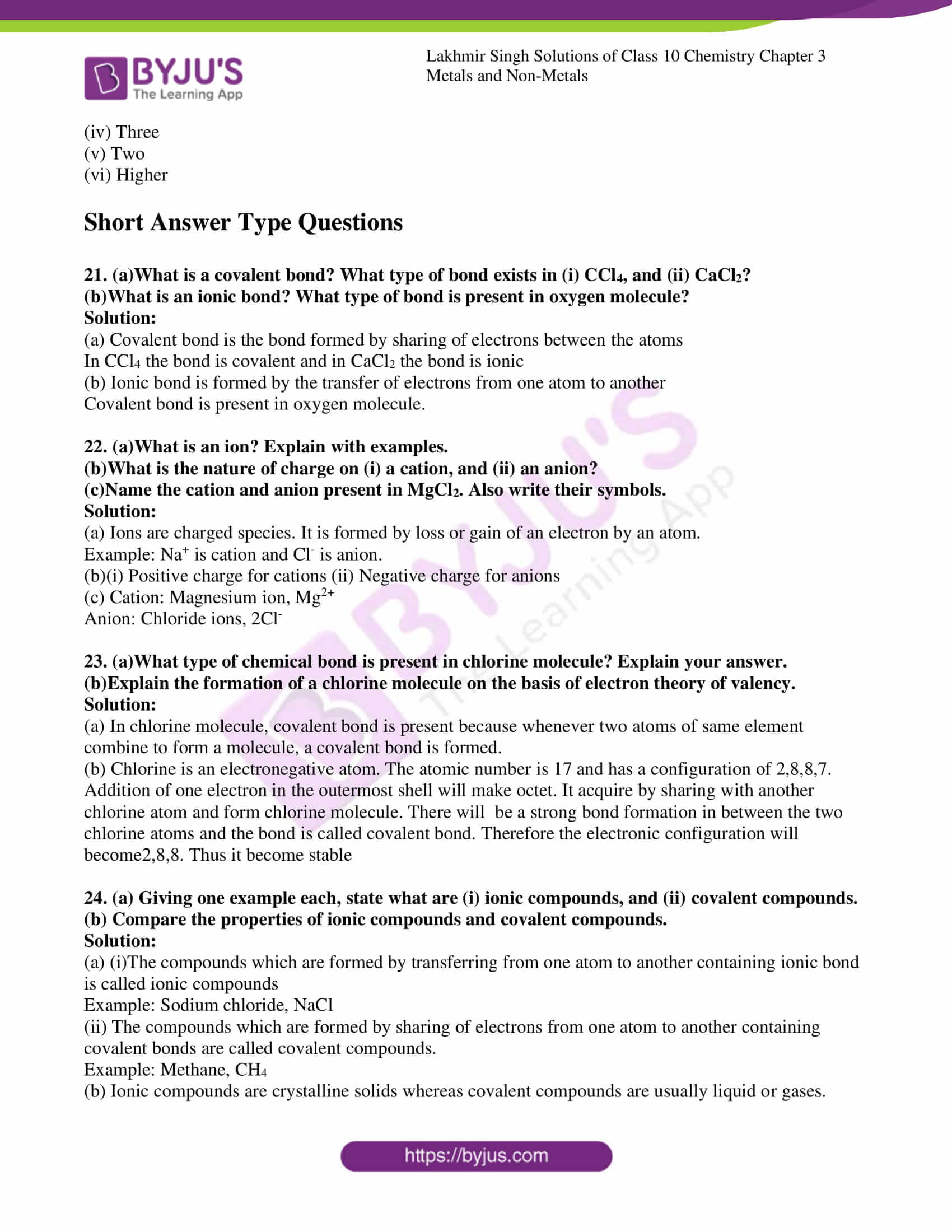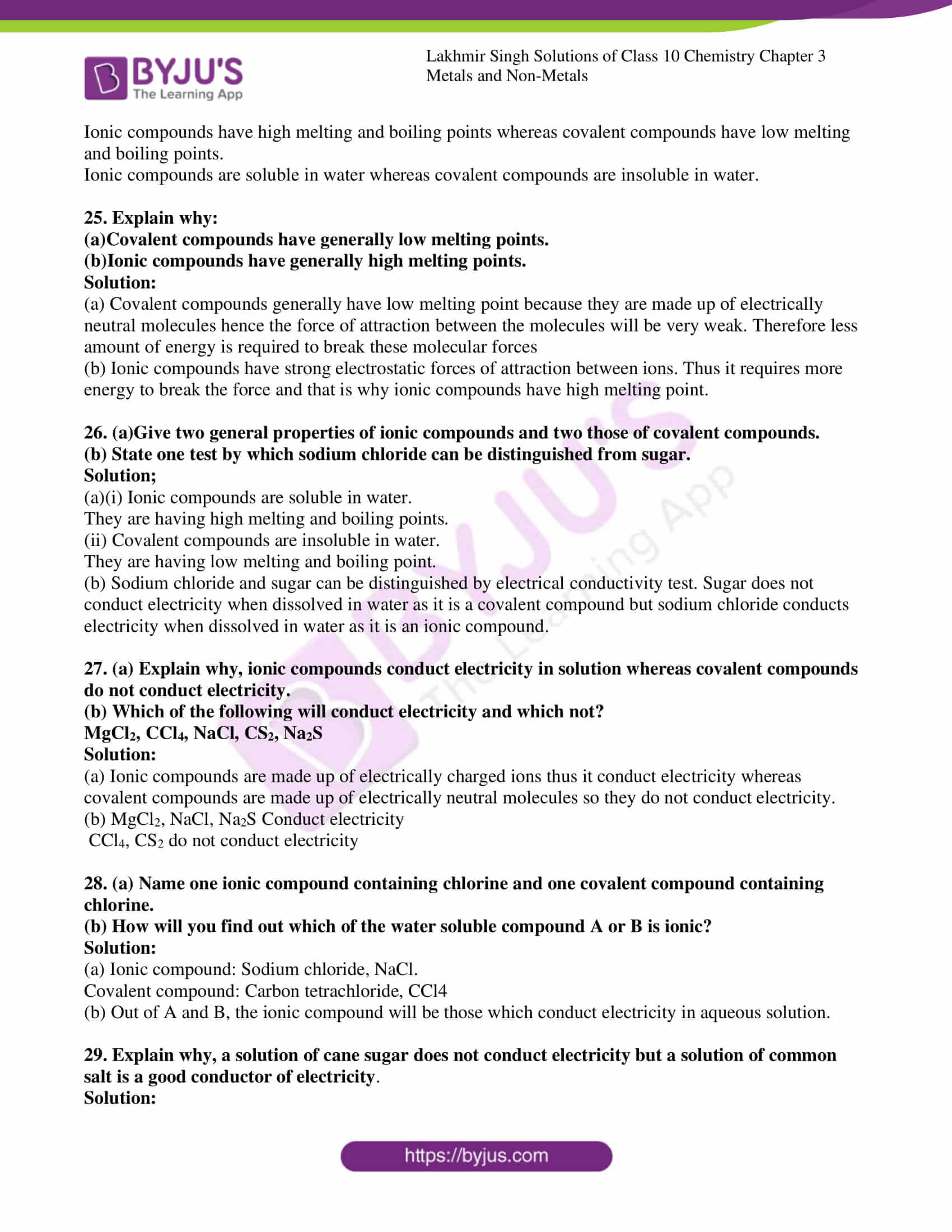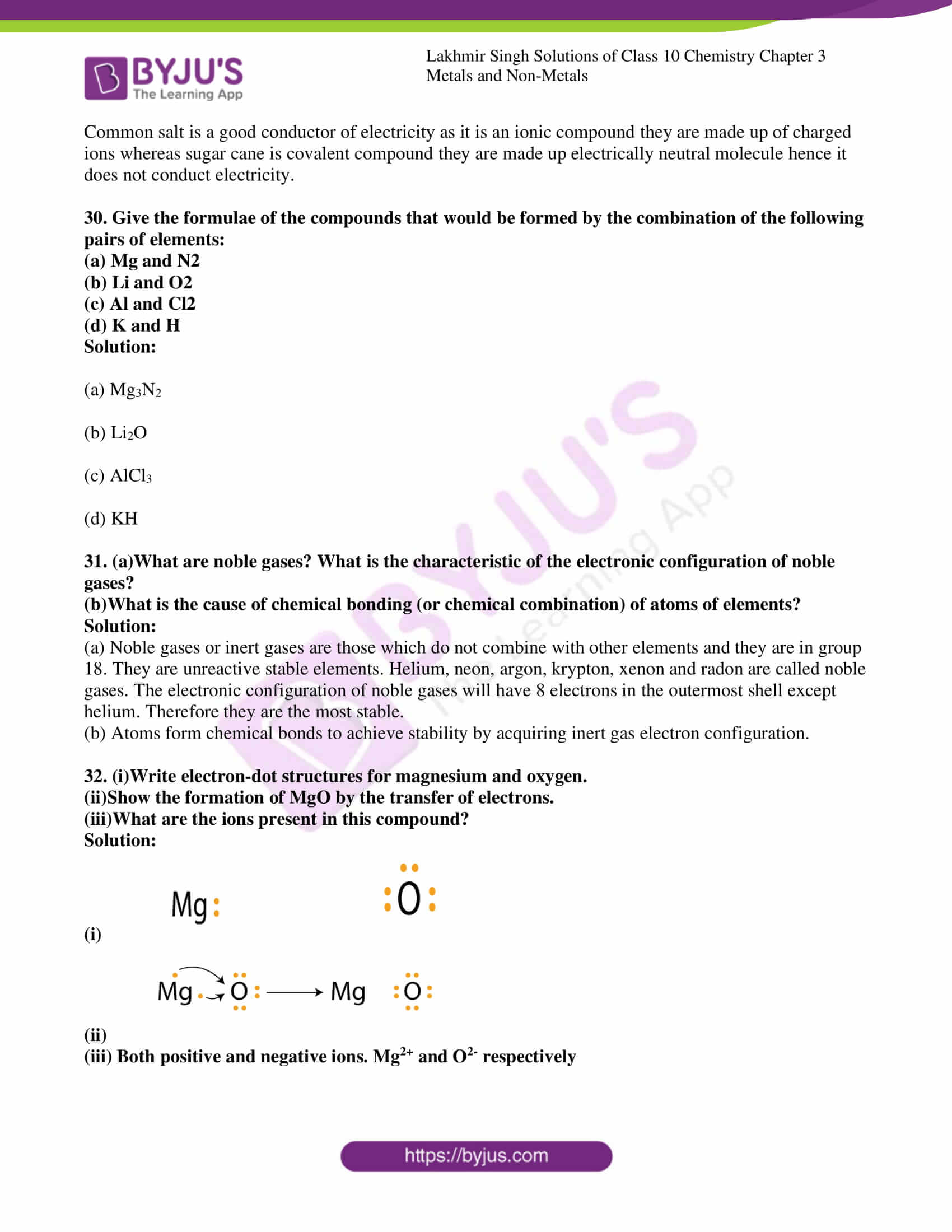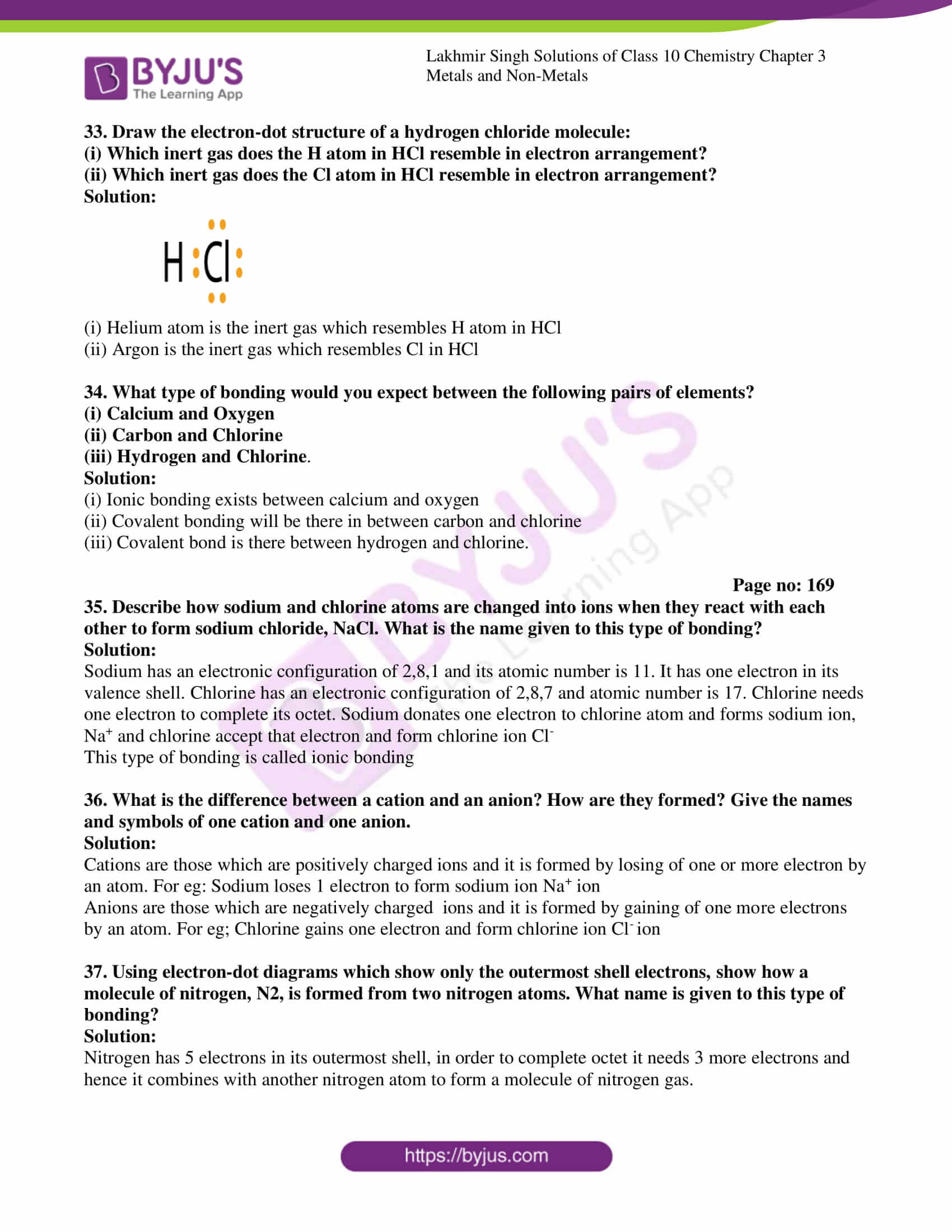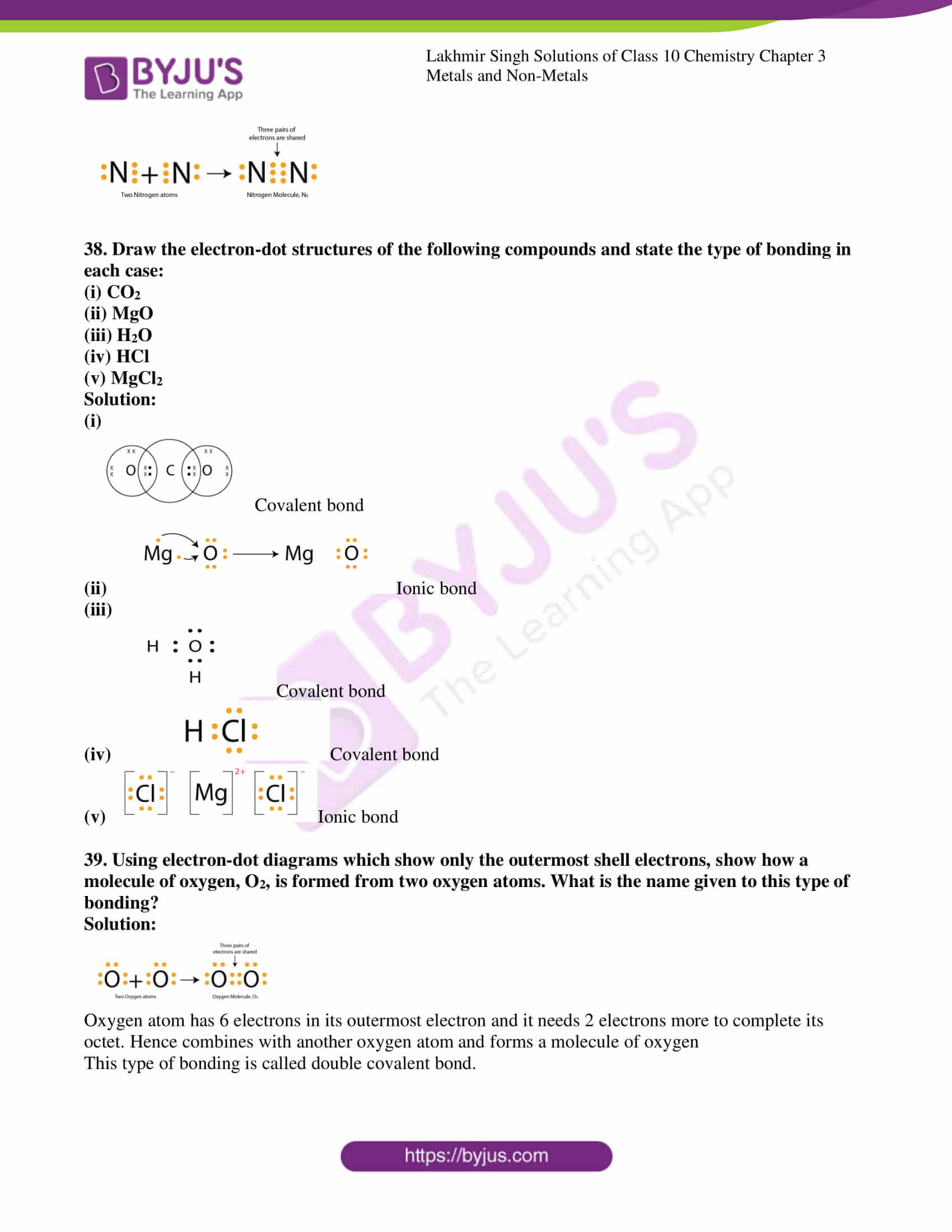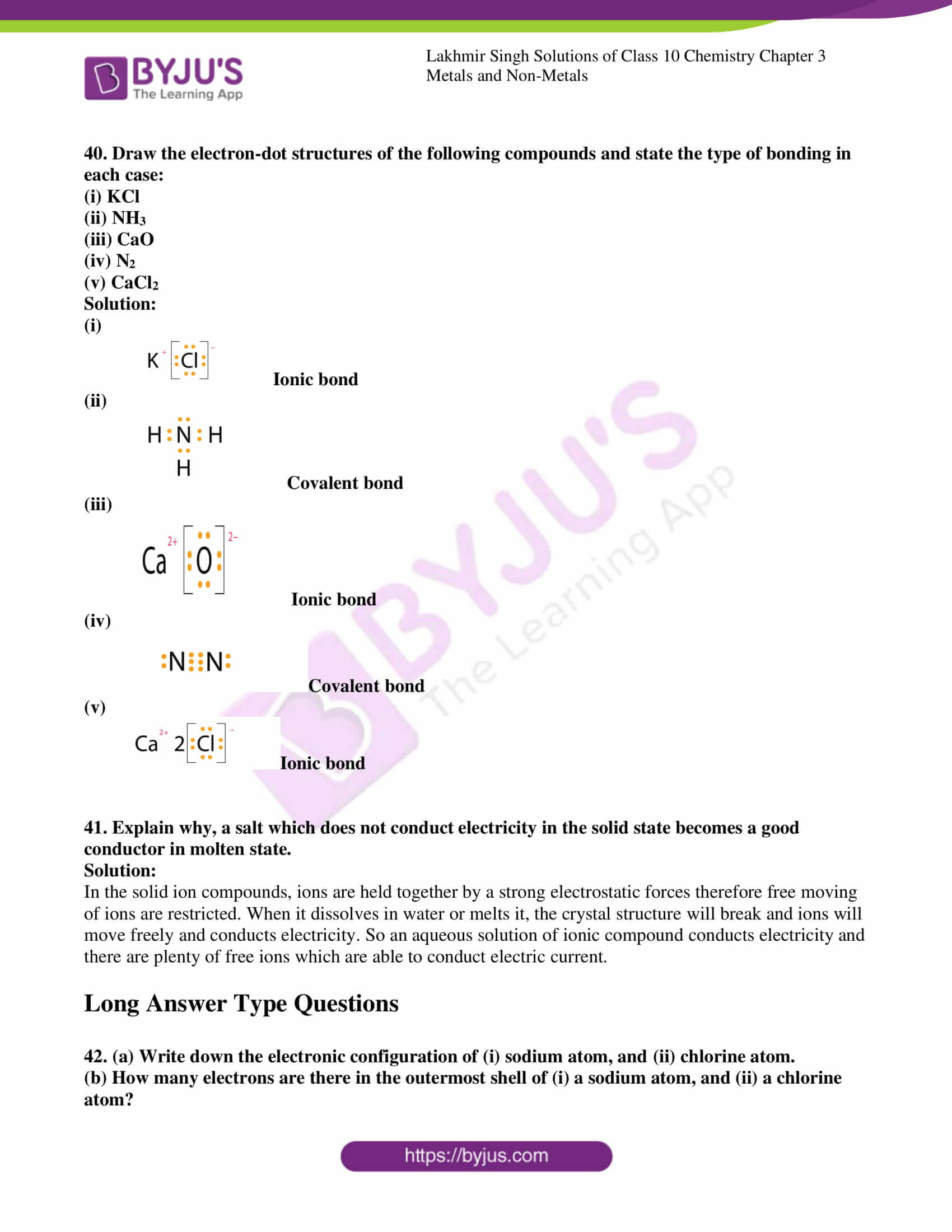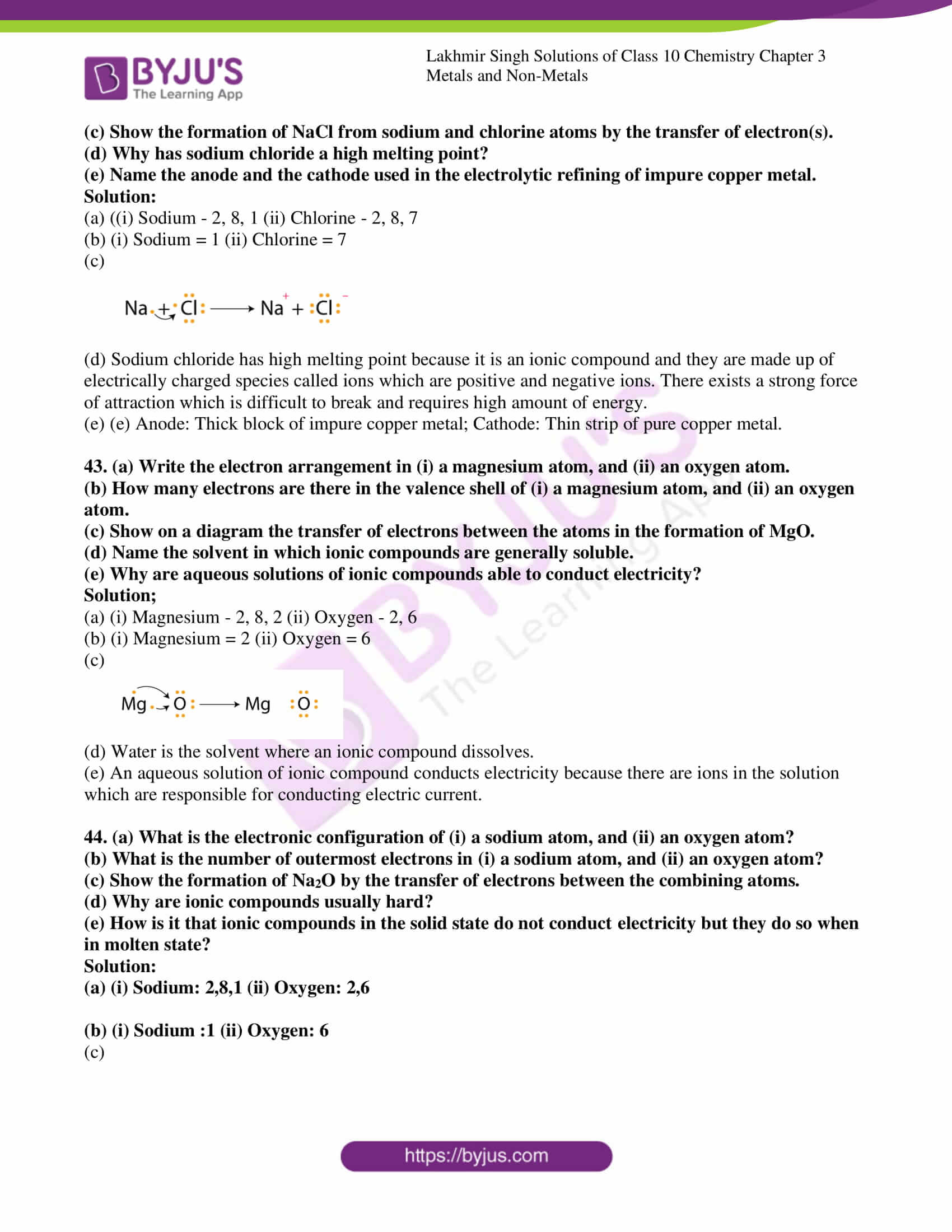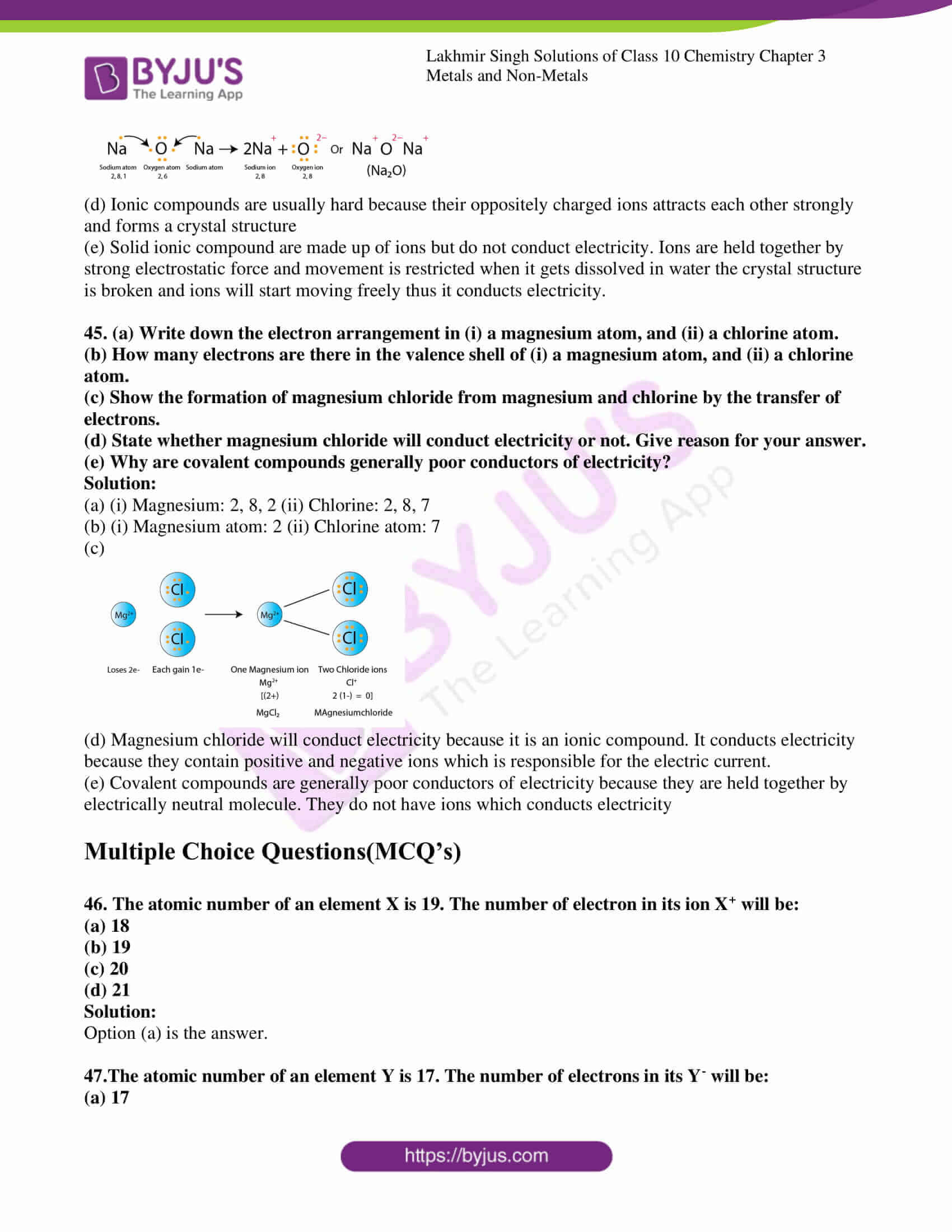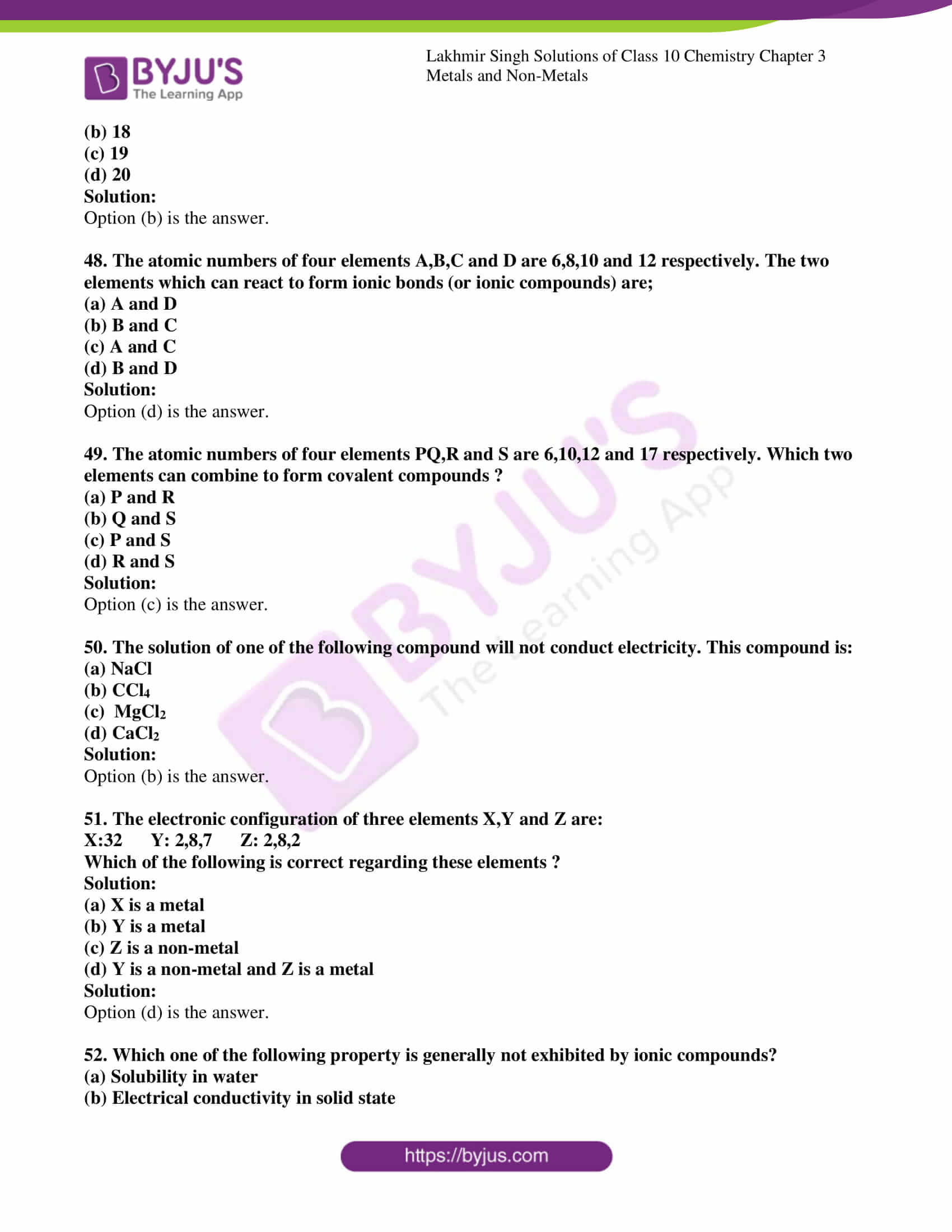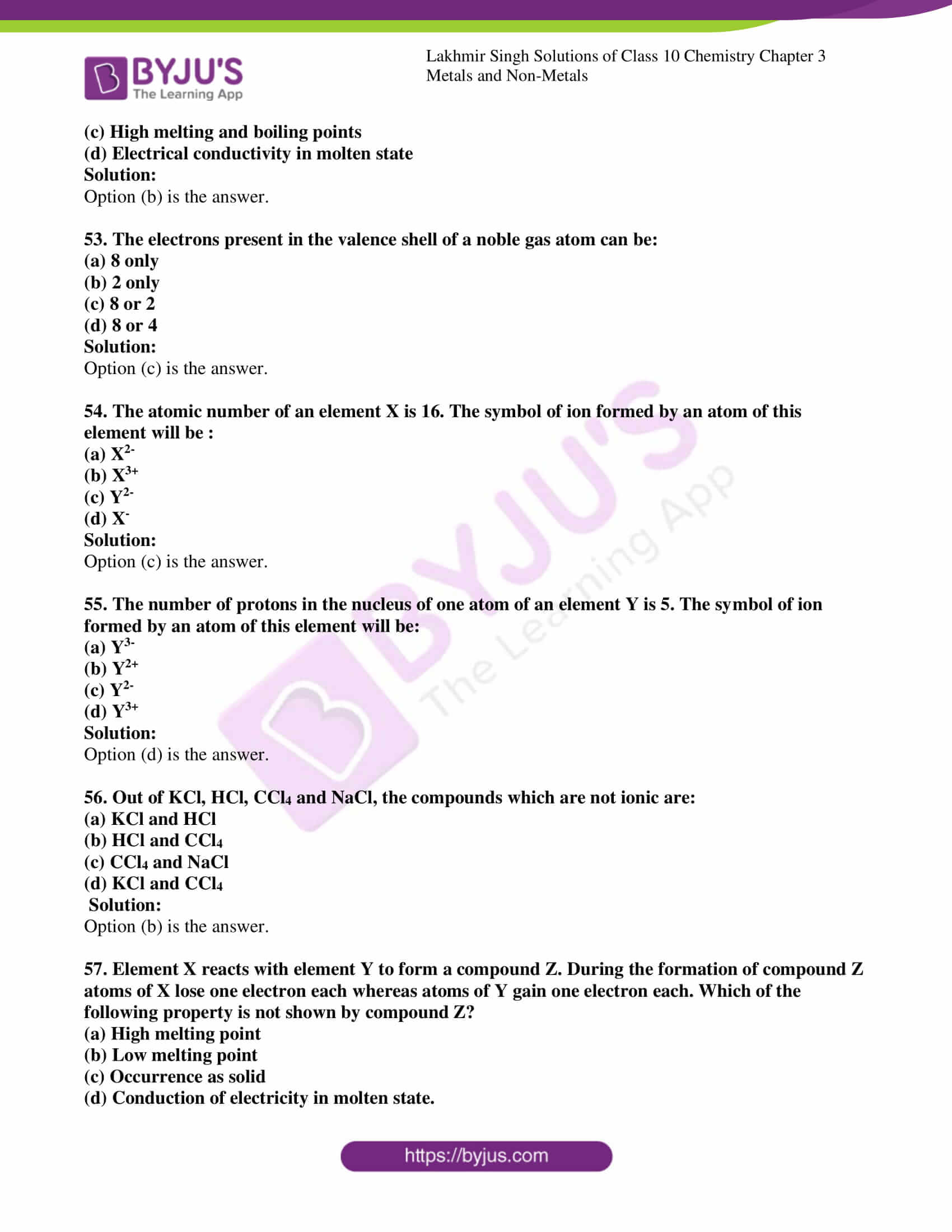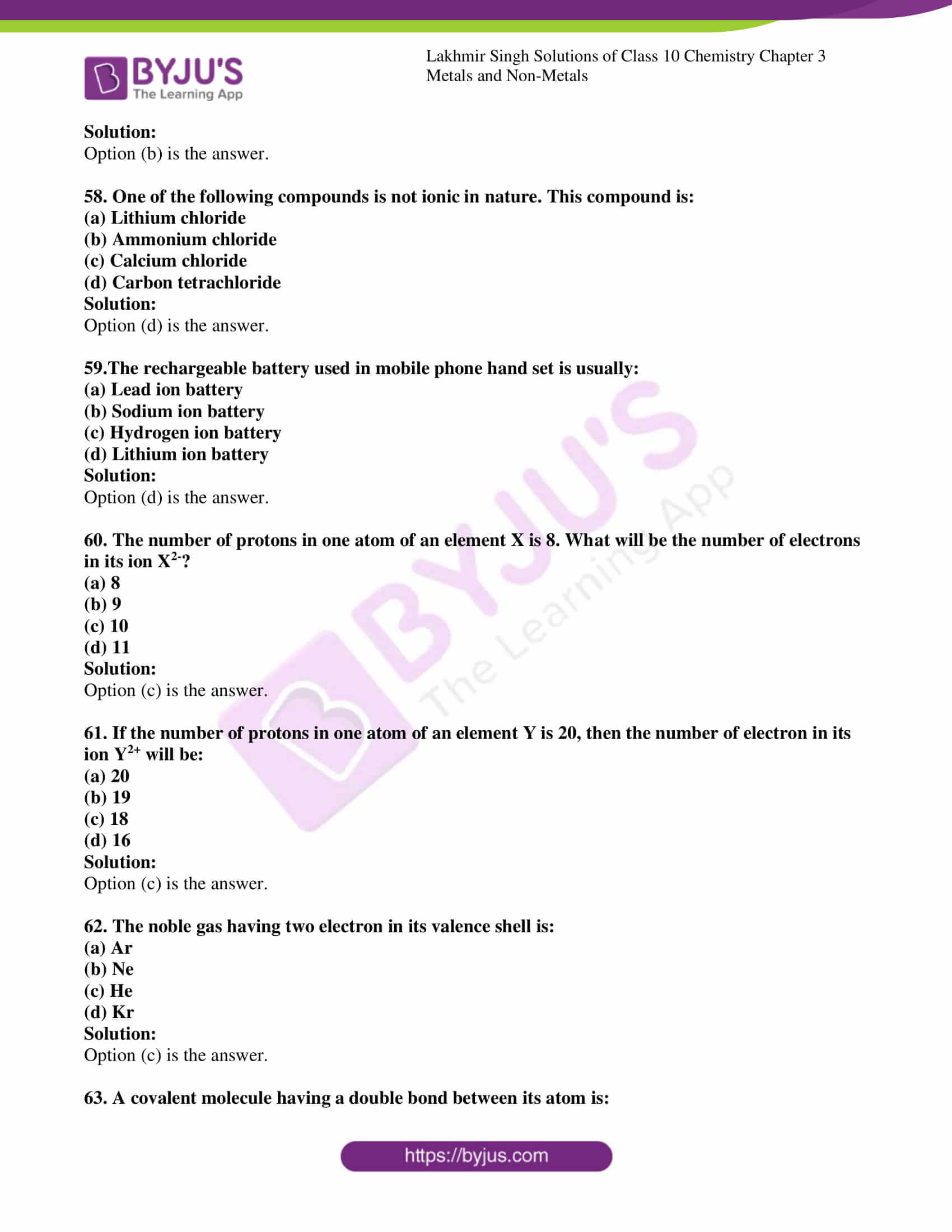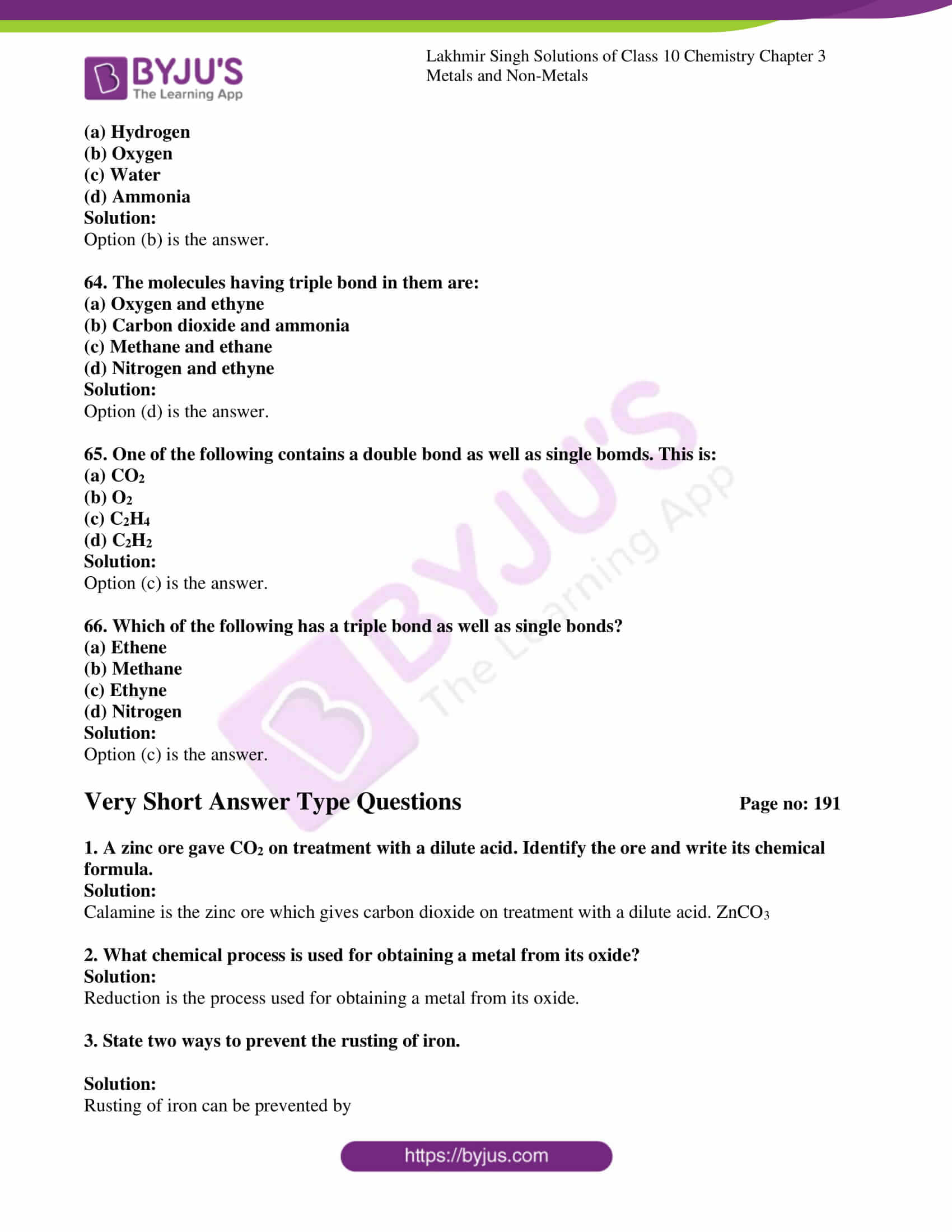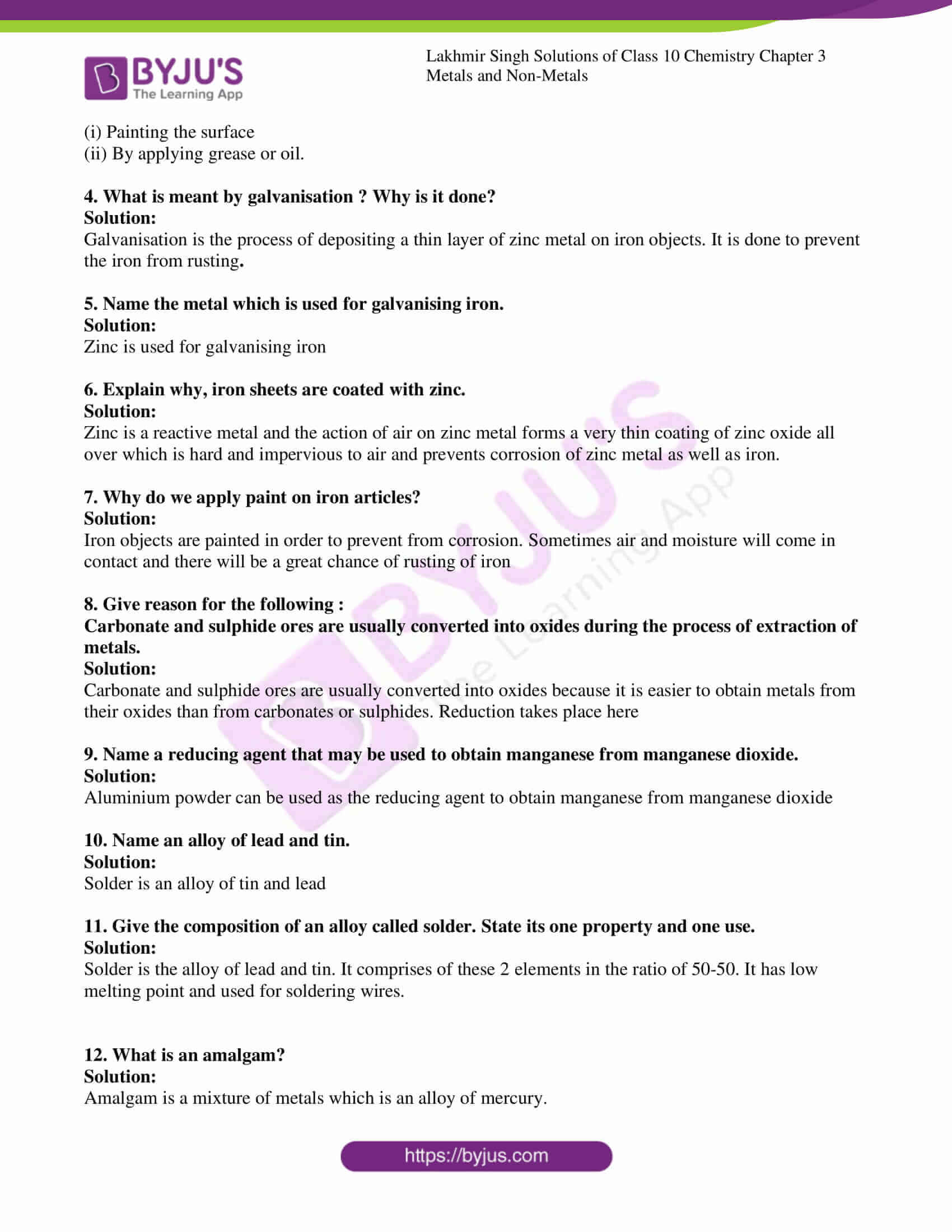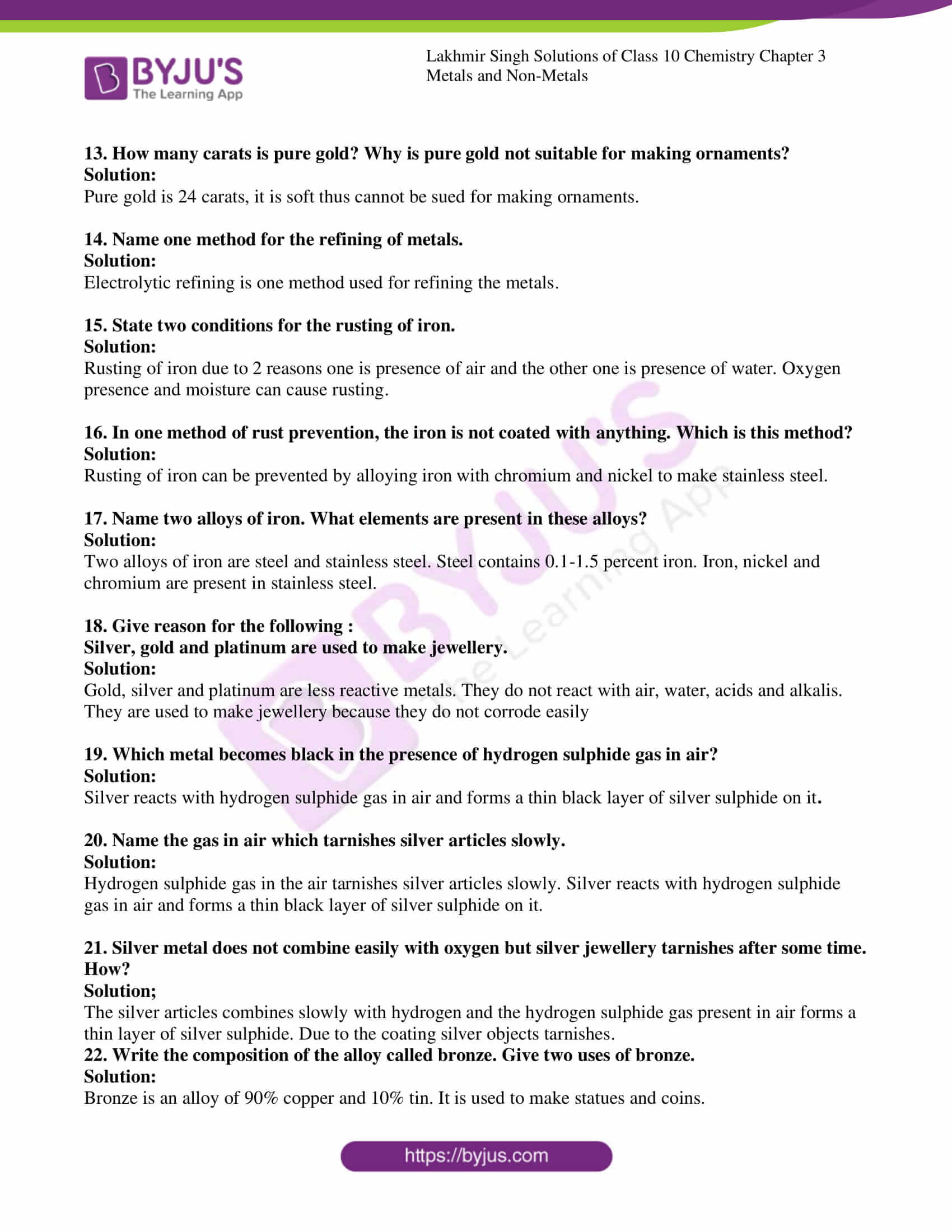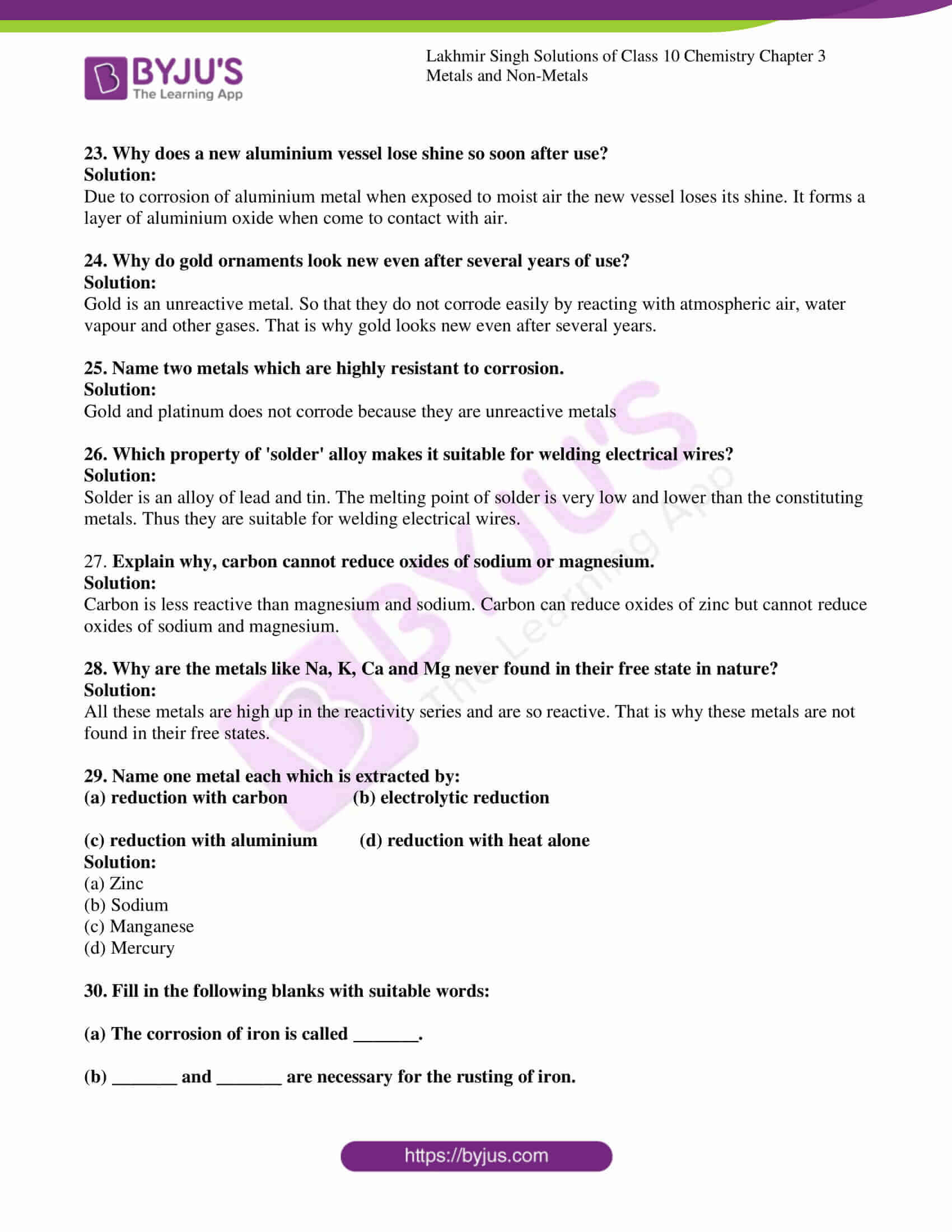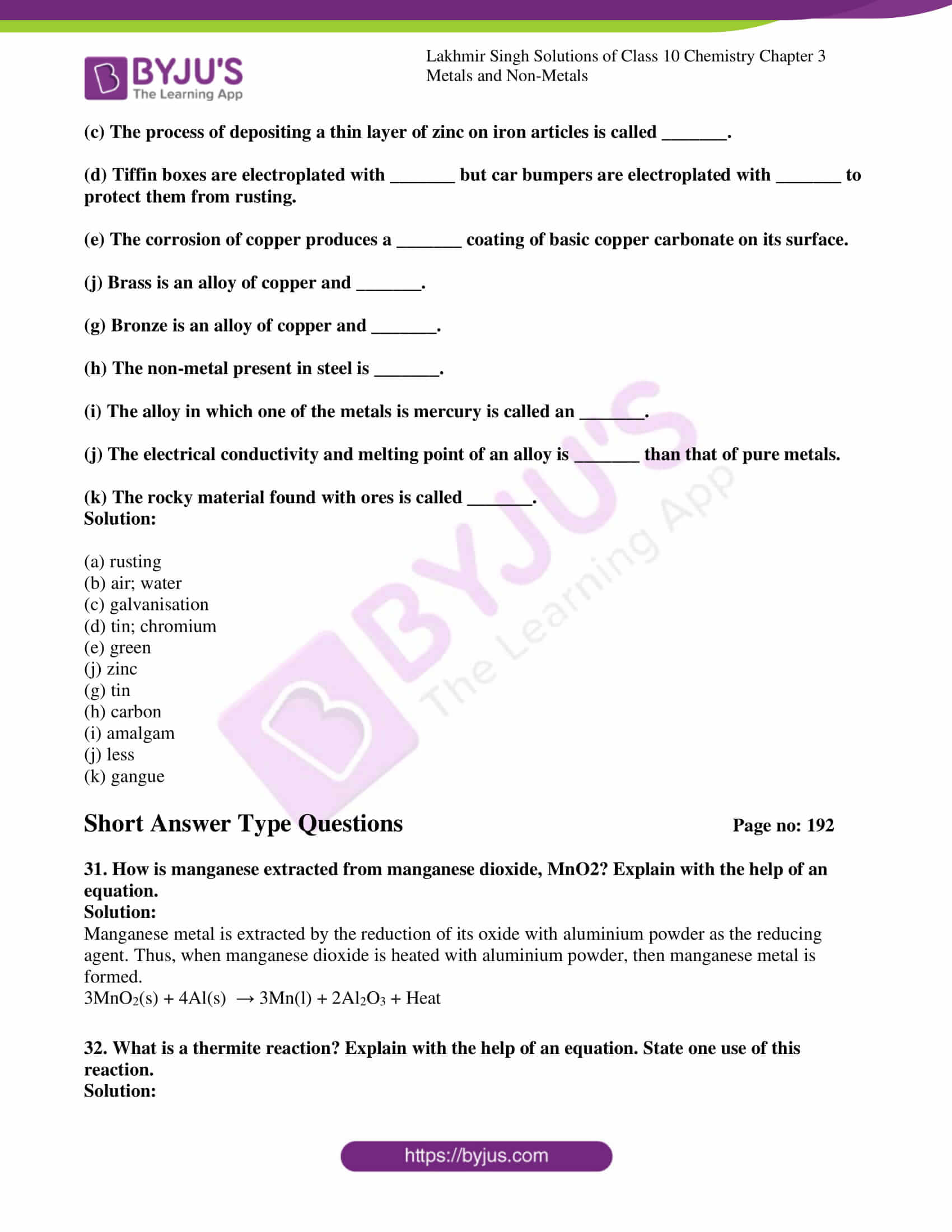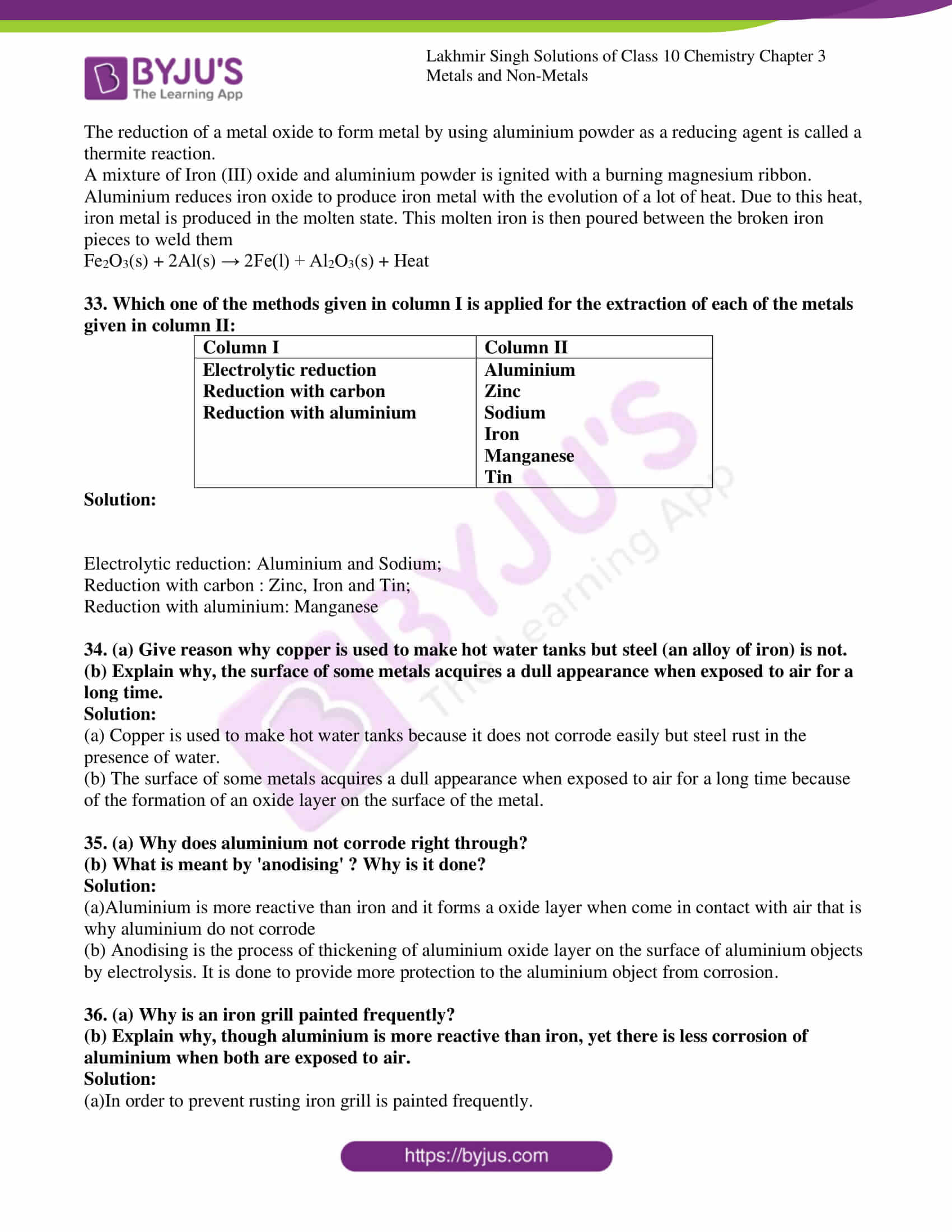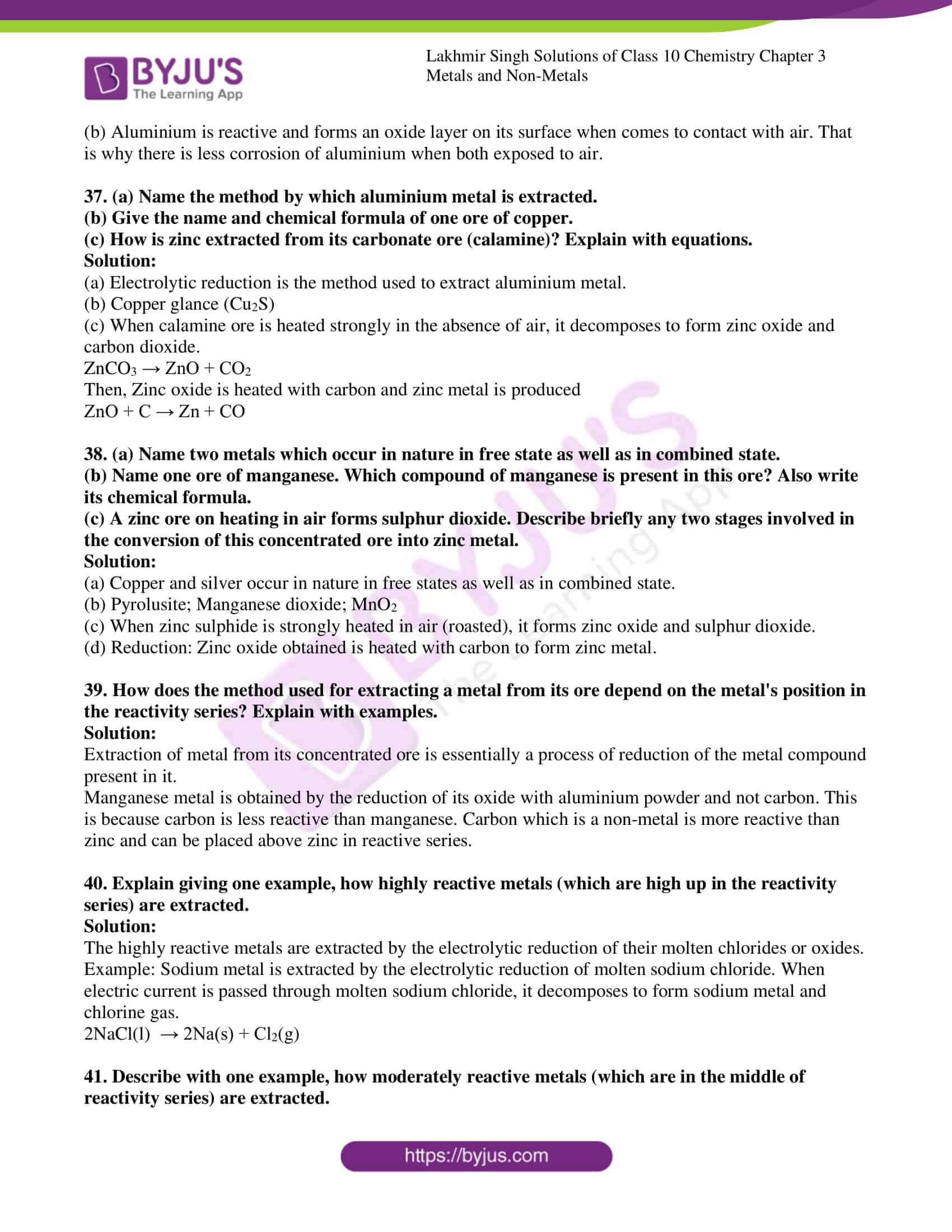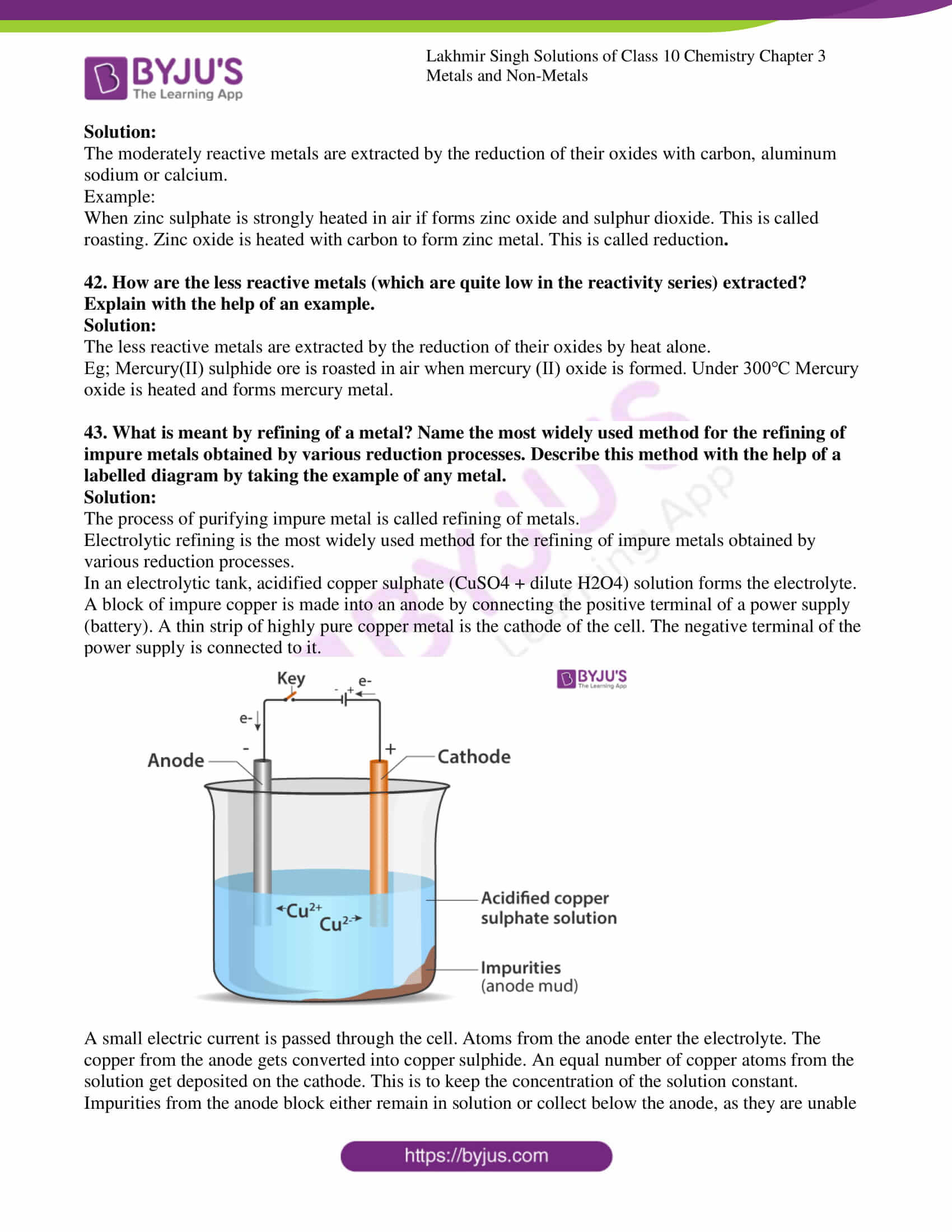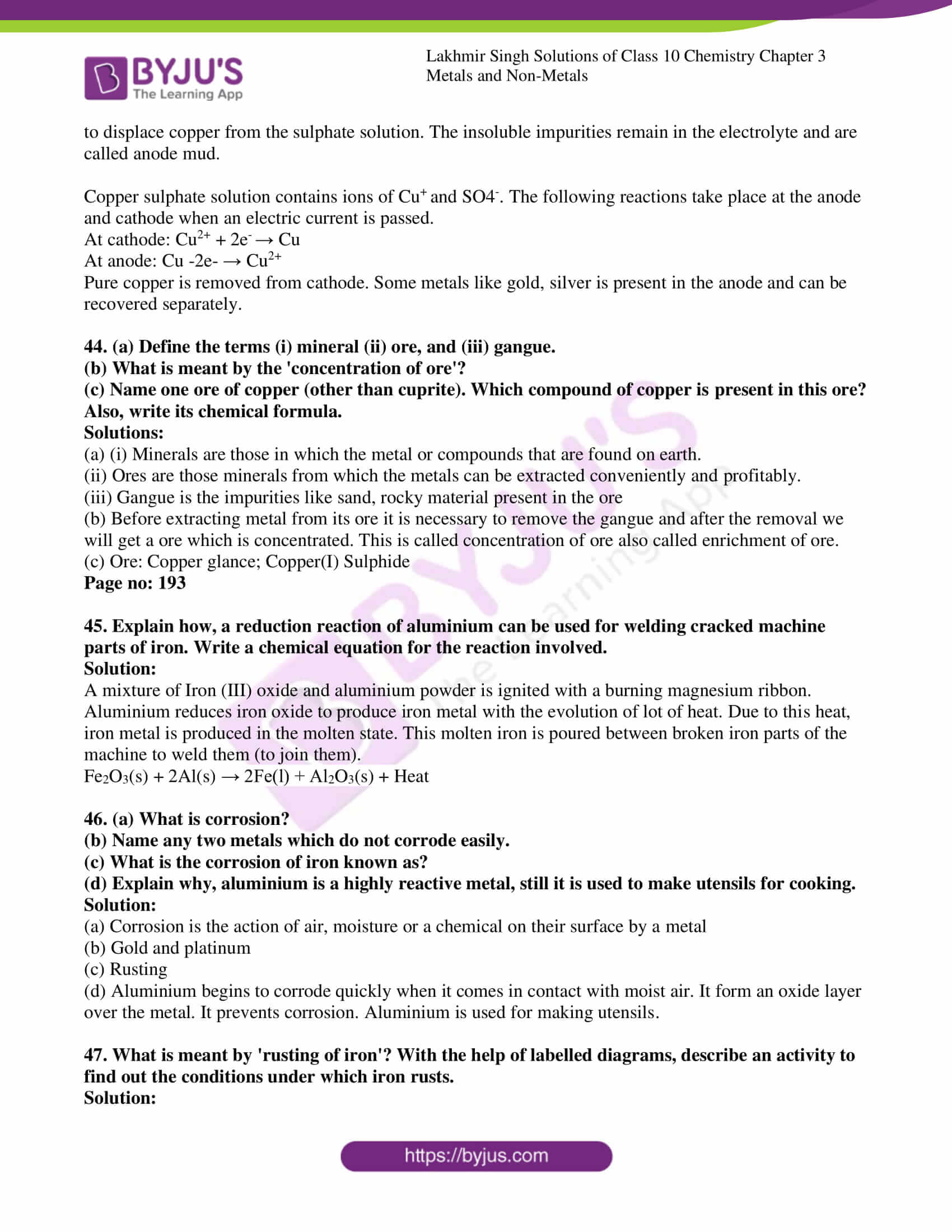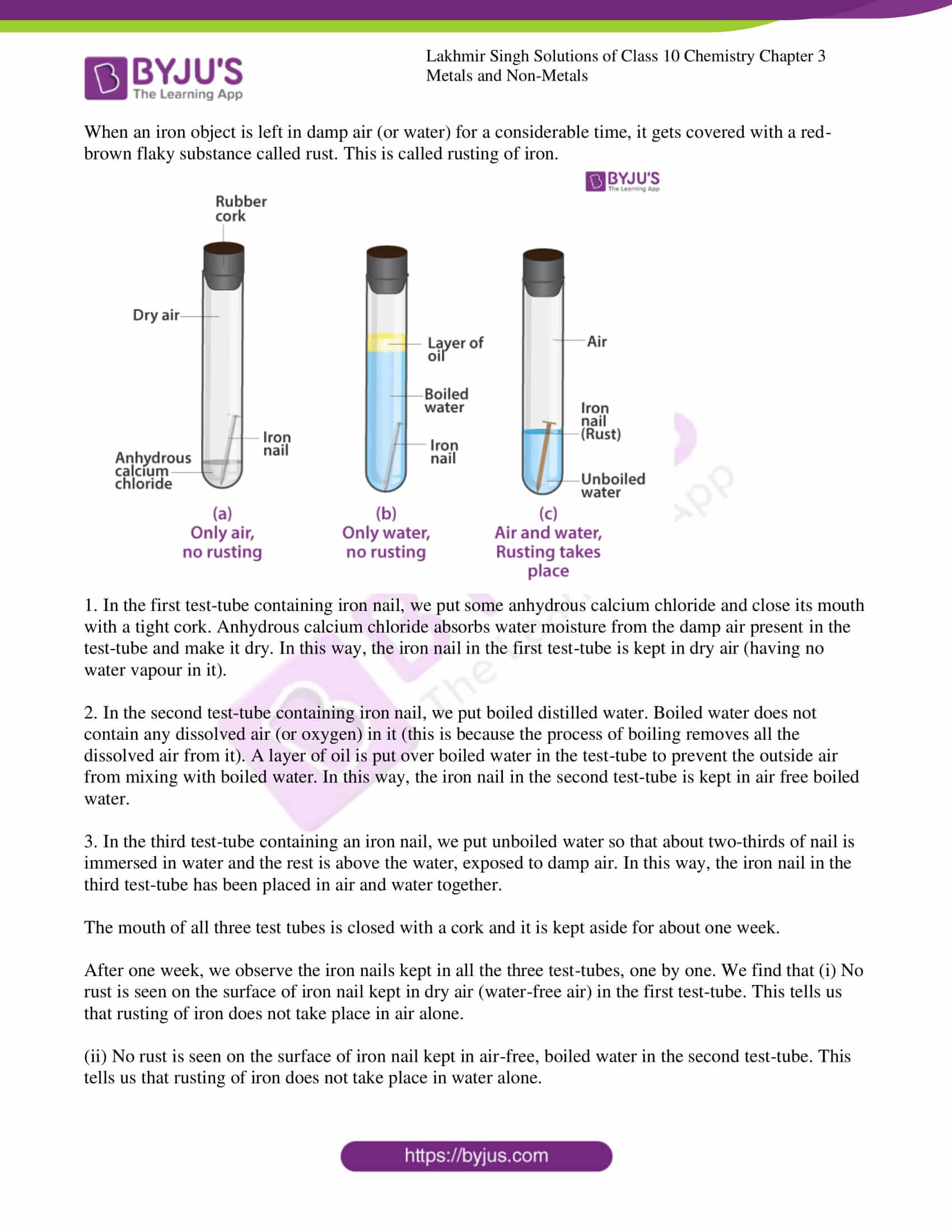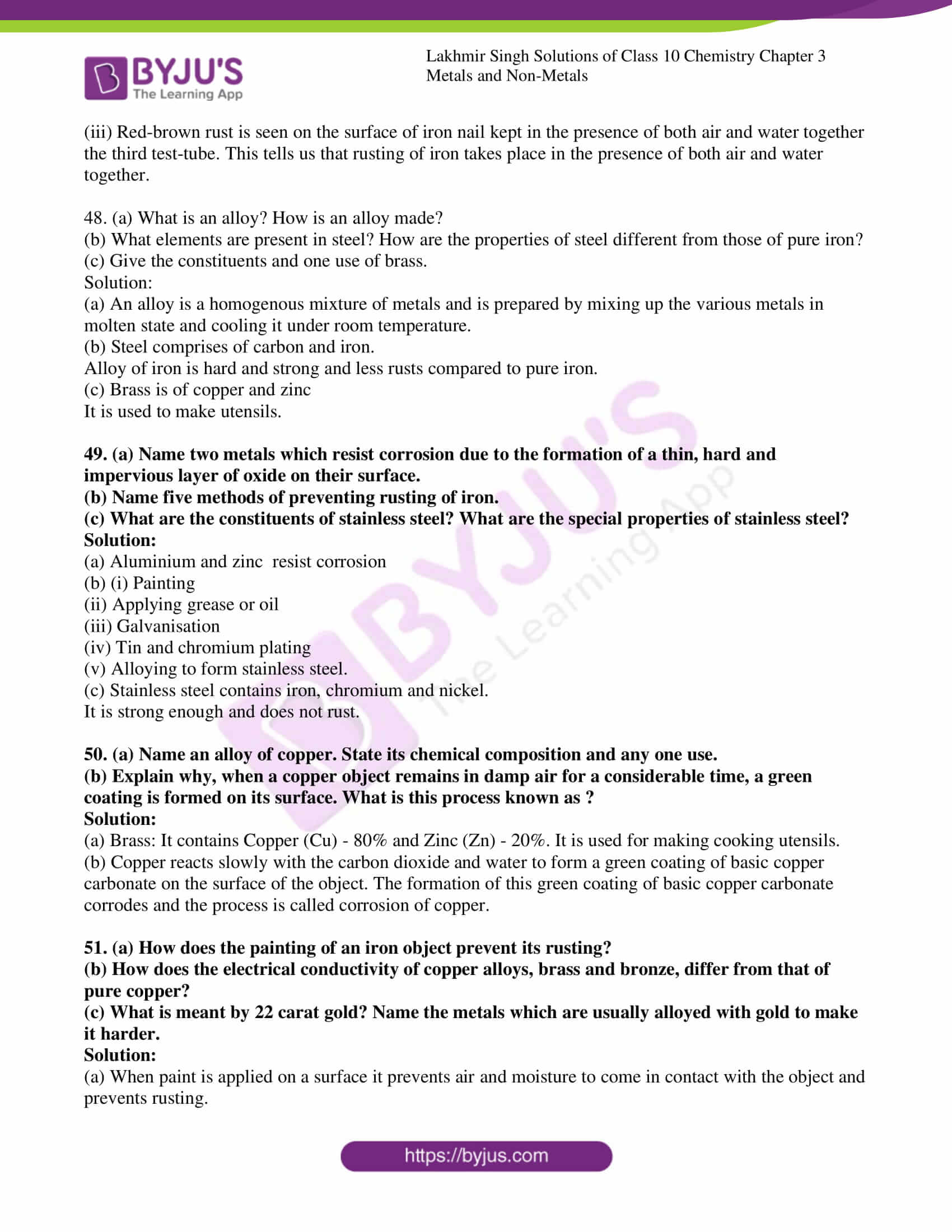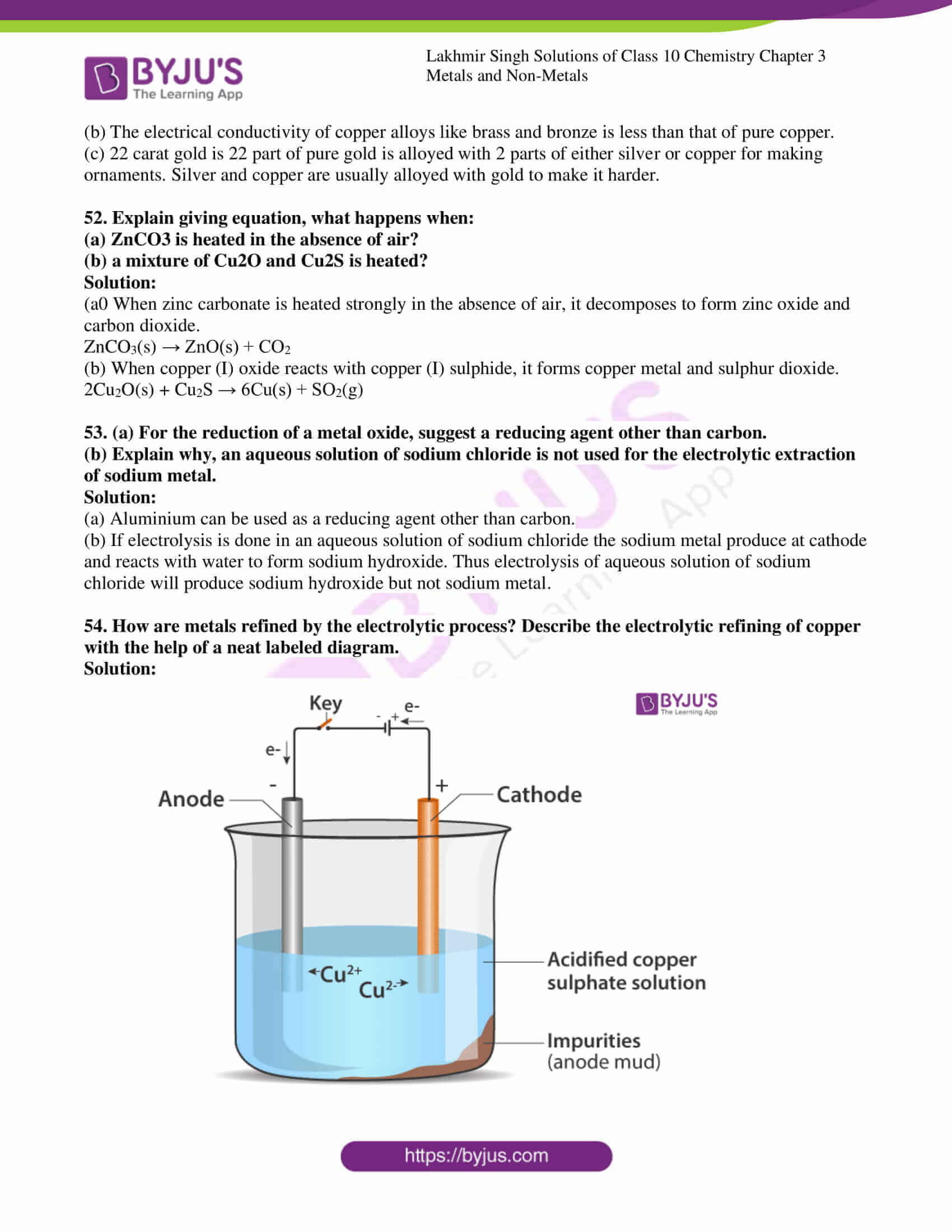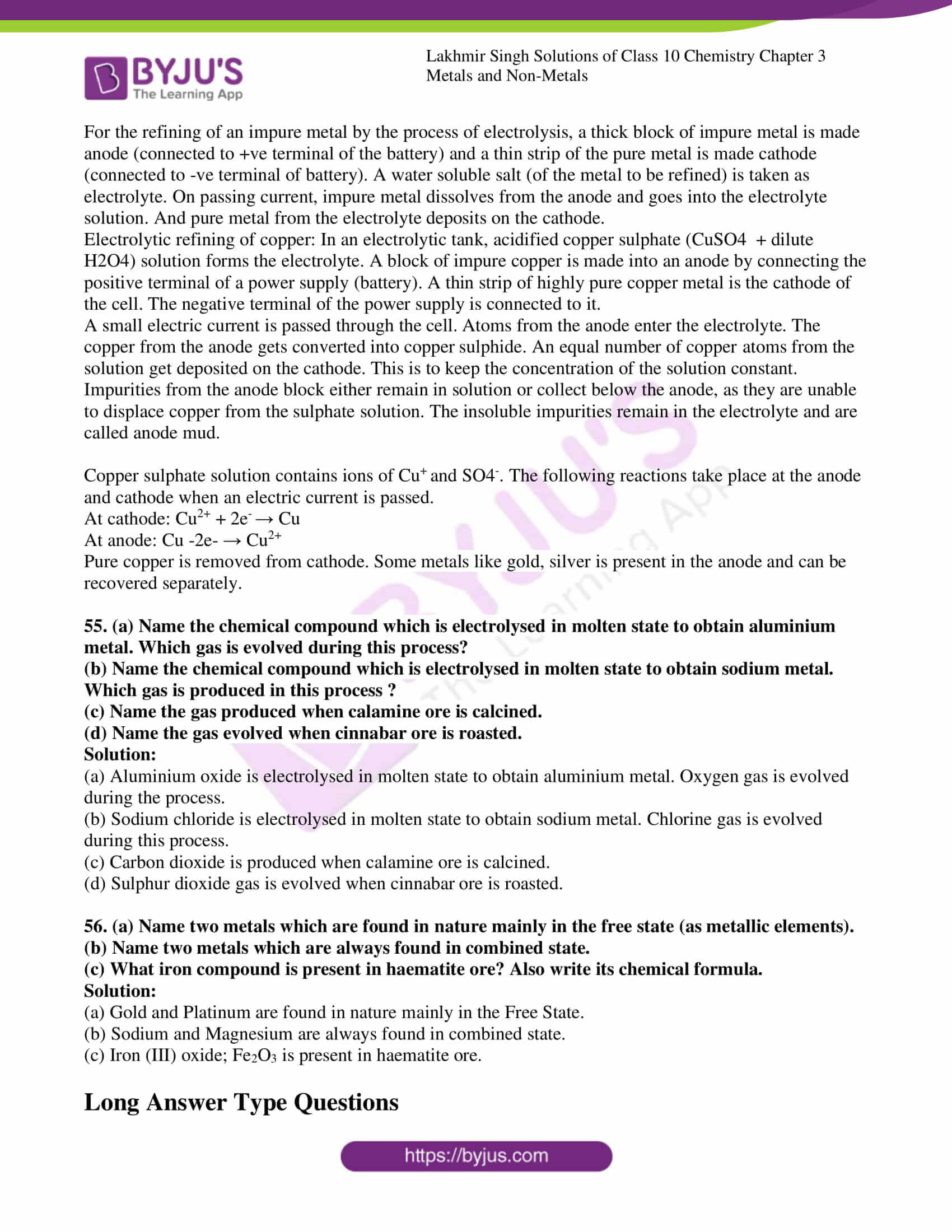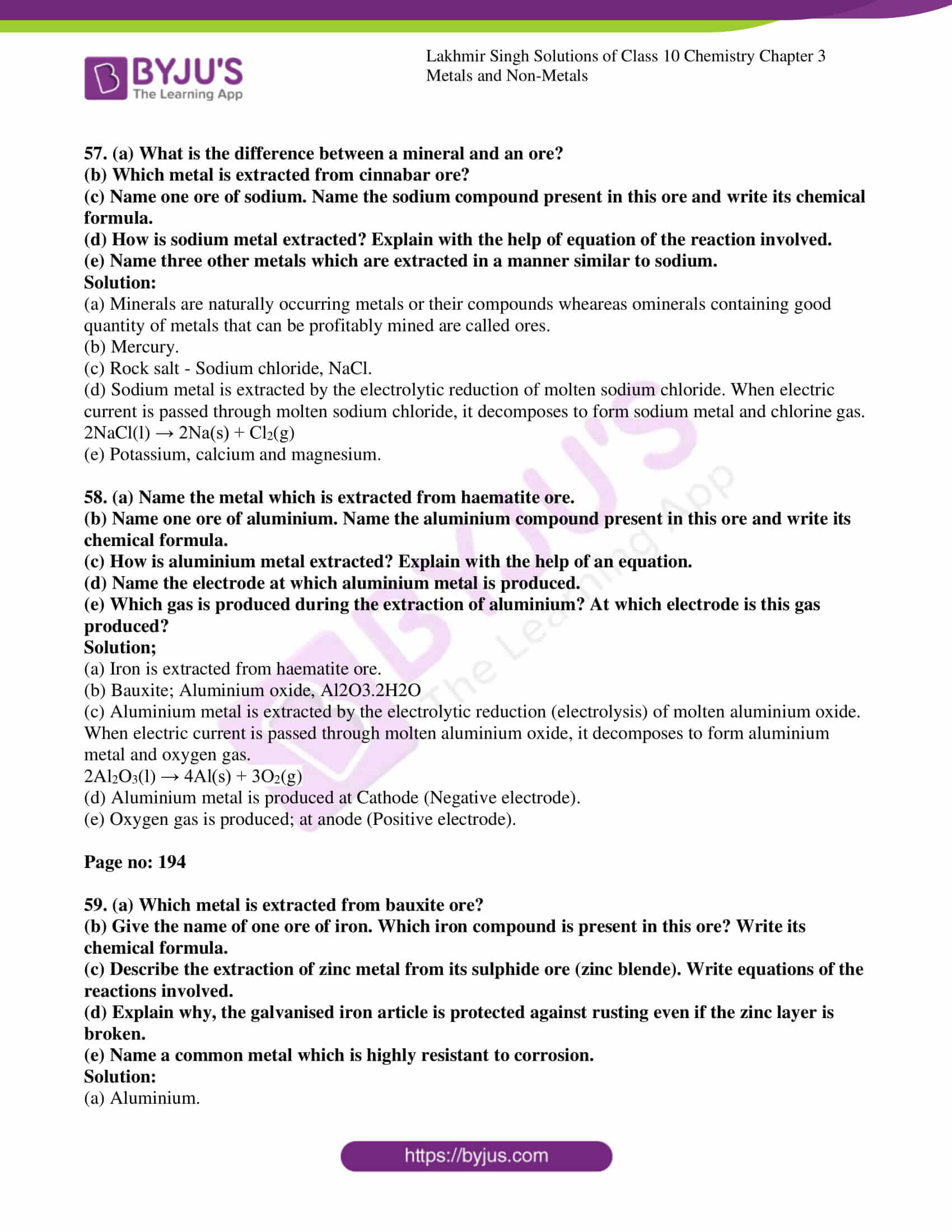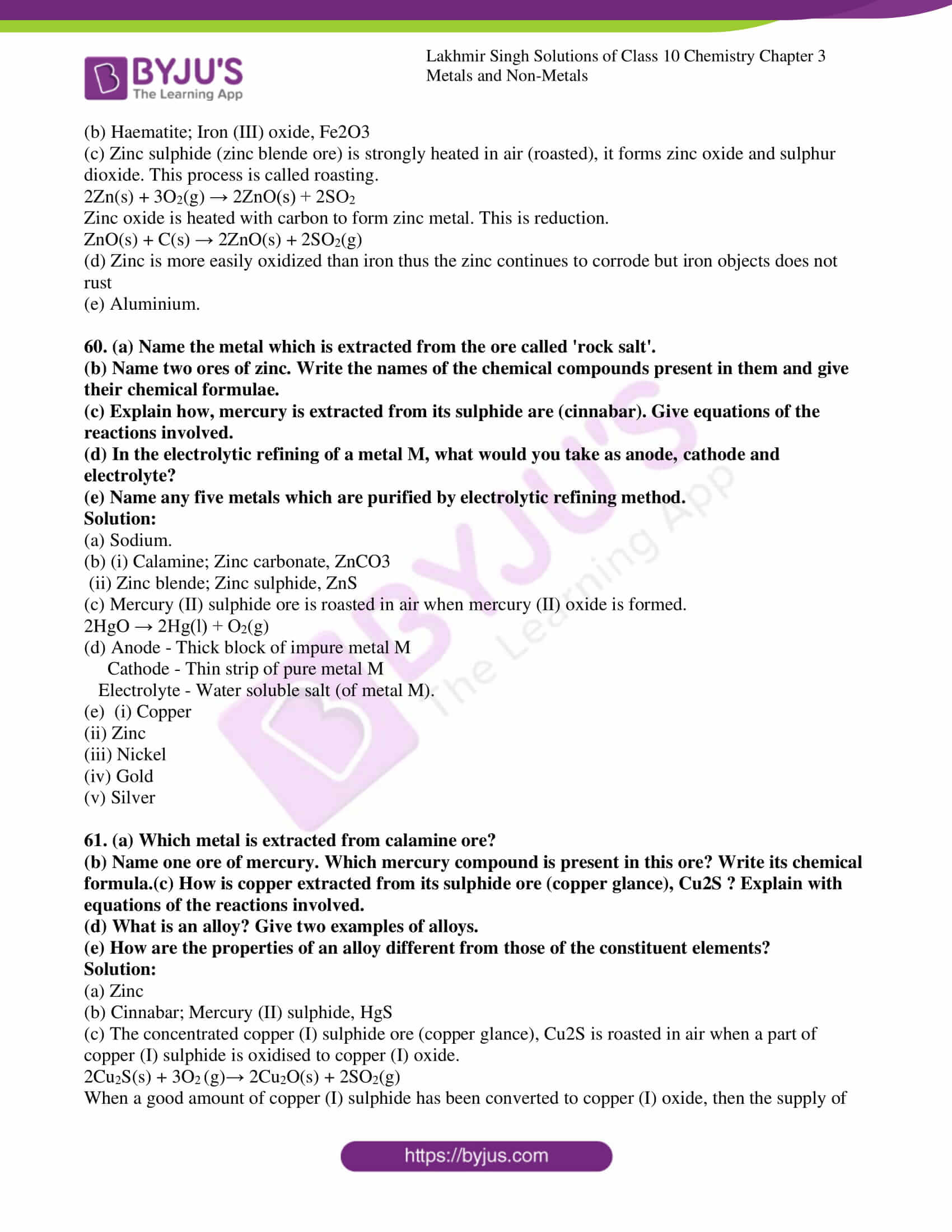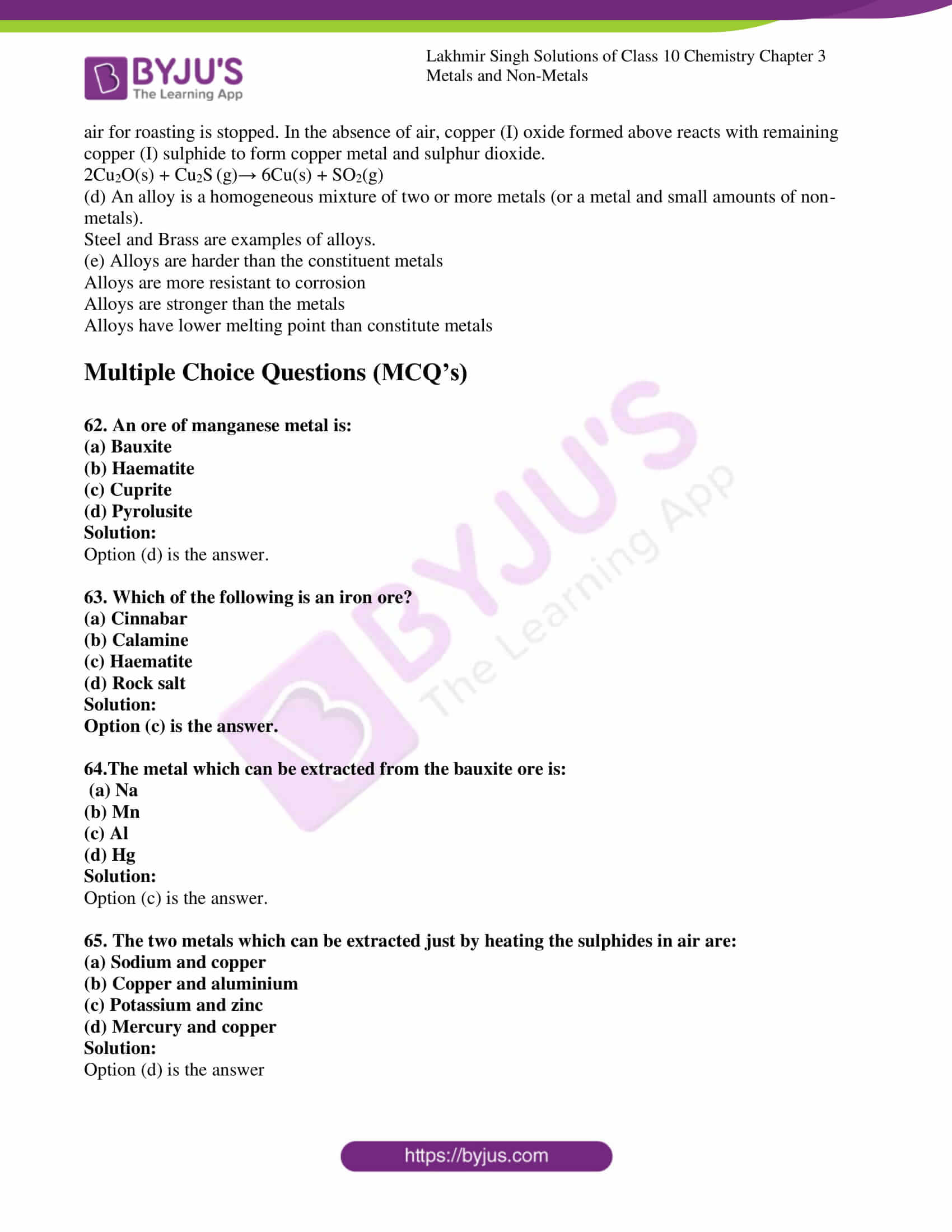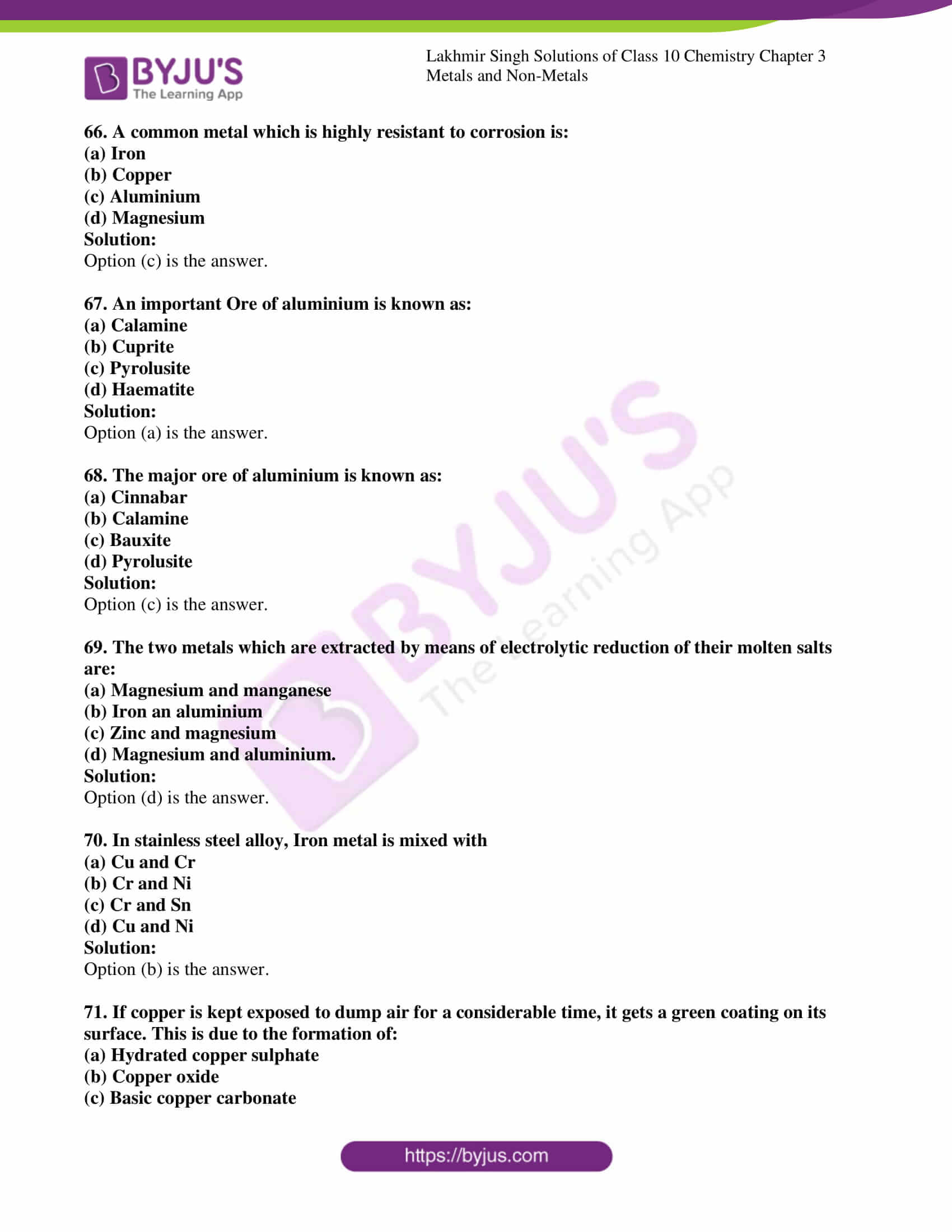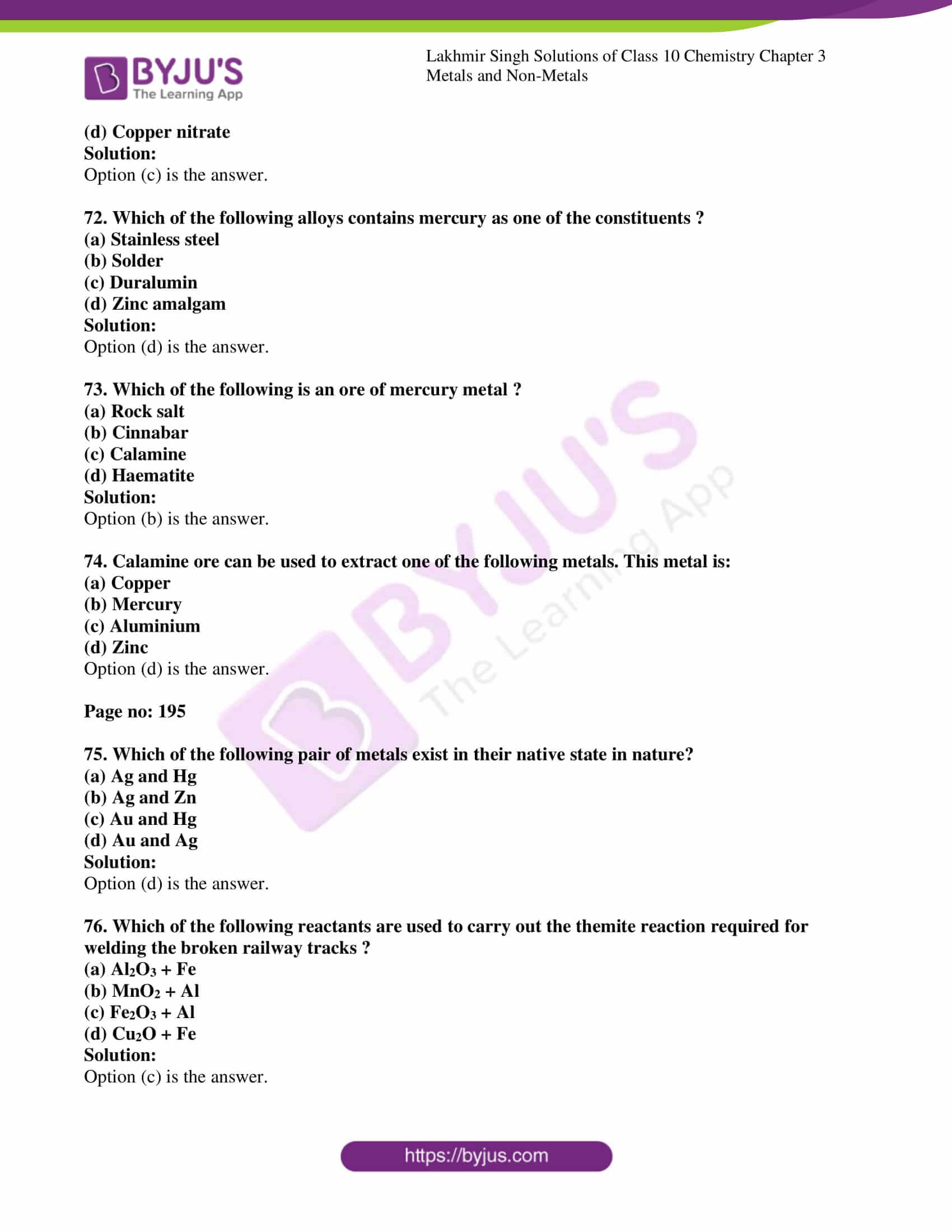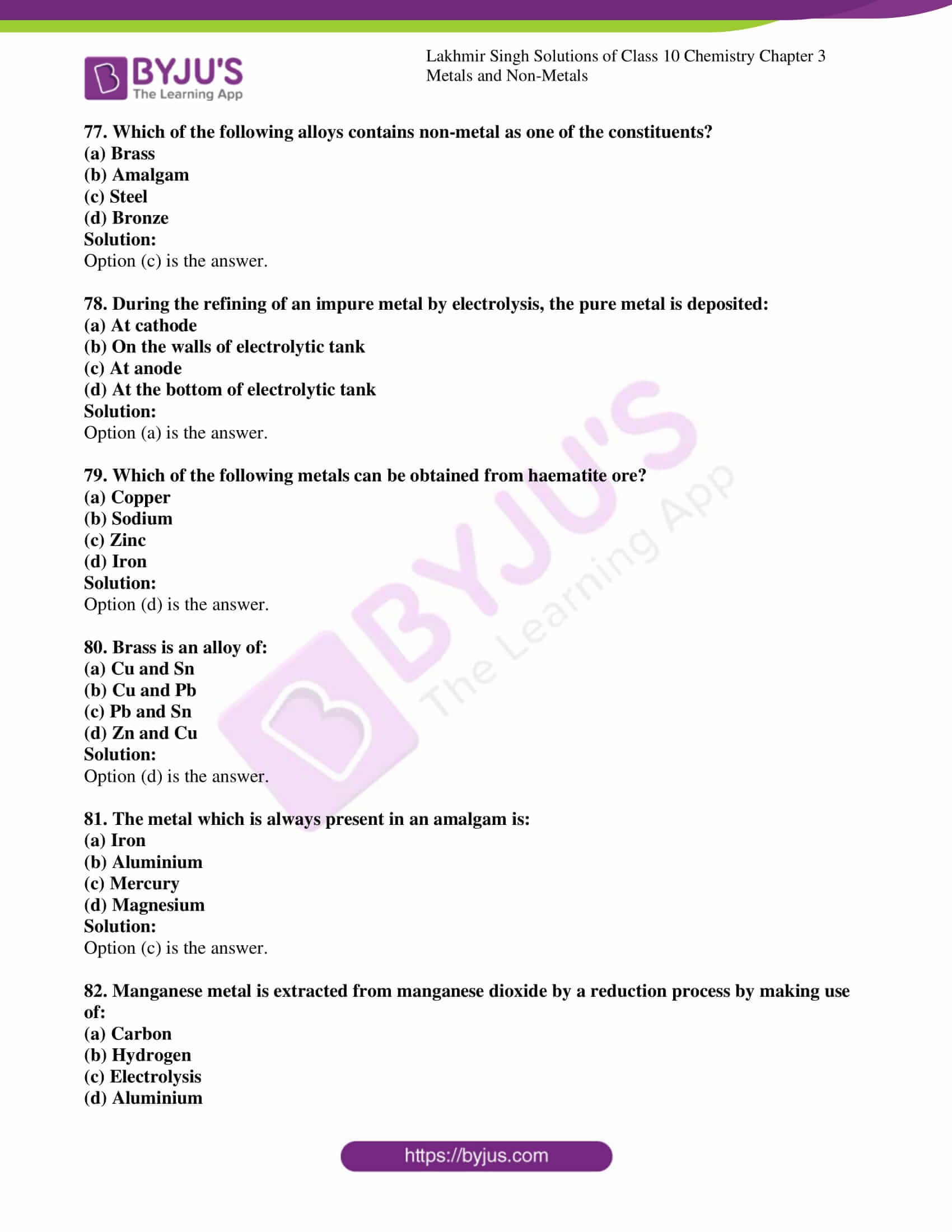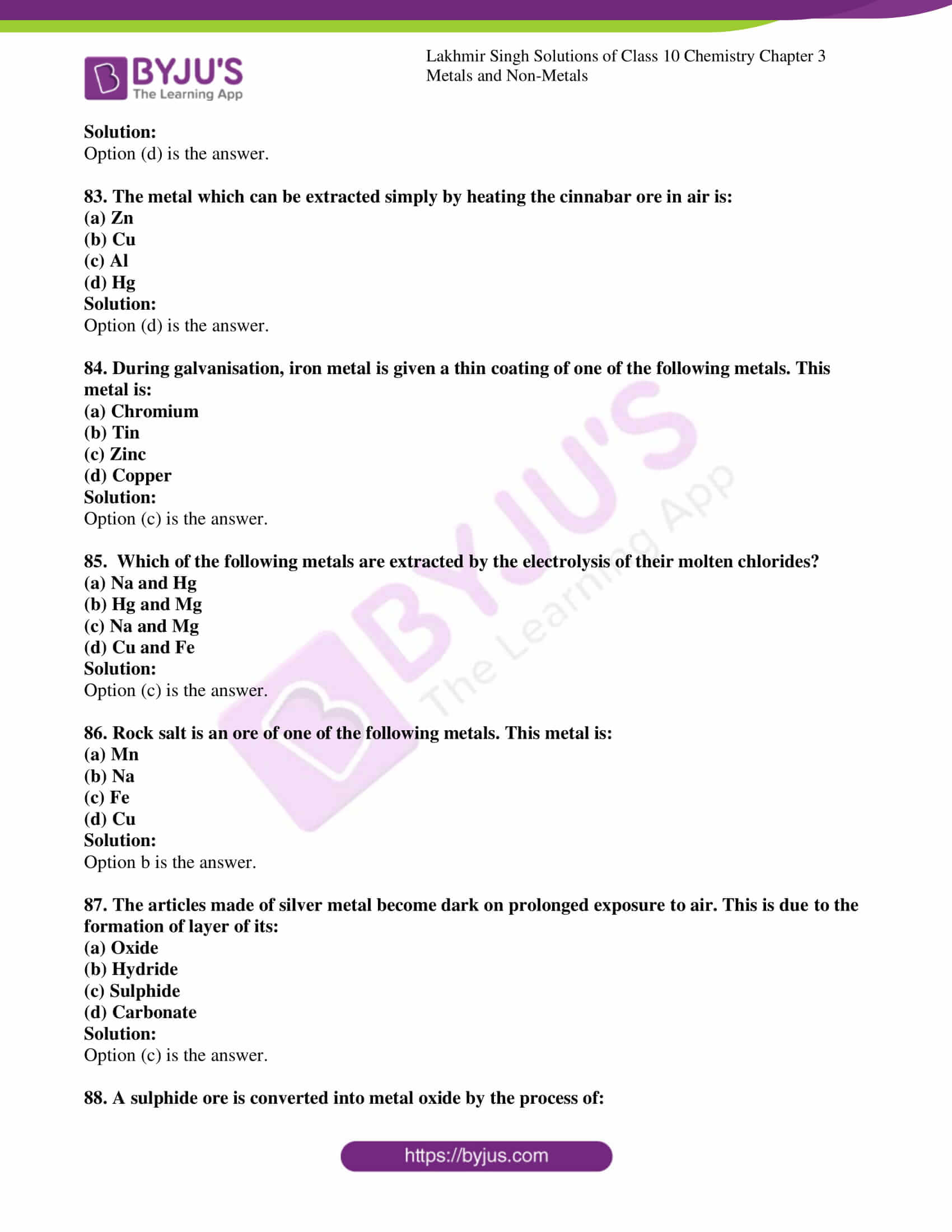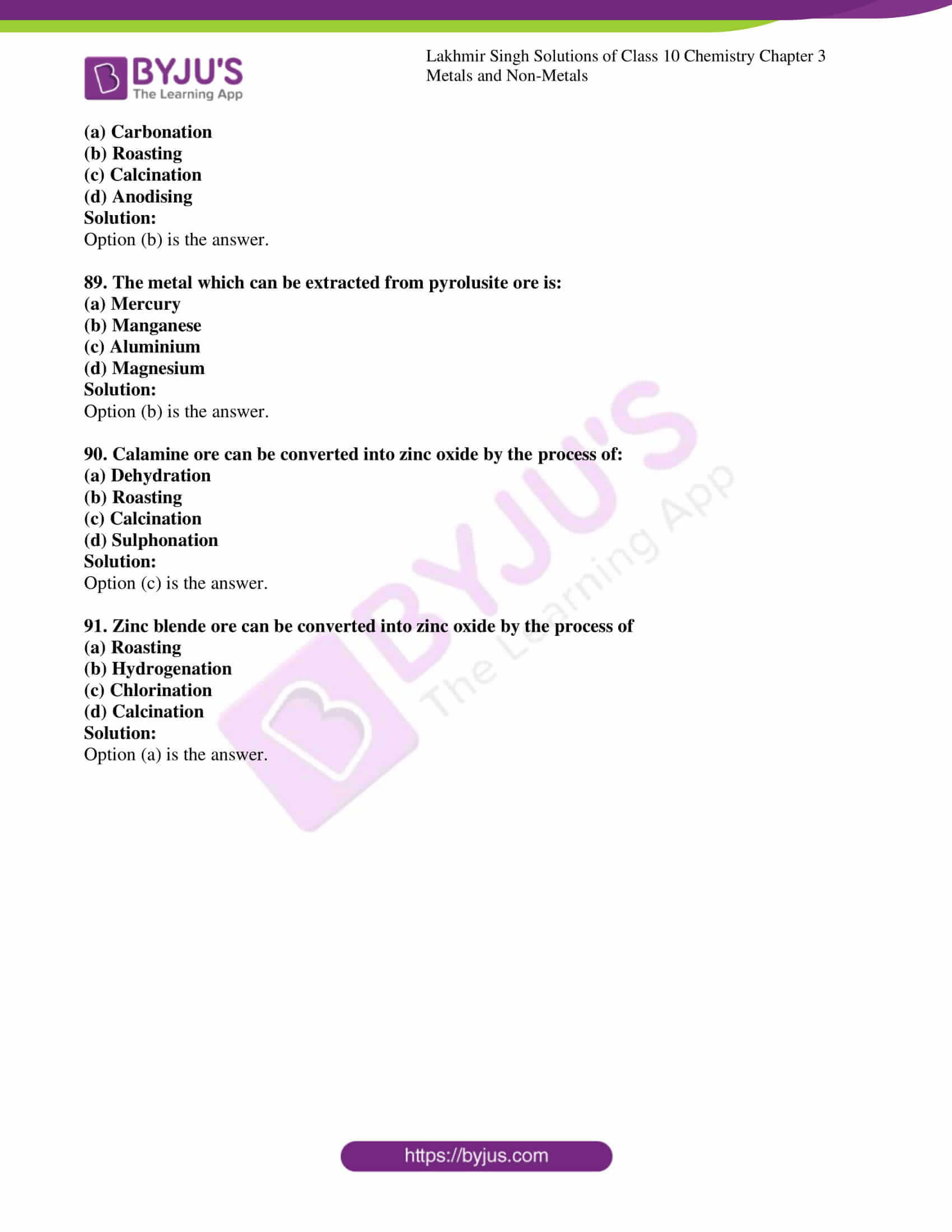### Access Lakhmir Singh Solutions for Class 10 Chemistry Chapter 3

Very Short Answer Type Questions Page no: 131

1. Name one metal and one non-metal which exist in liquid state at room temperature.

Solution:

Mercury is the metal and bromine is the non metal which exist ion liquid state at room temperature

2. Why are metals called electropositive elements whereas non-metals are called electronegative elements?

Solutions:

Metals are called electropositive elements because they can form positive ions by losing electrons from an atom and non-metals are called electronegative because they has the ability to gain electron and form negative ions.

3. (a)Name the most abundant metal in the earth’s crust.

(b)Name the most abundant non-metal in the earth’s crust.

Solution:

(a)Aluminium is the most abundant metal in the earth crust

(b)Oxygen is the most abundant non-metal in the earth crust

Page no: 132

4. Name one metal which has a low melting point.

Solution:

Cesium is the metal having low melting point

5. Name the metal which is the poorest conductor of heat.

Solution:

Lead is the metal which is a poor conductor of heat.

6. State whether the following statement is true or false:

Non-metals react with dilute acids to produce a gas which burns with a pop sound.

Solution:

False. Metals react with dilute acids to produce hydrogen gas that burns with a pop sound

7. From amongst the metals sodium, calcium, aluminium, copper and magnesium, name the metal:

(i)Which reacts with water only on boiling, and

(ii)Another which does not react even with steam.

Solution:

(i) Magnesium reacts with water only on boiling

(ii) Copper does not react even with steam

8. What changes in the colour of iron nails and copper sulphate solution do you observe after keeping the iron nails dipped in copper sulphate solution for about 30 minutes?

Solution:

Iron nail gets covered with a red brown coating of copper metal. Iron nail displaces copper from copper sulphate solution to form ferrous sulphate and the colour of copper sulphate fades away

9. What is aqua-regia? Name two special metals which are insoluble in common reagents but dissolve in aqua-regia.

Solution:

Aqua-regia is a freshly prepared mixture of one part of concentrated nitric acid and three parts of concentrated hydrochloric acid. Gold and platinum are the special metals that are insoluble in common reagents but soluble in aqua-regia

10. Give the names and formulae of (a) two acidic oxides, and (b) two basic oxides.

Solution:

(a) Carbon dioxide and sulphur dioxide are the two acidic oxides.

(b) Sodium oxide and magnesium oxides are two basic oxides.

11. What name is given to those metal oxides which show basic as well as acidic behaviour?

Solution:

Amphoteric oxides are those metal oxides which shows both the acidic and basic behaviour

12. Name two metals which form amphoteric oxides.

Solution:

Aluminium and zinc are two amphoteric oxides which shows basic and acidic behaviour.

13. A copper coin is kept immersed in a solution of silver nitrate for some time. What will happen to the coin and the colour of the solution?

Solution:

When copper coin is kept immersed in a solution of silver nitrate for some time, the colour of the coin becomes shiny greenish-white due to the deposition of silver on it.

14. Which property of copper and aluminium makes them suitable:

(a)For making cooking utensils and boilers?

(b)For making electric wires?

Solution:

(a)High thermal conducting property of copper and aluminium makes suitable for making cooking utensils and boilers.

(b) High electrical conductivity property of copper and aluminium makes suitable for making electric wires.

15. Write the names and formulae of (a) a metal hydride, and (b) a non-metal hydride.

Solutions:

Sodium hydride is a metal hydride (NaH)

Hydrogen sulphide is a non-metal hydride (H2S)

16. Name the metal which has been placed:

(a)At the bottom of the reactivity series

(b)At the top of the reactivity series

(c)Just below copper in the reactivity series

Solution:

(a) Gold is the metal placed at the bottom of the reactivity series

(b) Potassium is the metal at the top of the reactivity series

(c) Mercury is the metal placed just below the copper in the reactivity series

17. Which of the two metals is more reactive: copper or silver?

Solution:

Copper is more reactive than silver. The more reactive element can displace the less reactive element from the solution.

18. (a)Name one metal which is stored in kerosene oil.

(b)Name one non-metal which is stored under water.

Solution:

(a) Sodium is the metal stored in kerosene oil in order to prevent it from coming in contact with oxygen and moisture

(b) White phosphorus is the non-metal which is stored under water. Because it catches fire when exposed to air

19. Write equation for the reaction of:

(a)Sodium with oxygen

(b)Magnesium with oxygen

Solution:

(a)Sodium reacts with oxygen to form sodium oxide

4Na(s) + O2(g) → 2Na2O(s)

(b) Magnesiuum reacts with oxygen to form magnesium oxide

2Mg(s) + O2(g) → 2MgO(s)

20. Name two metals which are used:

(a)For making electric wires.

(b)For making domestic utensils and factory equipment.

(c)For making jewellery and to decorate sweets.

Solution:

(a) Aluminium and copper are the metals used for making electric wires

(b) Copper and aluminium are the metals used for making domestic utensils and factory equipment

(c) Gold and silver are the metals used for making jewellery and to decorate sweets

21. Which metal foil is used for packing some of the medicine tablets?

Solution:

Aluminium foil is used for packing some of the medicine tablet and also food items

22. Name the non-metal which is used:

(a) To convert vegetable oil into vegetable ghee (solid fat).

(b) As a rocket fuel (in liquid form).

(c) To make electrodes of dry cells.

(d) To preserve food materials.

(e) In the vulcanization of rubber.

Solution:

(a) Hydrogen is the non-metal which is used to convert vegetable oil into vegetable ghee

(b) Hydrogen is used as rocket fuel in its liquid form

(c) Carbon is used to make electrodes for dry cells

(d) Nitrogen is the non-metal used to preserve food material

(e) Vulcanization of rubber helps to improve the strength of the rubber and sulphur is used for the process.

23. Name one property which is characteristic of (a) metals, and (b) non-metals.

Solution:

Metals are malleable whereas non-metals are non-malleable.

24. What is meant by “brittleness”? Which type of elements usually shows brittleness: metals or non-materials?

Solution:

Brittleness is the property in which hard but liable to break easily. Mostly all non-metals show brittleness.

25. What will happen if a strip of zinc is immersed in a solution of copper sulphate?

Solution:

When a zinc metal is immersed in a copper sulphate solution, copper sulphate will lose its blue colour and a red brown coating of copper will deposit on zinc.

26. What will happen if a strip of copper is kept immersed in a solution of silver nitrate (AgNO3)?

Solution:

When strip of copper is kept immersed in a solution of silver nitrate the solution will gradually become blue and a shining greyish white deposit of silver metal will be formed on copper strip.

27. What happens when iron nails are put into copper sulphate solution?

Solution:

When iron nails are put into the copper sulphate solution, the blue colour of the copper sulphate solution will fade away and a reddish brown copper metal will form.

28. How would you show that silver is chemically less reactive than copper?

Solution:

When silver is dipped in a copper sulphate solution, no reaction will happen. Silver cannot displaces copper from the copper sulphate and thus silver shows less reactivity than copper.

29. Give reasons for the following:

Blue colour of copper sulphate solution is destroyed when iron fillings are added to it.

Solution:

Blue colour of copper sulphate is destroyed because iron displaces copper from copper sulphate solution as iron is more reactive than copper.

Page no: 133

30. Name a non-metal having a very high melting point.

Solution:

Diamond is the non-metal having low melting point

31. Which property of graphite is utilized in making electrodes?

Solution:

Graphite is having a properly of conduction of electricity. This conductivity helps in making electrodes

32. Name two non-metals which are both brittle and non-ductile.

Solution:

Sulphur and phosphorus are the two non-metals which are both brittle and non-ductile, which can be easily breakable and flexible

33. Explain why, the surface of some metals acquires a dull appearance when exposed to air for a long time.

Solution;

The surface of some metal acquires a dull appearance when exposed to air for long time because metals form a thin layer of oxides, carbonates or sulphides on their surface

34. Complete and balance the following equations:

(a) Na + O2

(b) Na2O + H2O →

(c) Fe(s) + H2O →

(d) Cu(NO3)(aq) + Zn →

Solution:

(a) 4Na + O2 → 2Na2O

(b) Na2O + H2O → 2NaOH

(c) Fe(s) + H2O → Fe3O4 + 4H2

(d) Cu(NO3)(aq) + Zn → Zn(NO3)2 + 2Cu

35. Fill in the following blanks with suitable words:

(a) Magnesium liberates ________ gas on reacting with hot boiling water.

(b) The white powder formed when magnesium ribbon burns in oxygen is of ________.

(c) Ordinary aluminium stripes are not attacked by water because of the presence of a layer of ________ on the surface of aluminium.

(d) A metal having low melting point is ________ but a non-metal having very high melting point is ________.

(e) Calcium is a ________ reactive metal than sodium.

Solution:

(a) Hydrogen.

(b) Magnesium oxide.

(c) Aluminium oxide.

(d) Sodium; Diamond.

(e) Less.

36. (a)What is meant by saying that the metals are malleable and ductile? Explain with examples.

(b)Name two metals which are both malleable and ductile.

(c)Which property of iron metal is utilized in producing iron sheets required for making buckets?

(d)Which property of copper metal is utilized in making thin wires?

Solutions:

(a) Metals are malleable because it can be pressed into thin sheets with hammer

Example: Aluminium

Metals are ductile because it can be stretched into wire without breaking.

Example: Copper

(b) Aluminium and copper

(c) Malleable property is utilized in producing iron sheets required for making buckets

(d) Ductile property is utilized in making thin wires

37. Name two metals which react violently with cold water. Write any three observations you would make when such a metal is dropped into water. How would you identify the gas evolved, if any, during the reaction?

Solution:

Sodium and potassium are the two metals which reacts violently with cold water.

Three observations:

1. Reaction is exothermic and huge amount of heat is produced.

2.Hydrogen gas is evolved

3. Small explosion with a pop sound takes place

If the gas evolved is hydrogen gas then it will make a pop sound

38. (a)With the help of examples, describe how metal oxides differ from non-metal oxides.

(b)Which of the following elements would yield: (i) an acidic oxide, (ii) a basic oxide, and (iii) a neutral oxide?

Na, S, C, K, H

Solution:

(a) Metal oxides are basic in nature which can turn red litmus solution to blue while non-metals oxides are generally acidic in nature and turns blue litmus to red.

For example Sodium oxide is basic in nature and sulphur dioxide is basic in nature

(b)(i) S and C would yield acidic acidic oxides

(ii) Basic oxides are Na and K

(iii) Neutral oxides is H

39. (a)What are amphoteric oxides? Give two examples of amphoteric oxides.

(b)Choose the acidic oxides, basic oxides and neutral oxides from the following:

Na2O; CO2.; CO; SO2; MgO; N2O; H2O

(c)Which of the following are amphoteric oxides:

Mgo, ZnO, P2O3, Al2O3, NO2

Solution:

(a) Amphoteric oxides are those which show basic as well as acidic character

Exxamples: Aluminium oxide and zinc oxide

(b) Acidic oxide: CO2.; CO; SO2

Basic oxides: Na2O; MgO;

Neutral oxide : H2O

(c) ZnO, Al2O3

40. (a)What is the nature of the oxide SO2? What happens when it is dissolved in water? Write the chemical equation of the reaction involved.

(b)What is the nature of the oxide Na2O? What happens when it is dissolved in water? Write the chemical equation of the reaction involved.

Solution:

(a) SO2 is acidic in nature. It produces sulphurous acid when dissolved in water

SO2(g) + H2O(l) → H2SO3 (aq)

(b) Na2O is basic in nature. It produces an alkali called sodium hydroxide when dissolved in water.

(b) Na2O + H2O → 2NaOH

41. (a)What type of oxides are formed when non-metals react with oxygen? Explain with an example.

(b)What type of oxides are formed when metals combine with oxygen? Explain with the help of an example.

Solution:

(a) When non-metals react with oxygen, they form acidic oxides and neutral oxides.

Example: When carbon reacts with oxygen it forms carbon dioxide which is acidic oxide and water is formed when hydrogen reacts with oxygen which is neutral.

(b) When metal combines with oxygen, they form basic oxides.

Example: Sodium reacts with oxygen to form a basic oxide called sodium oxide

42. (a)Explain why, metals usually do not liberate hydrogen gas with dilute nitric acid.

(b)Name two metals which can, however, liberate hydrogen gas from very dilute nitric acid.

Solution:

(a) Metals usually do not liberate hydrogen gas with dilute nitric acid because nitric acid is a strong oxidizing agent so it oxidizes this hydrogen to water in the reaction.

(b) Magnesium and manganese are the two metals.

43. (a)How do metals react with hydrogen? Explain with an example.

(b)How do non-metals react with hydrogen? Explain with an example.

Solution:

(a) Most of the metals do not react with hydrogen. But some of the reactive metals like sodium, potassium, calcium and magnesium can force the hydrogen atom to accept the electrons given by them and form salt like ionic compounds called metal hydrides

Example;

2Na(s) + H2(g) → 2NaH(s)

(b)Non-metals react with hydrogen by sharing their electrons with hydrogen electrons to form covalent hydrides

H2(g) + S(l) → H2S(g)

44. (a)What happens when calcium reacts with chlorine? Write an equation for the reaction which takes place.

(b)What happens when magnesium reacts with very dilute nitric acid? Write an equation for the reaction involved.

Solution:

(a) Calcium reacts with chlorine to form ionic chloride called calcium chloride. The reaction is vigorous.

Ca(s) + Cl2(g) → CaCl2(s)

(b) Magnesium reacts with very dilute nitric acid to form magnesium nitrate and hydrogen gas

Mg(s) + 2HNO3(aq) → Mg(NO3)2(aq) + H2(g)

45. (a) Arrange the following metals in order of their chemical reactivity, placing the most reactive metal first:

Magnesium, Copper, Iron, Sodium, Zinc, Lead, Calcium.

(b) What happens when a rod of zinc metal is dipped into a solution of copper sulphate? Give chemical equation of the reaction involved.

Solution:

(a) Sodium>Calcium>Magnesium>Zinc>Iron>Lead>Copper is the order of their chemical reactivity.

(b) When a strip of zinc metal is dipped in copper sulphate solution, then the blue colour of the copper sulphate will disappear and a coating of reddish brown will deposit on the zinc strip

Page no: 134

46. A copper plate was dipped in AgNO3 solution. After certain time, silver from the solution was deposited on the copper plate. State the reason why it happened. Give the chemical equation of the reaction involved.

Solution:

When a copper plate is dipped in silver nitrate solution silver will get deposited on the copper plate because copper is more reactive than silver and it displaces silver from silver nitrate solution

2AgNO3 + Cu → Cu(NO3)2(aq) + 2Ag(s)

47. State five uses of metals and five of non-metals

Solution:

Uses of metals:

1. Metals are ductile and can drawn into wires

2. Metals are malleable

3. Mercury, the metal is used in thermometers.

4. Gold and silver used to make jewelry

5. Copper is used to make wires and utensils

Uses of non-metals

1. Hydrogen is used to manufacture ammonia

2. Hydrogen is used as rocket fuel

3. Graphite is used to make electrodes.

4. Nitrogen is used to preserve food as it is inert

5.Sulphur used to vulcanise rubber

48. State one use of each of the following metals:

Copper, Aluminium, Iron, Silver, Gold, Mercury

Solution:

Copper is used to make wires and utensils.

Aluminium is used for packing food items and some medicines.

Iron is used to make utensils.

Silver is used to make jewellery.

Gold is also used to make jewellery.

Mercury is used in thermometers.

49. (a)State one use of each of the following non-metals:

Hydrogen, carbon (as Graphite), Nitrogen, Sulphur

(b)Name the metal which is used in making thermometers.

Solution:

(a) Hydrogen is used as fuels in rockets

Carbon is used to make electrodes of electrolytic cells

Nitrogen is used to manufacture of ammonia and fertilizers

Sulphur is used to make sulphuric acid

(b) Mercury is used in thermometers

50. (a)Why does aluminium not react with water under ordinary conditions?

(b)Name two metals which can displace hydrogen from dilute acids.

(c)Name two metals which cannot displace hydrogen from dilute acids.

Solution:

(a) Aluminium do not react with water under ordinary conditions because of the presence of a thin layer of aluminium oxide on its surface.

(b) Sodium and magnesium are the two metals which can displace hydrogen from dilute acids.

(c) Copper and silver cannot displace hydrogen from dilute acids.

51. (a)Why is sodium kept immersed in kerosene oil?

(b)Why is white phosphorus kept immersed under water?

(c)Can we keep sodium immersed under water? Why?

Solution:

(a) Sodium is high reactive element it will react with oxygen to catch fire. Hence to prevent the explosion sodium kept in kerosene.

(b) White phosphorus starts burning when it comes into contact with air but it does not react with water.

(c) Sodium cannot keep immersed in water because it reacts vigorously in cold water tpo form hydroxide and hydrogen gas and huge amount of heat

52. (a)Describe the reaction of potassium with water. Write the equation of the reaction involved.

(b)Write an equation of the reaction of iron with steam. Indicate the physical states of all the reactants and products.

(c)Which gas is produced when dilute hydrochloric acid is added to a reactive metal?

Solution:

(a) Potassium reacts with cold water vigorously and forms potassium hydroxide and hydrogen gas.

2K(s) + 2H2O(l) → 2KOH(aq) + H2(g) + Heat

(b) 2Fe(s) + 4H2O(g) → Fe3O4(s) + 4H2(g)

(c) Hydrogen gas is produced when dilute hydrochloric acid is added to reactive metal.

53. (a)Give one example, with equation, of the displacement of hydrogen by a metal from an acid.

(b)Name two metals (other than zinc and iron) which can displace hydrogen from dilute hydrochloric acid?

Solution:

(a) Magnesium reacts with very dilute nitric acid to form magnesium nitrate and hydrogen gas.

Mg(s) + 2HNO3(aq) → Mg(NO3)2(aq) + H2(g)

54. What is the action of water on (a) sodium (b) magnesium and (c) aluminium? Write equations of the chemical reactions involved.

Solution:

(a) Sodium reacts vigorously with cold water to form sodium hydroxide and hydrogen gas

2Na(s) + 2H2O(l) → 2NaOH(aq) + H2(g) + Heat

(b) Magnesium reacts with water to form magnesium hydroxide and hydrogen.

Mg(s) + 2H2O(l) → Mg(OH)2(aq) + H2(g)

(c) Aluminum reacts with steam to form aluminium oxide and hydrogen gas

2Al(s) + 3H2O(g) → Al2O3(s) + 3H2(g)

55. You are given samples of three metals- sodium, magnesium and copper. Suggest any two activities to arrange them in order of their decreasing reactivities.

Solution:

Sodium, magnesium and copper can be arranged based on their reactivity with water. Sodium reacts violently in reaction with water. Magnesium does not react with water and copper also do the same.The most reactive is sodium and less reactive is copper.

Sodium>magnesium>copper

56. (a)Write one reaction in which aluminium oxide behaves as a basic oxide and another in which it behaves as an acidic oxide.

(b)What special name is given to substances like aluminium oxide.

(c)Name another metal oxide which behaves like aluminium oxide.

Solution:

(a) Al2O3 + 6HCl → 2AlCl3 + 3H2O

Aluminium here acts as basic oxide

Al2O3 + 2NaOH → 2NaAlO2 + H2O

Aluminium acts as a acidic oxide here

(b) Amphoteric oxides which acts as both basic and acidic

(c) Zinc oxide is another metal which behaves like aluminium oxide

57. (a)What happens when calcium reacts with water? Write the chemical equations of the reaction of calcium with water.

(b)Write the chemical equation of the reaction which takes place when iron reacts with dilute sulphuric acid. What happens when the gas produced is ignited with a burning matchstick?

Solution:

(a) Calcium reacts with cold water to form calcium hydroxide and hydrogen gas

Ca + 2H2O → Ca(OH)2 + H2

(b) When iron reacts with dilute sulphuric acid it forms iron sulphate and hydrogen gas

Fe + H2SO4 → FeSO4 + H2

58. You are given a dry cell, a torch bulb with holder, wires and crocodile clips. How would you use them to distinguish between samples of metals and non-metals?

Solution:

A torch bulb is fitted in a holder and connecting wires with crocodile clips and connected them to an electric circuit. Insert a piece of sulphur between the crocodile clip and bulk does not light up. This says that sulphur does not allow the electric current to pass. Now insert a piece of copper between crocodile clip and bulb will glow. This indicates that non-metal do not conduct electricity and metals conducts electricity

59. State any five physical properties of metals and five physical properties of non-metals.

Solution:

Properties of Metals:

1. Metals are ductile

2. Metals are malleable

3. Metals are good conductor of electricity

4. They are lustrous

5. They are hard in nature

Properties of non-metals

1. Non-metals are non-malleable

2. They are non-ductile

3. They are generally soft

4. They are bad conductors of electricity

5. They are non-lustrous

60. (a)Name two physical properties each of sodium and carbon in which their behaviour is not as expected from their classification as metal and non-metal respectively.

(b)Name two metals whose melting points are so low that they melt when held in the hand.

Solution:

(a) Sodium is soft and have low melting point

Carbon is non-metal. Allotrope of carbon is graphite they are good conductors of electricity and have high melting point.

(b) Gallium and cesium are having low melting point and will melt in hand when held.

61. Metals are said to be shiny. Why do metals generally appear to be dull? How can their brightness be restored?

Solution:

Metals appears to be dull when they kept open on air for a long time it forms a thin layer of oxide, carbonate or sulphide on their surface by the slow action of various gases.

Brightness can be restored by rubbing the dull surface with a sand paper then outer layer is removed and metal object become shiny.

62. (a) What are metals? Name five metals.

(b) Name a metal which is so soft that it can be cut with a knife.

(c) Name the metal which is the best conductor of heat and electricity.

(d) What happens when a metal reacts with dilute hydrochloric acid? Explain with the help of an example.

(e) Write the equations for the reactions of:

(i) Magnesium with dilute hydrochloric acid

(ii) Aluminium with dilute hydrochloric acid

(iii) Zinc with dilute hydrochloric acid

(iv) Iron with dilute hydrochloric acid

Name the products formed in each case. Also indicate the physical states of all the substances involved.

Solution:

(a) Metals are the elements that conduct electricity, malleable and ductile.

Example: Iron, aluminium etc.

(b) Sodium

(c) silver

(d) when a metal react with dilute hydrochloric acid, it produces metal chloride and hydrogen.

Example: Mg + 2HCl → 2MgCl2 + H2

(e)(i) Mg + 2HCl → 2MgCl2 + H2

(ii) 2Al + 6HCl → 2AlCl3 + 3H2

(iii) Zn + 2HCl → ZnCl2 + H2

(iv) Fe + 2HCl → FeCl2 + H2

Page no:135

63. (a) Define non-metals. Give five examples of non-metals.

(b) Name a non-metal which conducts electricity.

(c) Name a non-metal having lustre (shining surface).

(d) Name a non-metal which is extremely hard.

(e) How do non-metals react with oxygen? Explain with an example. Give equation of the reaction involved. What is the nature of the product formed ? How will you demonstrate it?

Solution:

(a) Non-metals are those which have poor conduction of electricity and they are neither malleable nor ductile

Examples: Carbon, Sulphur, Phosphorus, Silicon and oxygen.

(b) Carbon conducts electricity

(c) Iodine has shining lustre

(d) Carbon allotrope diamond is hard

(e) Non-metals react with oxygen to form acidic oxides or neutral oxides. Carbon burns in air to form carbon dioxide.

C(s) + O2(g) → CO2(g)

Product formed is acidic. When carbon dissolves in water, it forms carbonic acid and turns litmus to red.

64. (a) What is meant by the reactivity series of metals? Arrange the following metals in an increasing order of their reactivities towards water:

Zinc, Iron, Magnesium, Sodium

(b) Hydrogen is not a metal but still it has been assigned a place in the reactivity series of metals. Why ?

(c) Name one metal more reactive and another less reactive than hydrogen.

(d) Name one metal which displaces copper from copper sulphate solution and one which does not.

(e) Name one metal which displaces silver from silver nitrate solution and one which does not.

Solution:

(a) Reactivity series is the series of arrangement in a vertical column in decreasing order of their reactivities.

Increasing order of reactivity : Iron< Zinc< Magnesium< Sodium

(b) Hydrogen is not a metal but it is placed in the reactivity series of metals due to the fact that like metals hydrogen also loses electrons and forms positive ions.

(c) Lead is more reactive than hydrogen and copper is less reactive than hydrogen.

(d) Zinc displaces copper from copper sulphate solution and mercury does not displaces copper from copper sulphate solution.

(e) Copper displaces silver from silver nitrate but gold does not do this.

65. (a) State any three differences between the physical properties of metals and non-metals.

(b) Differentiate between metals and non-metals on the basis of their chemical properties.

(c) State three reasons (of which at least one must be chemical) for believing that sodium is a metal.

(d) State three reasons (of which at least one must be chemical) for believing that sulphur is a non-metal.

(e) Which non-metal has been placed in the reactivity series of metals?

Solution:

(a) Difference of metals and non-metals on their physical properties

1. Metals are malleable whereas non-metals are non-malleable.

2. Metals are ductile whereas non-metals are non-ductile

3. Metals are good conductors of electricity whereas non-metals are bad conductors of electricity

(b) Difference of metals and non-metals on their chemical properties.

1. Metals form basic oxides whereas non-metals form acidic or neutral oxides.

2. Metals displace hydrogen from water whereas non-metals form acidic or neutral oxides.

3. Metal displace hydrogen from dilute acids whereas non-metals do not react with dilute acids

(c) Sodium is a solid that conducts electricity and form basic oxides.

(d) Sulphur is a non-metal. It is non-conductor of electricity and forms acidic oxides.

(e) Hydrogen is non-metal but placed in reactive series

Multiple Choice Questions(MCQ’s)

66. The elements whose oxides can turn phenolphthalein solution pink are:

(a) Na and K

(b) K and C

(c) Na and S

(d) K and P

Solution:

67.”Is malleable and ductile”. This best describes:

(a) A metal

(b) A compound

(c) A non-metal

(d) A solution

Solution:

68. One of the following is not a neutral oxide. This is:

(a) CO

(b) H2O

(c) N2O

(d) Na2O

Solution:

69. A basic oxide will be formed by the element:

(a) K

(b) S

(c) P

(d) Kr

Solution:

70. An acidic oxide is produced by the element:

(a) Na

(b) C

(c) Ca

(d) H

Solution:

71. You are given a solution of AgNO3. Which of the following do you think cannot displace Ag from AgNO3 solution ?

(a) Magneisum

(b) Zinc

(c) Gold

(d) Copper

Solution:

72. Out of aluminium, Copper, calcium, and tin, the most reactive metal is:

(a) Aluminium

(b) Copper

(c) Tin

(d) Calcium

Solution:

73.The least reactive metal among the following is:

(a) Sodium

(b) Silver

(c) copper

Solution:

74. An element X reacts with hydrogen, when heated to form a covalent hydride H2X. If H2X has a smell of rotten eggs, the element X is likely to be:

(a) Carbon

(b) Sulphur

(c) Chlorine

(d) Phosphorus

Solution:

75. Out of the following oxides, the amphoteric oxide is:

(a) Fe2O3

(b) Al2O3

(c) P2O5

(d) N2O

Solution:

76. The metals which can produce amphoteric oxides are:

(a) Sodium and aluminium

(b) Zinc and potassium

(c) Calcium and sodium

(d) Aluminium and zinc

Solution:

77. An element X forms two oxides XO and XO2. The oxide XO is neutral but XO2 is acidic in nature. The element X is most likely to be:

(a) Sulphur

(b) Carbon

(c) Calcium

(d) Hydrogen

Solution:

78.The elements whose oxides can turn litmus solution blue are:

(a) Carbon and sulphur

(b) Sodium and carbon

(c) Potassium and magnesium

(d) Magnesium and sulphur

Solution:

79. The elements whose oxide can turn litmus solution red are:

(a) Lithium and sodium

(b) Copper and potassium

(c) Carbon and hydrogen

(d) Phosphorus and sulphur

Solution:

80. Zinc Oxide is a metal oxide. Which of the following term best describes the nature of zinc oxides:

(a) An acidic oxide

(b) A basic oxide

(c) An amphoteric oxide

(d) A neutral oxide

Solution:

81. A metal less reactive and another metal more reactive than hydrogen are:

(b) Iron and magnesium

(c) Copper and tin

(d) Copper and mercury

Solution:

82. An element E reacts with water to form a solution which turns phenolphthalein solution pink. The element E is most likely to be:

(a) S

(b) Ca

(c) C

(d) Ag

Solution:

83. An element reacts with oxygen to give a compound with a high melting point. This compound is also soluble in water. The element is likely to be:

(a) Calcium

(b) Carbon

(c) Silicon

(d) Iron

Solution:

84. Which one of the following four metals would be displaced from the solution of its salt by other three metals:

(a) Zn

(b) Ag

(c) Cu

(d) Mg

Solution:

85. An element is soft and can be cut with a knife. It is very reactive and cannot be kept open in the air. It reacts vigorously with water. The element is most likely to be:

(a) Mg

(b) S

(c) P

(d) Na

Solution:

86. Which of the following metal exist in the liquid state ?

(a) Na

(b) Ag

(c) Cr

(d) Hg

Solution:

87. Which of the following non-metal is a liquid ?

(a) Carbon

(b) Sulphur

(c) Bromine

(d) Iodine

Solution:

88.Which of the following pair of reactants can undergo a displacement reaction under appropriate conditions?

(a) MgSO4 + Fe

(b) ZnSO4 + Fe

(c) MgSO4 + Pb

(d) CuSO4 + Fe

Solution:

Very Short Answer Type Page no: 167

1. What is the name of the chemical bond formed:

(a) By the sharing of electrons between two atoms?

(b) By the transfer of electrons from one atom to another?

Solution:

(a) Covalent bond is the bond formed by sharing of electrons between two atoms

(b) Ionic bond is the bond formed by transferring of electrons from one atom to another.

2. Name a carbon containing molecule which has two double bonds.

Solution:

Carbon dioxide is the molecule which has two double bonds.

3. What would be the electron-dot structure of carbon dioxide which has the formula CO2?

Solution: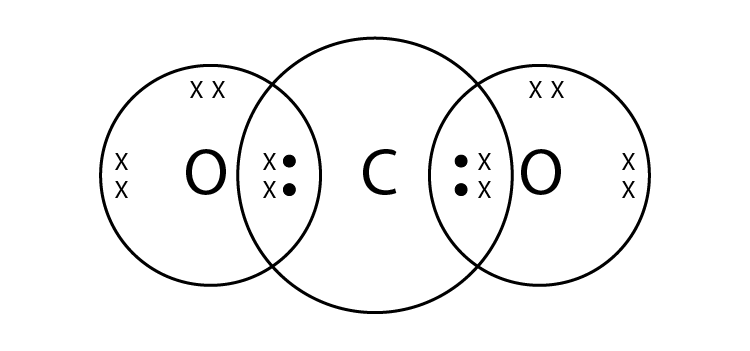4. What type of chemical bond is formed between:

(a) Potassium and bromine?

(b) Carbon and bromine?

Solution:

(a) Ionic bond is the bond between potassium and bromine

(b) Covalent bond is the bond between carbon and bromine

5. (a) What do we call those particles which have more or less electrons than the normal atoms?

(b) What do we call those particles which have more electrons than the normal atoms?

(c) What do we call those particles which have less electrons than the normal atoms?

Solution:

(a) Ions are the particles having more or less electron than the normal atoms

(b) Anions are the particles having more electrons than the normal atoms

(c) Cations are the particles having fewer electrons than the normal atom

6. (a) The atomic number of sodium is 11. What is the number of electrons in Na+?

(b) The atomic number of chlorine is 17. What is the number of electrons in Cl-?

Solution:

(a) No. of electrons in Na+= 10

(b) No. of electrons in Cl- = 18

7. The atomic number of an element X is 8 and that of element Y is 12. Write down the symbols of the ions you would expect to be formed from their atoms.

Solution:

X will form X2- as its atomic number is 8

Y will form Y2+ as its atomic number is 12

8. (a)Write down the electronic configuration of (i) magnesium atom, and (ii) magnesium ion. (At. No. of Mg = 12)

(b)Write down the electronic configuration of (i) sulphur atom, and (ii) sulphide ion, (At. No. of S = 16)

Solution:

(a) (i) Mg = 2, 8, 2 (ii) Mg2+ = 2, 8

(b) (i) S = 2, 8, 6 (ii) S2- = 2, 8, 8

9. What type of chemical bonds are present in a solid compound which has a high melting point, does not conduct electricity in the solid state but becomes a good conductor in the molten state?

Solution:

Ionic bonds are present in a solid compound which has a high melting point, does not conduct electricity in the solid state but becomes a good conductor in the molten state

10. State whether the following statement is true or false:

The aqueous solution of an ionic compound conducts electricity because there are plenty of free electrons in the solution.

Solution:

The statement is false. The aqueous solution of an ionic compound conducts electricity because there are plenty of free ‘ions’ in the solution.

11. What type of bonds are present in hydrogen chloride and oxygen?

Solution:

Covalent bond is present in hydrogen chloride and oxygen. Here sharing of electrons takes place.

12. Write the electron-dot structures for the following molecules:

(i) NaCl (ii) Cl2

Solution:

(i)

(ii)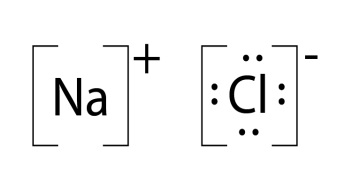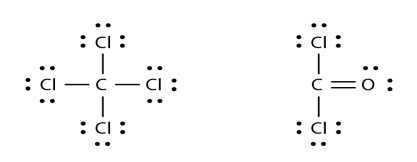13. What type of bonds are present in water molecule? Draw the electron-dot structure of water (H2O).

Solution:

Covalent Bonds are present in water molecule14. What type of bonds are present in methane (CH4) and solution chloride (NaCl)?

Solution:

Methane has covalent bonds and sodium chloride has ionic bonds.

15. State one major difference between covalent and ionic bonds and give one example each of covalent and ionic compounds.

Solution:

Ionic compounds are good conductors of electricity when dissolved in water or melted but covalent compounds do not conduct electricity. Example: NaCl is Ionic compound

Carbon dioxide is an example for covalent compounds.

16. What type of bonds are present in the following molecules? Draw their electron-dot structures.

(i) H2 (ii) CH4 (iii) Cl2 (iv) O2

Solution:

Covalent bonds are present in the given molecules

(i)

(ii)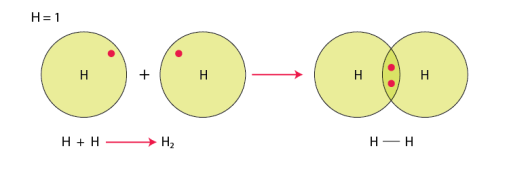(iii)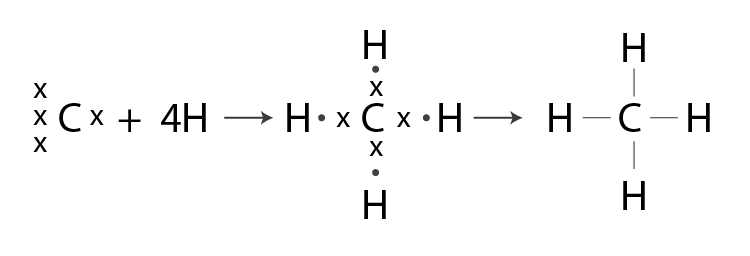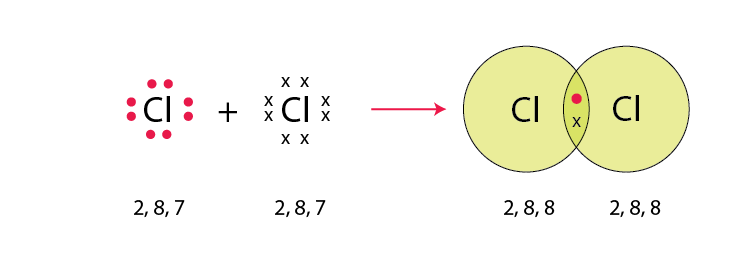(iv)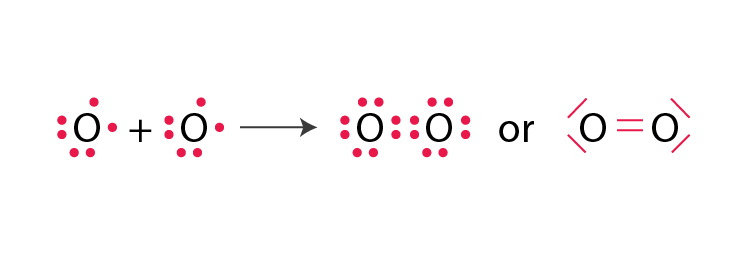17. Which inert gas electron configuration do the Cl atoms in Cl2 molecule resemble? What is this electron configuration?

Solution:

Argon is the inert gas electron configuration do the Cl atoms in Cl2. Its electronic configuration is 2, 8, 8.

18. Which of the following compounds are ionic and which are covalent?

Urea, Cane sugar, Hydrogen chloride, Sodium chloride, Ammonium chloride, Carbon tetrachloride, Ammonia, alcohol, Magnesium chloride.

Solution:

Ionic compounds: Sodium Chloride, Ammonium chloride and Magnesium chloride

Covalent compounds: Urea, Cane sugar, Hydrogen chloride, Carbon tetrachloride, Ammonia, Alcohol.

19. Give one example each of the following:

(i)A molecule containing a single covalent bond

(ii)A molecule containing a double covalent bond

(iii)A molecule containing a triple covalent bond

(iv)A compound containing an ionic bond

Solution:

(i) Hydrogen is the molecule containing single covalent bond.

(ii) Oxygen is the molecule containing double covalent bond.

(iii) Nitrogen is the molecule containing triple covalent bond.

(iv) Sodium chloride is the molecule containing an ionic bond.

Page no: 168

20. Fill in the blanks in the following sentences:

(i)Two atoms of the same element combine to form a molecule. The bond between them is known as _____ bond.

(ii) Two chlorine atoms combine to form a molecule. The bond between them is known as _____.

(iii) In forming oxygen molecule, _____ electrons are shared by each atom of oxygen.

(iv) In forming N2 molecule, _____ electrons are shared by each atom of nitrogen.

(v) The number of single covalent bonds in C2H2 molecule are _____.

(vi) Melting points and boiling points of ionic compounds are generally _____ than those of covalent compounds.

Solution:

(i) Covalent

(ii) Covalent

(iii) Two

(iv) Three

(v) Two

(vi) Higher

21. (a)What is a covalent bond? What type of bond exists in (i) CCl4, and (ii) CaCl2?

(b)What is an ionic bond? What type of bond is present in oxygen molecule?

Solution:

(a) Covalent bond is the bond formed by sharing of electrons between the atoms

In CCl4 the bond is covalent and in CaCl2 the bond is ionic

(b) Ionic bond is formed by the transfer of electrons from one atom to another

Covalent bond is present in oxygen molecule.

22. (a)What is an ion? Explain with examples.

(b)What is the nature of charge on (i) a cation, and (ii) an anion?

(c)Name the cation and anion present in MgCl2. Also write their symbols.

Solution:

(a) Ions are charged species. It is formed by loss or gain of an electron by an atom.

Example: Na+ is cation and Cl is anion.

(b)(i) Positive charge for cations (ii) Negative charge for anions

(c) Cation: Magnesium ion, Mg2+

Anion: Chloride ions, 2Cl

23. (a)What type of chemical bond is present in chlorine molecule? Explain your answer.

(b)Explain the formation of a chlorine molecule on the basis of electron theory of valency.

Solution:

(a) In chlorine molecule, covalent bond is present because whenever two atoms of same element combine to form a molecule, a covalent bond is formed.

(b) Chlorine is an electronegative atom. The atomic number is 17 and has a configuration of 2,8,8,7. Addition of one electron in the outermost shell will make octet. It acquire by sharing with another chlorine atom and form chlorine molecule. There will be a strong bond formation in between the two chlorine atoms and the bond is called covalent bond. Therefore the electronic configuration will become2,8,8. Thus it become stable

24. (a) Giving one example each, state what are (i) ionic compounds, and (ii) covalent compounds.

(b) Compare the properties of ionic compounds and covalent compounds.

Solution:

(a) (i)The compounds which are formed by transferring from one atom to another containing ionic bond is called ionic compounds

Example: Sodium chloride, NaCl

(ii) The compounds which are formed by sharing of electrons from one atom to another containing covalent bonds are called covalent compounds.

Example: Methane, CH4

(b) Ionic compounds are crystalline solids whereas covalent compounds are usually liquid or gases.

Ionic compounds have high melting and boiling points whereas covalent compounds have low melting and boiling points.

Ionic compounds are soluble in water whereas covalent compounds are insoluble in water.

25. Explain why:

(a)Covalent compounds have generally low melting points.

(b)Ionic compounds have generally high melting points.

Solution:

(a) Covalent compounds generally have low melting point because they are made up of electrically neutral molecules hence the force of attraction between the molecules will be very weak. Therefore less amount of energy is required to break these molecular forces

(b) Ionic compounds have strong electrostatic forces of attraction between ions. Thus it requires more energy to break the force and that is why ionic compounds have high melting point.

26. (a)Give two general properties of ionic compounds and two those of covalent compounds.

(b) State one test by which sodium chloride can be distinguished from sugar.

Solution;

(a)(i) Ionic compounds are soluble in water.

They are having high melting and boiling points.

(ii) Covalent compounds are insoluble in water.

They are having low melting and boiling point.

(b) Sodium chloride and sugar can be distinguished by electrical conductivity test. Sugar does not conduct electricity when dissolved in water as it is a covalent compound but sodium chloride conducts electricity when dissolved in water as it is an ionic compound.

27. (a) Explain why, ionic compounds conduct electricity in solution whereas covalent compounds do not conduct electricity.

(b) Which of the following will conduct electricity and which not?

MgCl2, CCl4, NaCl, CS2, Na2S

Solution:

(a) Ionic compounds are made up of electrically charged ions thus it conduct electricity whereas covalent compounds are made up of electrically neutral molecules so they do not conduct electricity.

(b) MgCl2, NaCl, Na2S Conduct electricity

CCl4, CS2 do not conduct electricity

28. (a) Name one ionic compound containing chlorine and one covalent compound containing chlorine.

(b) How will you find out which of the water soluble compound A or B is ionic?

Solution:

(a) Ionic compound: Sodium chloride, NaCl.

Covalent compound: Carbon tetrachloride, CCl4

(b) Out of A and B, the ionic compound will be those which conduct electricity in aqueous solution.

29. Explain why, a solution of cane sugar does not conduct electricity but a solution of common salt is a good conductor of electricity.

Solution:

Common salt is a good conductor of electricity as it is an ionic compound they are made up of charged ions whereas sugar cane is covalent compound they are made up electrically neutral molecule hence it does not conduct electricity.

30. Give the formulae of the compounds that would be formed by the combination of the following pairs of elements:

(a) Mg and N2

(b) Li and O2

(c) Al and Cl2

(d) K and H

Solution:

(a) Mg3N2

(b) Li2O

(c) AlCl3

(d) KH

31. (a)What are noble gases? What is the characteristic of the electronic configuration of noble gases?

(b)What is the cause of chemical bonding (or chemical combination) of atoms of elements?

Solution:

(a) Noble gases or inert gases are those which do not combine with other elements and they are in group 18. They are unreactive stable elements. Helium, neon, argon, krypton, xenon and radon are called noble gases. The electronic configuration of noble gases will have 8 electrons in the outermost shell except helium. Therefore they are the most stable.

(b) Atoms form chemical bonds to achieve stability by acquiring inert gas electron configuration.

32. (i)Write electron-dot structures for magnesium and oxygen.

(ii)Show the formation of MgO by the transfer of electrons.

(iii)What are the ions present in this compound?

Solution:

(i)(ii)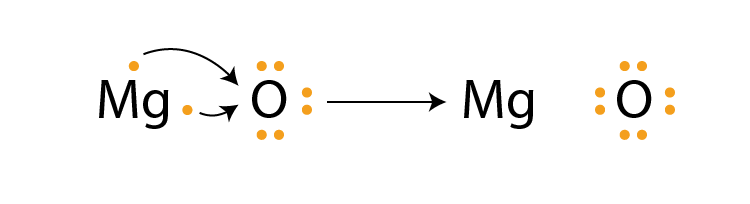(iii) Both positive and negative ions. Mg2+ and O2- respectively

33. Draw the electron-dot structure of a hydrogen chloride molecule:

(i) Which inert gas does the H atom in HCl resemble in electron arrangement?

(ii) Which inert gas does the Cl atom in HCl resemble in electron arrangement?

Solution:(i) Helium atom is the inert gas which resembles H atom in HCl

(ii) Argon is the inert gas which resembles Cl in HCl

34. What type of bonding would you expect between the following pairs of elements?

(i) Calcium and Oxygen

(ii) Carbon and Chlorine

(iii) Hydrogen and Chlorine.

Solution:

(i) Ionic bonding exists between calcium and oxygen

(ii) Covalent bonding will be there in between carbon and chlorine

(iii) Covalent bond is there between hydrogen and chlorine.

Page no: 169

35. Describe how sodium and chlorine atoms are changed into ions when they react with each other to form sodium chloride, NaCl. What is the name given to this type of bonding?

Solution:

Sodium has an electronic configuration of 2,8,1 and its atomic number is 11. It has one electron in its valence shell. Chlorine has an electronic configuration of 2,8,7 and atomic number is 17. Chlorine needs one electron to complete its octet. Sodium donates one electron to chlorine atom and forms sodium ion, Na+ and chlorine accept that electron and form chlorine ion Cl

This type of bonding is called ionic bonding

36. What is the difference between a cation and an anion? How are they formed? Give the names and symbols of one cation and one anion.

Solution:

Cations are those which are positively charged ions and it is formed by losing of one or more electron by an atom. For eg: Sodium loses 1 electron to form sodium ion Na+ ion

Anions are those which are negatively charged ions and it is formed by gaining of one more electrons by an atom. For eg; Chlorine gains one electron and form chlorine ion Clion

37. Using electron-dot diagrams which show only the outermost shell electrons, show how a molecule of nitrogen, N2, is formed from two nitrogen atoms. What name is given to this type of bonding?

Solution:

Nitrogen has 5 electrons in its outermost shell, in order to complete octet it needs 3 more electrons and hence it combines with another nitrogen atom to form a molecule of nitrogen gas.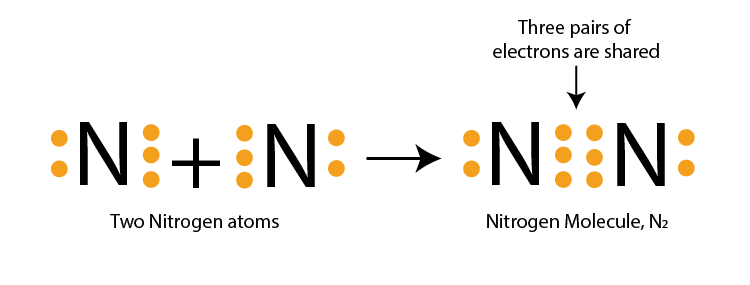38. Draw the electron-dot structures of the following compounds and state the type of bonding in each case:

(i) CO2

(ii) MgO

(iii) H2O

(iv) HCl

(v) MgCl2

Solution:

(i)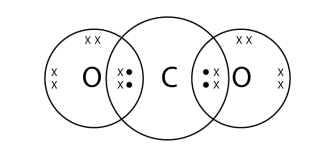Covalent bond

(ii)Ionic bond

(iii)Covalent bond

(iv)Covalent bond

(v)Ionic bond

39. Using electron-dot diagrams which show only the outermost shell electrons, show how a molecule of oxygen, O2, is formed from two oxygen atoms. What is the name given to this type of bonding?

Solution: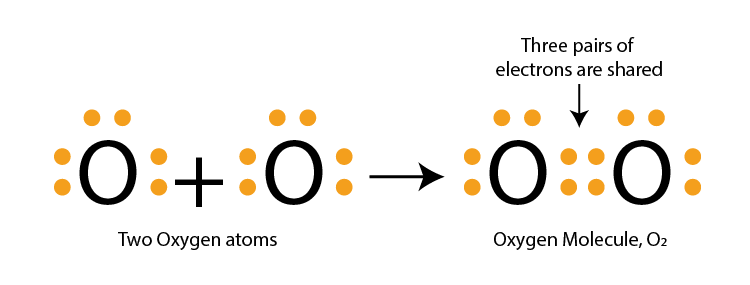Oxygen atom has 6 electrons in its outermost electron and it needs 2 electrons more to complete its octet. Hence combines with another oxygen atom and forms a molecule of oxygen

This type of bonding is called double covalent bond.

40. Draw the electron-dot structures of the following compounds and state the type of bonding in each case:

(i) KCl

(ii) NH3

(iii) CaO

(iv) N2

(v) CaCl2

Solution:

(i)Ionic bond

(ii)Covalent bond

(iii)Ionic bond

(iv)Covalent bond

(v)Ionic bond

41. Explain why, a salt which does not conduct electricity in the solid state becomes a good conductor in molten state.

Solution:

In the solid ion compounds, ions are held together by a strong electrostatic forces therefore free moving of ions are restricted. When it dissolves in water or melts it, the crystal structure will break and ions will move freely and conducts electricity. So an aqueous solution of ionic compound conducts electricity and there are plenty of free ions which are able to conduct electric current.

42. (a) Write down the electronic configuration of (i) sodium atom, and (ii) chlorine atom.

(b) How many electrons are there in the outermost shell of (i) a sodium atom, and (ii) a chlorine atom?

(c) Show the formation of NaCl from sodium and chlorine atoms by the transfer of electron(s).

(d) Why has sodium chloride a high melting point?

(e) Name the anode and the cathode used in the electrolytic refining of impure copper metal.

Solution:

(a) ((i) Sodium – 2, 8, 1 (ii) Chlorine – 2, 8, 7

(b) (i) Sodium = 1 (ii) Chlorine = 7

(c)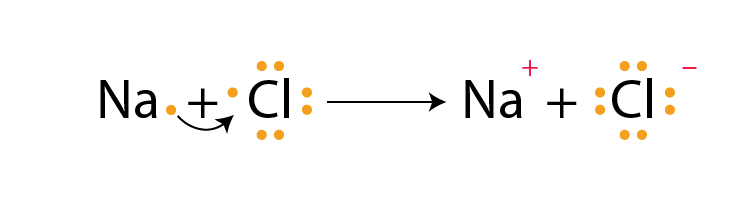(d) Sodium chloride has high melting point because it is an ionic compound and they are made up of electrically charged species called ions which are positive and negative ions. There exists a strong force of attraction which is difficult to break and requires high amount of energy.

(e) (e) Anode: Thick block of impure copper metal; Cathode: Thin strip of pure copper metal.

43. (a) Write the electron arrangement in (i) a magnesium atom, and (ii) an oxygen atom.

(b) How many electrons are there in the valence shell of (i) a magnesium atom, and (ii) an oxygen atom.

(c) Show on a diagram the transfer of electrons between the atoms in the formation of MgO.

(d) Name the solvent in which ionic compounds are generally soluble.

(e) Why are aqueous solutions of ionic compounds able to conduct electricity?

Solution;

(a) (i) Magnesium – 2, 8, 2 (ii) Oxygen – 2, 6

(b) (i) Magnesium = 2 (ii) Oxygen = 6

(c)(d) Water is the solvent where an ionic compound dissolves.

(e) An aqueous solution of ionic compound conducts electricity because there are ions in the solution which are responsible for conducting electric current.

44. (a) What is the electronic configuration of (i) a sodium atom, and (ii) an oxygen atom?

(b) What is the number of outermost electrons in (i) a sodium atom, and (ii) an oxygen atom?

(c) Show the formation of Na2O by the transfer of electrons between the combining atoms.

(d) Why are ionic compounds usually hard?

(e) How is it that ionic compounds in the solid state do not conduct electricity but they do so when in molten state?

Solution:

(a) (i) Sodium: 2,8,1 (ii) Oxygen: 2,6

(b) (i) Sodium :1 (ii) Oxygen: 6

(c)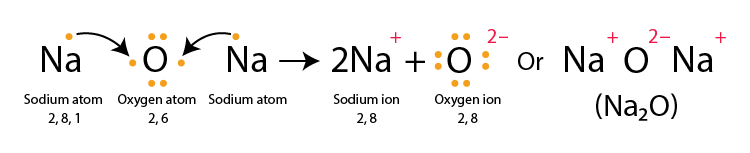(d) Ionic compounds are usually hard because their oppositely charged ions attracts each other strongly and forms a crystal structure

(e) Solid ionic compound are made up of ions but do not conduct electricity. Ions are held together by strong electrostatic force and movement is restricted when it gets dissolved in water the crystal structure is broken and ions will start moving freely thus it conducts electricity.

45. (a) Write down the electron arrangement in (i) a magnesium atom, and (ii) a chlorine atom.

(b) How many electrons are there in the valence shell of (i) a magnesium atom, and (ii) a chlorine atom.

(c) Show the formation of magnesium chloride from magnesium and chlorine by the transfer of electrons.

(d) State whether magnesium chloride will conduct electricity or not. Give reason for your answer.

(e) Why are covalent compounds generally poor conductors of electricity?

Solution:

(a) (i) Magnesium: 2, 8, 2 (ii) Chlorine: 2, 8, 7

(b) (i) Magnesium atom: 2 (ii) Chlorine atom: 7

(c)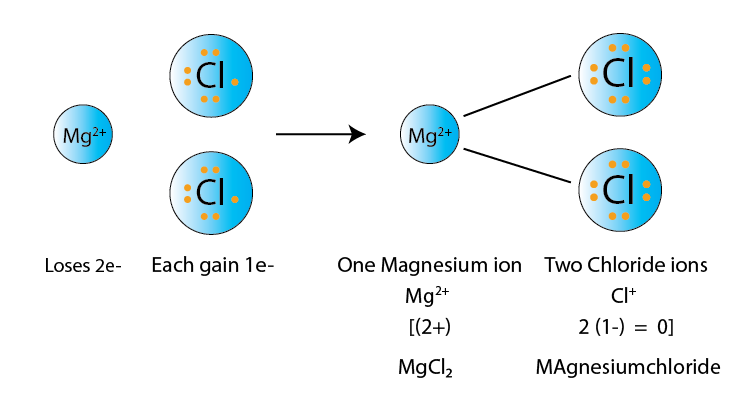(d) Magnesium chloride will conduct electricity because it is an ionic compound. It conducts electricity because they contain positive and negative ions which is responsible for the electric current.

(e) Covalent compounds are generally poor conductors of electricity because they are held together by electrically neutral molecule. They do not have ions which conducts electricity

Multiple Choice Questions(MCQ’s)

46. The atomic number of an element X is 19. The number of electron in its ion X+ will be:

(a) 18

(b) 19

(c) 20

(d) 21

Solution:

47.The atomic number of an element Y is 17. The number of electrons in its Y will be:

(a) 17

(b) 18

(c) 19

(d) 20

Solution:

48. The atomic numbers of four elements A,B,C and D are 6,8,10 and 12 respectively. The two elements which can react to form ionic bonds (or ionic compounds) are;

(a) A and D

(b) B and C

(c) A and C

(d) B and D

Solution:

49. The atomic numbers of four elements PQ,R and S are 6,10,12 and 17 respectively. Which two elements can combine to form covalent compounds ?

(a) P and R

(b) Q and S

(c) P and S

(d) R and S

Solution:

50. The solution of one of the following compound will not conduct electricity. This compound is:

(a) NaCl

(b) CCl4

(c) MgCl2

(d) CaCl2

Solution:

51. The electronic configuration of three elements X,Y and Z are:

X:32 Y: 2,8,7 Z: 2,8,2

Which of the following is correct regarding these elements ?

Solution:

(a) X is a metal

(b) Y is a metal

(c) Z is a non-metal

(d) Y is a non-metal and Z is a metal

Solution:

52. Which one of the following property is generally not exhibited by ionic compounds?

(a) Solubility in water

(b) Electrical conductivity in solid state

(c) High melting and boiling points

(d) Electrical conductivity in molten state

Solution:

53. The electrons present in the valence shell of a noble gas atom can be:

(a) 8 only

(b) 2 only

(c) 8 or 2

(d) 8 or 4

Solution:

54. The atomic number of an element X is 16. The symbol of ion formed by an atom of this element will be :

(a) X2-

(b) X3+

(c) Y2-

(d) X

Solution:

55. The number of protons in the nucleus of one atom of an element Y is 5. The symbol of ion formed by an atom of this element will be:

(a) Y3-

(b) Y2+

(c) Y2-

(d) Y3+

Solution:

56. Out of KCl, HCl, CCl4 and NaCl, the compounds which are not ionic are:

(a) KCl and HCl

(b) HCl and CCl4

(c) CCl4 and NaCl

(d) KCl and CCl4

Solution:

57. Element X reacts with element Y to form a compound Z. During the formation of compound Z atoms of X lose one electron each whereas atoms of Y gain one electron each. Which of the following property is not shown by compound Z?

(a) High melting point

(b) Low melting point

(c) Occurrence as solid

(d) Conduction of electricity in molten state.

Solution:

58. One of the following compounds is not ionic in nature. This compound is:

(a) Lithium chloride

(b) Ammonium chloride

(c) Calcium chloride

(d) Carbon tetrachloride

Solution:

59.The rechargeable battery used in mobile phone hand set is usually:

(b) Sodium ion battery

(c) Hydrogen ion battery

(d) Lithium ion battery

Solution:

60. The number of protons in one atom of an element X is 8. What will be the number of electrons in its ion X2-?

(a) 8

(b) 9

(c) 10

(d) 11

Solution:

61. If the number of protons in one atom of an element Y is 20, then the number of electron in its ion Y2+ will be:

(a) 20

(b) 19

(c) 18

(d) 16

Solution:

62. The noble gas having two electron in its valence shell is:

(a) Ar

(b) Ne

(c) He

(d) Kr

Solution:

63. A covalent molecule having a double bond between its atom is:

(a) Hydrogen

(b) Oxygen

(c) Water

(d) Ammonia

Solution:

64. The molecules having triple bond in them are:

(a) Oxygen and ethyne

(b) Carbon dioxide and ammonia

(c) Methane and ethane

(d) Nitrogen and ethyne

Solution:

65. One of the following contains a double bond as well as single bomds. This is:

(a) CO2

(b) O2

(c) C2H4

(d) C2H2

Solution:

66. Which of the following has a triple bond as well as single bonds?

(a) Ethene

(b) Methane

(c) Ethyne

(d) Nitrogen

Solution:

Very Short Answer Type Questions Page no: 191

1. A zinc ore gave CO2 on treatment with a dilute acid. Identify the ore and write its chemical formula.

Solution:

Calamine is the zinc ore which gives carbon dioxide on treatment with a dilute acid. ZnCO3

2. What chemical process is used for obtaining a metal from its oxide?

Solution:

Reduction is the process used for obtaining a metal from its oxide.

3. State two ways to prevent the rusting of iron.

Solution:

Rusting of iron can be prevented by

(i) Painting the surface

(ii) By applying grease or oil.

4. What is meant by galvanisation ? Why is it done?

Solution:

Galvanisation is the process of depositing a thin layer of zinc metal on iron objects. It is done to prevent the iron from rusting.

5. Name the metal which is used for galvanising iron.

Solution:

Zinc is used for galvanising iron

6. Explain why, iron sheets are coated with zinc.

Solution:

Zinc is a reactive metal and the action of air on zinc metal forms a very thin coating of zinc oxide all over which is hard and impervious to air and prevents corrosion of zinc metal as well as iron.

7. Why do we apply paint on iron articles?

Solution:

Iron objects are painted in order to prevent from corrosion. Sometimes air and moisture will come in contact and there will be a great chance of rusting of iron

8. Give reason for the following :

Carbonate and sulphide ores are usually converted into oxides during the process of extraction of metals.

Solution:

Carbonate and sulphide ores are usually converted into oxides because it is easier to obtain metals from their oxides than from carbonates or sulphides. Reduction takes place here

9. Name a reducing agent that may be used to obtain manganese from manganese dioxide.

Solution:

Aluminium powder can be used as the reducing agent to obtain manganese from manganese dioxide

10. Name an alloy of lead and tin.

Solution:

Solder is an alloy of tin and lead

11. Give the composition of an alloy called solder. State its one property and one use.

Solution:

Solder is the alloy of lead and tin. It comprises of these 2 elements in the ratio of 50-50. It has low melting point and used for soldering wires.

12. What is an amalgam?

Solution:

Amalgam is a mixture of metals which is an alloy of mercury.

13. How many carats is pure gold? Why is pure gold not suitable for making ornaments?

Solution:

Pure gold is 24 carats, it is soft thus cannot be sued for making ornaments.

14. Name one method for the refining of metals.

Solution:

Electrolytic refining is one method used for refining the metals.

15. State two conditions for the rusting of iron.

Solution:

Rusting of iron due to 2 reasons one is presence of air and the other one is presence of water. Oxygen presence and moisture can cause rusting.

16. In one method of rust prevention, the iron is not coated with anything. Which is this method?

Solution:

Rusting of iron can be prevented by alloying iron with chromium and nickel to make stainless steel.

17. Name two alloys of iron. What elements are present in these alloys?

Solution:

Two alloys of iron are steel and stainless steel. Steel contains 0.1-1.5 percent iron. Iron, nickel and chromium are present in stainless steel.

18. Give reason for the following :

Silver, gold and platinum are used to make jewellery.

Solution:

Gold, silver and platinum are less reactive metals. They do not react with air, water, acids and alkalis. They are used to make jewellery because they do not corrode easily

19. Which metal becomes black in the presence of hydrogen sulphide gas in air?

Solution:

Silver reacts with hydrogen sulphide gas in air and forms a thin black layer of silver sulphide on it.

20. Name the gas in air which tarnishes silver articles slowly.

Solution:

Hydrogen sulphide gas in the air tarnishes silver articles slowly. Silver reacts with hydrogen sulphide gas in air and forms a thin black layer of silver sulphide on it.

21. Silver metal does not combine easily with oxygen but silver jewellery tarnishes after some time. How?

Solution;

The silver articles combines slowly with hydrogen and the hydrogen sulphide gas present in air forms a thin layer of silver sulphide. Due to the coating silver objects tarnishes.

22. Write the composition of the alloy called bronze. Give two uses of bronze.

Solution:

Bronze is an alloy of 90% copper and 10% tin. It is used to make statues and coins.

23. Why does a new aluminium vessel lose shine so soon after use?

Solution:

Due to corrosion of aluminium metal when exposed to moist air the new vessel loses its shine. It forms a layer of aluminium oxide when come to contact with air.

24. Why do gold ornaments look new even after several years of use?

Solution:

Gold is an unreactive metal. So that they do not corrode easily by reacting with atmospheric air, water vapour and other gases. That is why gold looks new even after several years.

25. Name two metals which are highly resistant to corrosion.

Solution:

Gold and platinum does not corrode because they are unreactive metals

26. Which property of ‘solder’ alloy makes it suitable for welding electrical wires?

Solution:

Solder is an alloy of lead and tin. The melting point of solder is very low and lower than the constituting metals. Thus they are suitable for welding electrical wires.

27. Explain why, carbon cannot reduce oxides of sodium or magnesium.

Solution:

Carbon is less reactive than magnesium and sodium. Carbon can reduce oxides of zinc but cannot reduce oxides of sodium and magnesium.

28. Why are the metals like Na, K, Ca and Mg never found in their free state in nature?

Solution:

All these metals are high up in the reactivity series and are so reactive. That is why these metals are not found in their free states.

29. Name one metal each which is extracted by:

(a) reduction with carbon (b) electrolytic reduction

(c) reduction with aluminium (d) reduction with heat alone

Solution:

(a) Zinc

(b) Sodium

(c) Manganese

(d) Mercury

30. Fill in the following blanks with suitable words:

(a) The corrosion of iron is called _______.

(b) _______ and _______ are necessary for the rusting of iron.

(c) The process of depositing a thin layer of zinc on iron articles is called _______.

(d) Tiffin boxes are electroplated with _______ but car bumpers are electroplated with _______ to protect them from rusting.

(e) The corrosion of copper produces a _______ coating of basic copper carbonate on its surface.

(j) Brass is an alloy of copper and _______.

(g) Bronze is an alloy of copper and _______.

(h) The non-metal present in steel is _______.

(i) The alloy in which one of the metals is mercury is called an _______.

(j) The electrical conductivity and melting point of an alloy is _______ than that of pure metals.

(k) The rocky material found with ores is called _______.

Solution:

(a) rusting

(b) air; water

(c) galvanisation

(d) tin; chromium

(e) green

(j) zinc

(g) tin

(h) carbon

(i) amalgam

(j) less

(k) gangue

Short Answer Type Questions Page no: 192

31. How is manganese extracted from manganese dioxide, MnO2? Explain with the help of an equation.

Solution:

Manganese metal is extracted by the reduction of its oxide with aluminium powder as the reducing agent. Thus, when manganese dioxide is heated with aluminium powder, then manganese metal is formed.

3MnO2(s) + 4Al(s) → 3Mn(l) + 2Al2O3 + Heat

32. What is a thermite reaction? Explain with the help of an equation. State one use of this reaction.

Solution:

The reduction of a metal oxide to form metal by using aluminium powder as a reducing agent is called a thermite reaction.

A mixture of Iron (III) oxide and aluminium powder is ignited with a burning magnesium ribbon. Aluminium reduces iron oxide to produce iron metal with the evolution of a lot of heat. Due to this heat, iron metal is produced in the molten state. This molten iron is then poured between the broken iron pieces to weld them

Fe2O3(s) + 2Al(s) → 2Fe(l) + Al2O3(s) + Heat

33. Which one of the methods given in column I is applied for the extraction of each of the metals given in column II:

 Column I Column II Electrolytic reduction Reduction with carbon Reduction with aluminium Aluminium Zinc Sodium Iron Manganese Tin

Solution:

Electrolytic reduction: Aluminium and Sodium;

Reduction with carbon : Zinc, Iron and Tin;

Reduction with aluminium: Manganese

34. (a) Give reason why copper is used to make hot water tanks but steel (an alloy of iron) is not.

(b) Explain why, the surface of some metals acquires a dull appearance when exposed to air for a long time.

Solution:

(a) Copper is used to make hot water tanks because it does not corrode easily but steel rust in the presence of water.

(b) The surface of some metals acquires a dull appearance when exposed to air for a long time because of the formation of an oxide layer on the surface of the metal.

35. (a) Why does aluminium not corrode right through?

(b) What is meant by ‘anodising’ ? Why is it done?

Solution:

(a)Aluminium is more reactive than iron and it forms a oxide layer when come in contact with air that is why aluminium do not corrode

(b) Anodising is the process of thickening of aluminium oxide layer on the surface of aluminium objects by electrolysis. It is done to provide more protection to the aluminium object from corrosion.

36. (a) Why is an iron grill painted frequently?

(b) Explain why, though aluminium is more reactive than iron, yet there is less corrosion of aluminium when both are exposed to air.

Solution:

(a)In order to prevent rusting iron grill is painted frequently.

(b) Aluminium is reactive and forms an oxide layer on its surface when comes to contact with air. That is why there is less corrosion of aluminium when both exposed to air.

37. (a) Name the method by which aluminium metal is extracted.

(b) Give the name and chemical formula of one ore of copper.

(c) How is zinc extracted from its carbonate ore (calamine)? Explain with equations.

Solution:

(a) Electrolytic reduction is the method used to extract aluminium metal.

(b) Copper glance (Cu2S)

(c) When calamine ore is heated strongly in the absence of air, it decomposes to form zinc oxide and carbon dioxide.

ZnCO3 → ZnO + CO2

Then, Zinc oxide is heated with carbon and zinc metal is produced

ZnO + C → Zn + CO

38. (a) Name two metals which occur in nature in free state as well as in combined state.

(b) Name one ore of manganese. Which compound of manganese is present in this ore? Also write its chemical formula.

(c) A zinc ore on heating in air forms sulphur dioxide. Describe briefly any two stages involved in the conversion of this concentrated ore into zinc metal.

Solution:

(a) Copper and silver occur in nature in free states as well as in combined state.

(b) Pyrolusite; Manganese dioxide; MnO2

(c) When zinc sulphide is strongly heated in air (roasted), it forms zinc oxide and sulphur dioxide.

(d) Reduction: Zinc oxide obtained is heated with carbon to form zinc metal.

39. How does the method used for extracting a metal from its ore depend on the metal’s position in the reactivity series? Explain with examples.

Solution:

Extraction of metal from its concentrated ore is essentially a process of reduction of the metal compound present in it.

Manganese metal is obtained by the reduction of its oxide with aluminium powder and not carbon. This is because carbon is less reactive than manganese. Carbon which is a non-metal is more reactive than zinc and can be placed above zinc in reactive series.

40. Explain giving one example, how highly reactive metals (which are high up in the reactivity series) are extracted.

Solution:

The highly reactive metals are extracted by the electrolytic reduction of their molten chlorides or oxides.

Example: Sodium metal is extracted by the electrolytic reduction of molten sodium chloride. When electric current is passed through molten sodium chloride, it decomposes to form sodium metal and chlorine gas.

2NaCl(l) → 2Na(s) + Cl2(g)

41. Describe with one example, how moderately reactive metals (which are in the middle of reactivity series) are extracted.

Solution:

The moderately reactive metals are extracted by the reduction of their oxides with carbon, aluminum sodium or calcium.

Example:

When zinc sulphate is strongly heated in air if forms zinc oxide and sulphur dioxide. This is called roasting. Zinc oxide is heated with carbon to form zinc metal. This is called reduction.

42. How are the less reactive metals (which are quite low in the reactivity series) extracted? Explain with the help of an example.

Solution:

The less reactive metals are extracted by the reduction of their oxides by heat alone.

Eg; Mercury(II) sulphide ore is roasted in air when mercury (II) oxide is formed. Under 300℃ Mercury oxide is heated and forms mercury metal.

43. What is meant by refining of a metal? Name the most widely used method for the refining of impure metals obtained by various reduction processes. Describe this method with the help of a labelled diagram by taking the example of any metal.

Solution:

The process of purifying impure metal is called refining of metals.

Electrolytic refining is the most widely used method for the refining of impure metals obtained by various reduction processes.

In an electrolytic tank, acidified copper sulphate (CuSO4 + dilute H2O4) solution forms the electrolyte. A block of impure copper is made into an anode by connecting the positive terminal of a power supply (battery). A thin strip of highly pure copper metal is the cathode of the cell. The negative terminal of the power supply is connected to it.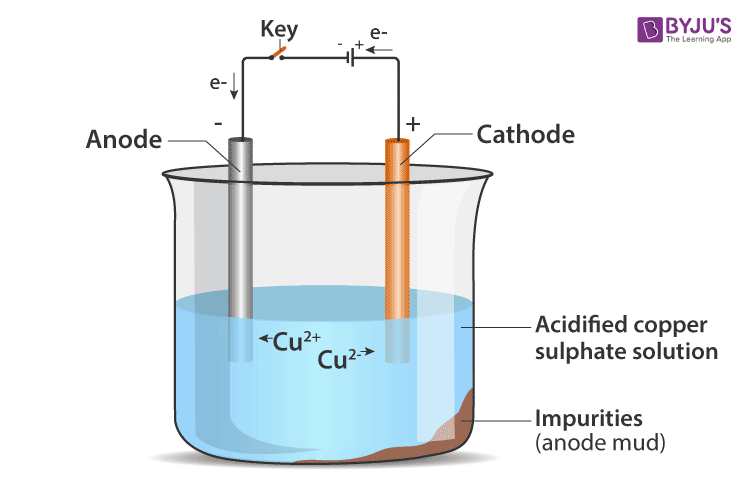A small electric current is passed through the cell. Atoms from the anode enter the electrolyte. The copper from the anode gets converted into copper sulphide. An equal number of copper atoms from the solution get deposited on the cathode. This is to keep the concentration of the solution constant. Impurities from the anode block either remain in solution or collect below the anode, as they are unable to displace copper from the sulphate solution. The insoluble impurities remain in the electrolyte and are called anode mud.

Copper sulphate solution contains ions of Cu+ and SO4. The following reactions take place at the anode and cathode when an electric current is passed.

At cathode: Cu2+ + 2e→ Cu

At anode: Cu -2e- → Cu2+

Pure copper is removed from cathode. Some metals like gold, silver is present in the anode and can be recovered separately.

44. (a) Define the terms (i) mineral (ii) ore, and (iii) gangue.

(b) What is meant by the ‘concentration of ore’?

(c) Name one ore of copper (other than cuprite). Which compound of copper is present in this ore? Also, write its chemical formula.

Solutions:

(a) (i) Minerals are those in which the metal or compounds that are found on earth.

(ii) Ores are those minerals from which the metals can be extracted conveniently and profitably.

(iii) Gangue is the impurities like sand, rocky material present in the ore

(b) Before extracting metal from its ore it is necessary to remove the gangue and after the removal we will get a ore which is concentrated. This is called concentration of ore also called enrichment of ore.

(c) Ore: Copper glance; Copper(I) Sulphide

Page no: 193

45. Explain how, a reduction reaction of aluminium can be used for welding cracked machine parts of iron. Write a chemical equation for the reaction involved.

Solution:

A mixture of Iron (III) oxide and aluminium powder is ignited with a burning magnesium ribbon. Aluminium reduces iron oxide to produce iron metal with the evolution of lot of heat. Due to this heat, iron metal is produced in the molten state. This molten iron is poured between broken iron parts of the machine to weld them (to join them).

Fe2O3(s) + 2Al(s) → 2Fe(l) + Al2O3(s) + Heat

46. (a) What is corrosion?

(b) Name any two metals which do not corrode easily.

(c) What is the corrosion of iron known as?

(d) Explain why, aluminium is a highly reactive metal, still it is used to make utensils for cooking.

Solution:

(a) Corrosion is the action of air, moisture or a chemical on their surface by a metal

(b) Gold and platinum

(c) Rusting

(d) Aluminium begins to corrode quickly when it comes in contact with moist air. It form an oxide layer over the metal. It prevents corrosion. Aluminium is used for making utensils.

47. What is meant by ‘rusting of iron’? With the help of labelled diagrams, describe an activity to find out the conditions under which iron rusts.

Solution:

When an iron object is left in damp air (or water) for a considerable time, it gets covered with a red-brown flaky substance called rust. This is called rusting of iron.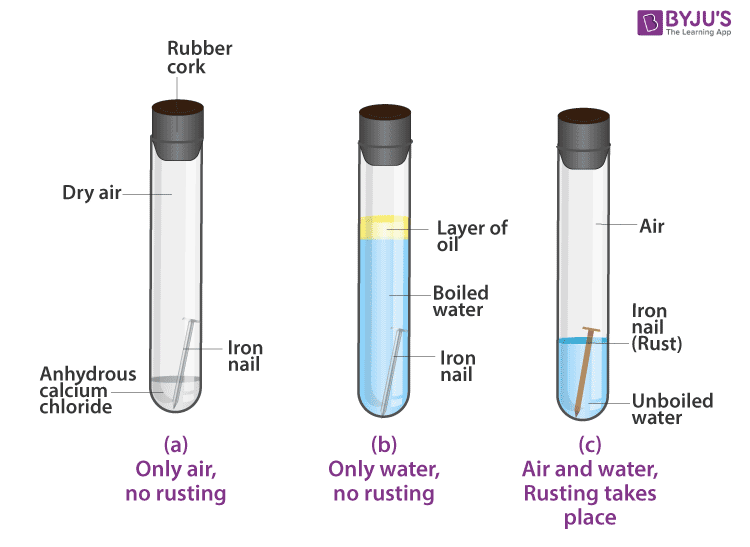1. In the first test-tube containing iron nail, we put some anhydrous calcium chloride and close its mouth with a tight cork. Anhydrous calcium chloride absorbs water moisture from the damp air present in the test-tube and make it dry. In this way, the iron nail in the first test-tube is kept in dry air (having no water vapour in it).

2. In the second test-tube containing iron nail, we put boiled distilled water. Boiled water does not contain any dissolved air (or oxygen) in it (this is because the process of boiling removes all the dissolved air from it). A layer of oil is put over boiled water in the test-tube to prevent the outside air from mixing with boiled water. In this way, the iron nail in the second test-tube is kept in air free boiled water.

3. In the third test-tube containing an iron nail, we put unboiled water so that about two-thirds of nail is immersed in water and the rest is above the water, exposed to damp air. In this way, the iron nail in the third test-tube has been placed in air and water together.

The mouth of all three test tubes is closed with a cork and it is kept aside for about one week.

After one week, we observe the iron nails kept in all the three test-tubes, one by one. We find that (i) No rust is seen on the surface of iron nail kept in dry air (water-free air) in the first test-tube. This tells us that rusting of iron does not take place in air alone.

(ii) No rust is seen on the surface of iron nail kept in air-free, boiled water in the second test-tube. This tells us that rusting of iron does not take place in water alone.

(iii) Red-brown rust is seen on the surface of iron nail kept in the presence of both air and water together the third test-tube. This tells us that rusting of iron takes place in the presence of both air and water together.

48. (a) What is an alloy? How is an alloy made?

(b) What elements are present in steel? How are the properties of steel different from those of pure iron?

(c) Give the constituents and one use of brass.

Solution:

(a) An alloy is a homogenous mixture of metals and is prepared by mixing up the various metals in molten state and cooling it under room temperature.

(b) Steel comprises of carbon and iron.

Alloy of iron is hard and strong and less rusts compared to pure iron.

(c) Brass is of copper and zinc

It is used to make utensils.

49. (a) Name two metals which resist corrosion due to the formation of a thin, hard and impervious layer of oxide on their surface.

(b) Name five methods of preventing rusting of iron.

(c) What are the constituents of stainless steel? What are the special properties of stainless steel?

Solution:

(a) Aluminium and zinc resist corrosion

(b) (i) Painting

(ii) Applying grease or oil

(iii) Galvanisation

(iv) Tin and chromium plating

(v) Alloying to form stainless steel.

(c) Stainless steel contains iron, chromium and nickel.

It is strong enough and does not rust.

50. (a) Name an alloy of copper. State its chemical composition and any one use.

(b) Explain why, when a copper object remains in damp air for a considerable time, a green coating is formed on its surface. What is this process known as ?

Solution:

(a) Brass: It contains Copper (Cu) – 80% and Zinc (Zn) – 20%. It is used for making cooking utensils.

(b) Copper reacts slowly with the carbon dioxide and water to form a green coating of basic copper carbonate on the surface of the object. The formation of this green coating of basic copper carbonate corrodes and the process is called corrosion of copper.

51. (a) How does the painting of an iron object prevent its rusting?

(b) How does the electrical conductivity of copper alloys, brass and bronze, differ from that of pure copper?

(c) What is meant by 22 carat gold? Name the metals which are usually alloyed with gold to make it harder.

Solution:

(a) When paint is applied on a surface it prevents air and moisture to come in contact with the object and prevents rusting.

(b) The electrical conductivity of copper alloys like brass and bronze is less than that of pure copper.

(c) 22 carat gold is 22 part of pure gold is alloyed with 2 parts of either silver or copper for making ornaments. Silver and copper are usually alloyed with gold to make it harder.

52. Explain giving equation, what happens when:

(a) ZnCO3 is heated in the absence of air?

(b) a mixture of Cu2O and Cu2S is heated?

Solution:

(a0 When zinc carbonate is heated strongly in the absence of air, it decomposes to form zinc oxide and carbon dioxide.

ZnCO3(s) → ZnO(s) + CO2

(b) When copper (I) oxide reacts with copper (I) sulphide, it forms copper metal and sulphur dioxide.

2Cu2O(s) + Cu2S → 6Cu(s) + SO2(g)

53. (a) For the reduction of a metal oxide, suggest a reducing agent other than carbon.

(b) Explain why, an aqueous solution of sodium chloride is not used for the electrolytic extraction of sodium metal.

Solution:

(a) Aluminium can be used as a reducing agent other than carbon.

(b) If electrolysis is done in an aqueous solution of sodium chloride the sodium metal produce at cathode and reacts with water to form sodium hydroxide. Thus electrolysis of aqueous solution of sodium chloride will produce sodium hydroxide but not sodium metal.

54. How are metals refined by the electrolytic process? Describe the electrolytic refining of copper with the help of a neat labeled diagram.

Solution:For the refining of an impure metal by the process of electrolysis, a thick block of impure metal is made anode (connected to +ve terminal of the battery) and a thin strip of the pure metal is made cathode (connected to -ve terminal of battery). A water soluble salt (of the metal to be refined) is taken as electrolyte. On passing current, impure metal dissolves from the anode and goes into the electrolyte solution. And pure metal from the electrolyte deposits on the cathode.

Electrolytic refining of copper: In an electrolytic tank, acidified copper sulphate (CuSO4 + dilute H2O4) solution forms the electrolyte. A block of impure copper is made into an anode by connecting the positive terminal of a power supply (battery). A thin strip of highly pure copper metal is the cathode of the cell. The negative terminal of the power supply is connected to it.

A small electric current is passed through the cell. Atoms from the anode enter the electrolyte. The copper from the anode gets converted into copper sulphide. An equal number of copper atoms from the solution get deposited on the cathode. This is to keep the concentration of the solution constant. Impurities from the anode block either remain in solution or collect below the anode, as they are unable to displace copper from the sulphate solution. The insoluble impurities remain in the electrolyte and are called anode mud.

Copper sulphate solution contains ions of Cu+ and SO4. The following reactions take place at the anode and cathode when an electric current is passed.

At cathode: Cu2+ + 2e→ Cu

At anode: Cu -2e- → Cu2+

Pure copper is removed from cathode. Some metals like gold, silver is present in the anode and can be recovered separately.

55. (a) Name the chemical compound which is electrolysed in molten state to obtain aluminium metal. Which gas is evolved during this process?

(b) Name the chemical compound which is electrolysed in molten state to obtain sodium metal. Which gas is produced in this process ?

(c) Name the gas produced when calamine ore is calcined.

(d) Name the gas evolved when cinnabar ore is roasted.

Solution:

(a) Aluminium oxide is electrolysed in molten state to obtain aluminium metal. Oxygen gas is evolved during the process.

(b) Sodium chloride is electrolysed in molten state to obtain sodium metal. Chlorine gas is evolved during this process.

(c) Carbon dioxide is produced when calamine ore is calcined.

(d) Sulphur dioxide gas is evolved when cinnabar ore is roasted.

56. (a) Name two metals which are found in nature mainly in the free state (as metallic elements).

(b) Name two metals which are always found in combined state.

(c) What iron compound is present in haematite ore? Also write its chemical formula.

Solution:

(a) Gold and Platinum are found in nature mainly in the Free State.

(b) Sodium and Magnesium are always found in combined state.

(c) Iron (III) oxide; Fe2O3 is present in haematite ore.

57. (a) What is the difference between a mineral and an ore?

(b) Which metal is extracted from cinnabar ore?

(c) Name one ore of sodium. Name the sodium compound present in this ore and write its chemical formula.

(d) How is sodium metal extracted? Explain with the help of equation of the reaction involved.

(e) Name three other metals which are extracted in a manner similar to sodium.

Solution:

(a) Minerals are naturally occurring metals or their compounds wheareas ominerals containing good quantity of metals that can be profitably mined are called ores.

(b) Mercury.

(c) Rock salt – Sodium chloride, NaCl.

(d) Sodium metal is extracted by the electrolytic reduction of molten sodium chloride. When electric current is passed through molten sodium chloride, it decomposes to form sodium metal and chlorine gas.

2NaCl(l) → 2Na(s) + Cl2(g)

(e) Potassium, calcium and magnesium.

58. (a) Name the metal which is extracted from haematite ore.

(b) Name one ore of aluminium. Name the aluminium compound present in this ore and write its chemical formula.

(c) How is aluminium metal extracted? Explain with the help of an equation.

(d) Name the electrode at which aluminium metal is produced.

(e) Which gas is produced during the extraction of aluminium? At which electrode is this gas produced?

Solution;

(a) Iron is extracted from haematite ore.

(b) Bauxite; Aluminium oxide, Al2O3.2H2O

(c) Aluminium metal is extracted by the electrolytic reduction (electrolysis) of molten aluminium oxide. When electric current is passed through molten aluminium oxide, it decomposes to form aluminium metal and oxygen gas.

2Al2O3(l) → 4Al(s) + 3O2(g)

(d) Aluminium metal is produced at Cathode (Negative electrode).

(e) Oxygen gas is produced; at anode (Positive electrode).

Page no: 194

59. (a) Which metal is extracted from bauxite ore?

(b) Give the name of one ore of iron. Which iron compound is present in this ore? Write its chemical formula.

(c) Describe the extraction of zinc metal from its sulphide ore (zinc blende). Write equations of the reactions involved.

(d) Explain why, the galvanised iron article is protected against rusting even if the zinc layer is broken.

(e) Name a common metal which is highly resistant to corrosion.

Solution:

(a) Aluminium.

(b) Haematite; Iron (III) oxide, Fe2O3

(c) Zinc sulphide (zinc blende ore) is strongly heated in air (roasted), it forms zinc oxide and sulphur dioxide. This process is called roasting.

2Zn(s) + 3O2(g) → 2ZnO(s) + 2SO2

Zinc oxide is heated with carbon to form zinc metal. This is reduction.

ZnO(s) + C(s) → 2ZnO(s) + 2SO2(g)

(d) Zinc is more easily oxidized than iron thus the zinc continues to corrode but iron objects does not rust

(e) Aluminium.

60. (a) Name the metal which is extracted from the ore called ‘rock salt’.

(b) Name two ores of zinc. Write the names of the chemical compounds present in them and give their chemical formulae.

(c) Explain how, mercury is extracted from its sulphide are (cinnabar). Give equations of the reactions involved.

(d) In the electrolytic refining of a metal M, what would you take as anode, cathode and electrolyte?

(e) Name any five metals which are purified by electrolytic refining method.

Solution:

(a) Sodium.

(b) (i) Calamine; Zinc carbonate, ZnCO3

(ii) Zinc blende; Zinc sulphide, ZnS

(c) Mercury (II) sulphide ore is roasted in air when mercury (II) oxide is formed.

2HgO → 2Hg(l) + O2(g)

(d) Anode – Thick block of impure metal M

Cathode – Thin strip of pure metal M

Electrolyte – Water soluble salt (of metal M).

(e) (i) Copper

(ii) Zinc

(iii) Nickel

(iv) Gold

(v) Silver

61. (a) Which metal is extracted from calamine ore?

(b) Name one ore of mercury. Which mercury compound is present in this ore? Write its chemical formula.(c) How is copper extracted from its sulphide ore (copper glance), Cu2S ? Explain with equations of the reactions involved.

(d) What is an alloy? Give two examples of alloys.

(e) How are the properties of an alloy different from those of the constituent elements?

Solution:

(a) Zinc

(b) Cinnabar; Mercury (II) sulphide, HgS

(c) The concentrated copper (I) sulphide ore (copper glance), Cu2S is roasted in air when a part of copper (I) sulphide is oxidised to copper (I) oxide.

2Cu2S(s) + 3O2 (g)→ 2Cu2O(s) + 2SO2(g)

When a good amount of copper (I) sulphide has been converted to copper (I) oxide, then the supply of air for roasting is stopped. In the absence of air, copper (I) oxide formed above reacts with remaining copper (I) sulphide to form copper metal and sulphur dioxide.

2Cu2O(s) + Cu2S (g)→ 6Cu(s) + SO2(g)

(d) An alloy is a homogeneous mixture of two or more metals (or a metal and small amounts of non-metals).

Steel and Brass are examples of alloys.

(e) Alloys are harder than the constituent metals

Alloys are more resistant to corrosion

Alloys are stronger than the metals

Alloys have lower melting point than constitute metals

Multiple Choice Questions (MCQ’s)

62. An ore of manganese metal is:

(a) Bauxite

(b) Haematite

(c) Cuprite

(d) Pyrolusite

Solution:

63. Which of the following is an iron ore?

(a) Cinnabar

(b) Calamine

(c) Haematite

(d) Rock salt

Solution:

64.The metal which can be extracted from the bauxite ore is:

(a) Na

(b) Mn

(c) Al

(d) Hg

Solution:

65. The two metals which can be extracted just by heating the sulphides in air are:

(a) Sodium and copper

(b) Copper and aluminium

(c) Potassium and zinc

(d) Mercury and copper

Solution:

66. A common metal which is highly resistant to corrosion is:

(a) Iron

(b) Copper

(c) Aluminium

(d) Magnesium

Solution:

67. An important Ore of aluminium is known as:

(a) Calamine

(b) Cuprite

(c) Pyrolusite

(d) Haematite

Solution:

68. The major ore of aluminium is known as:

(a) Cinnabar

(b) Calamine

(c) Bauxite

(d) Pyrolusite

Solution:

69. The two metals which are extracted by means of electrolytic reduction of their molten salts are:

(a) Magnesium and manganese

(b) Iron an aluminium

(c) Zinc and magnesium

(d) Magnesium and aluminium.

Solution:

70. In stainless steel alloy, Iron metal is mixed with

(a) Cu and Cr

(b) Cr and Ni

(c) Cr and Sn

(d) Cu and Ni

Solution:

71. If copper is kept exposed to dump air for a considerable time, it gets a green coating on its surface. This is due to the formation of:

(a) Hydrated copper sulphate

(b) Copper oxide

(c) Basic copper carbonate

(d) Copper nitrate

Solution:

72. Which of the following alloys contains mercury as one of the constituents ?

(a) Stainless steel

(b) Solder

(c) Duralumin

(d) Zinc amalgam

Solution:

73. Which of the following is an ore of mercury metal ?

(a) Rock salt

(b) Cinnabar

(c) Calamine

(d) Haematite

Solution:

74. Calamine ore can be used to extract one of the following metals. This metal is:

(a) Copper

(b) Mercury

(c) Aluminium

(d) Zinc

Page no: 195

75. Which of the following pair of metals exist in their native state in nature?

(a) Ag and Hg

(b) Ag and Zn

(c) Au and Hg

(d) Au and Ag

Solution:

76. Which of the following reactants are used to carry out the themite reaction required for welding the broken railway tracks ?

(a) Al2O3 + Fe

(b) MnO2 + Al

(c) Fe2O3 + Al

(d) Cu2O + Fe

Solution:

77. Which of the following alloys contains non-metal as one of the constituents?

(a) Brass

(b) Amalgam

(c) Steel

(d) Bronze

Solution:

78. During the refining of an impure metal by electrolysis, the pure metal is deposited:

(a) At cathode

(b) On the walls of electrolytic tank

(c) At anode

(d) At the bottom of electrolytic tank

Solution:

79. Which of the following metals can be obtained from haematite ore?

(a) Copper

(b) Sodium

(c) Zinc

(d) Iron

Solution:

80. Brass is an alloy of:

(a) Cu and Sn

(b) Cu and Pb

(c) Pb and Sn

(d) Zn and Cu

Solution:

81. The metal which is always present in an amalgam is:

(a) Iron

(b) Aluminium

(c) Mercury

(d) Magnesium

Solution:

82. Manganese metal is extracted from manganese dioxide by a reduction process by making use of:

(a) Carbon

(b) Hydrogen

(c) Electrolysis

(d) Aluminium

Solution:

83. The metal which can be extracted simply by heating the cinnabar ore in air is:

(a) Zn

(b) Cu

(c) Al

(d) Hg

Solution:

84. During galvanisation, iron metal is given a thin coating of one of the following metals. This metal is:

(a) Chromium

(b) Tin

(c) Zinc

(d) Copper

Solution:

85. Which of the following metals are extracted by the electrolysis of their molten chlorides?

(a) Na and Hg

(b) Hg and Mg

(c) Na and Mg

(d) Cu and Fe

Solution:

86. Rock salt is an ore of one of the following metals. This metal is:

(a) Mn

(b) Na

(c) Fe

(d) Cu

Solution:

87. The articles made of silver metal become dark on prolonged exposure to air. This is due to the formation of layer of its:

(a) Oxide

(b) Hydride

(c) Sulphide

(d) Carbonate

Solution:

88. A sulphide ore is converted into metal oxide by the process of:

(a) Carbonation

(b) Roasting

(c) Calcination

(d) Anodising

Solution:

89. The metal which can be extracted from pyrolusite ore is:

(a) Mercury

(b) Manganese

(c) Aluminium

(d) Magnesium

Solution:

90. Calamine ore can be converted into zinc oxide by the process of:

(a) Dehydration

(b) Roasting

(c) Calcination

(d) Sulphonation

Solution:

91. Zinc blende ore can be converted into zinc oxide by the process of

(a) Roasting

(b) Hydrogenation

(c) Chlorination

(d) Calcination

Solution: